# RS Aggarwal Solutions for Class 9 Chapter 14: Areas of Triangles and Quadrilaterals

The students of Class 9 can use the RS Aggarwal Solutions as a valuable resource from exam point of view. The main objective of providing exercise wise solutions is to clear the doubts that arise in students while solving the problems. RS Aggarwal Solutions for Class 9 contains solutions which are prepared by expert faculties in a descriptive manner based on the CBSE syllabus.

The solutions are designed in such a way that they are easily understandable by the students to score well in the exams. Our experts have prepared the solutions according to the CBSE guidelines and evaluation process. RS Aggarwal Solutions for Class 9 Chapter 14 Areas of Triangles and Quadrilaterals are provided here.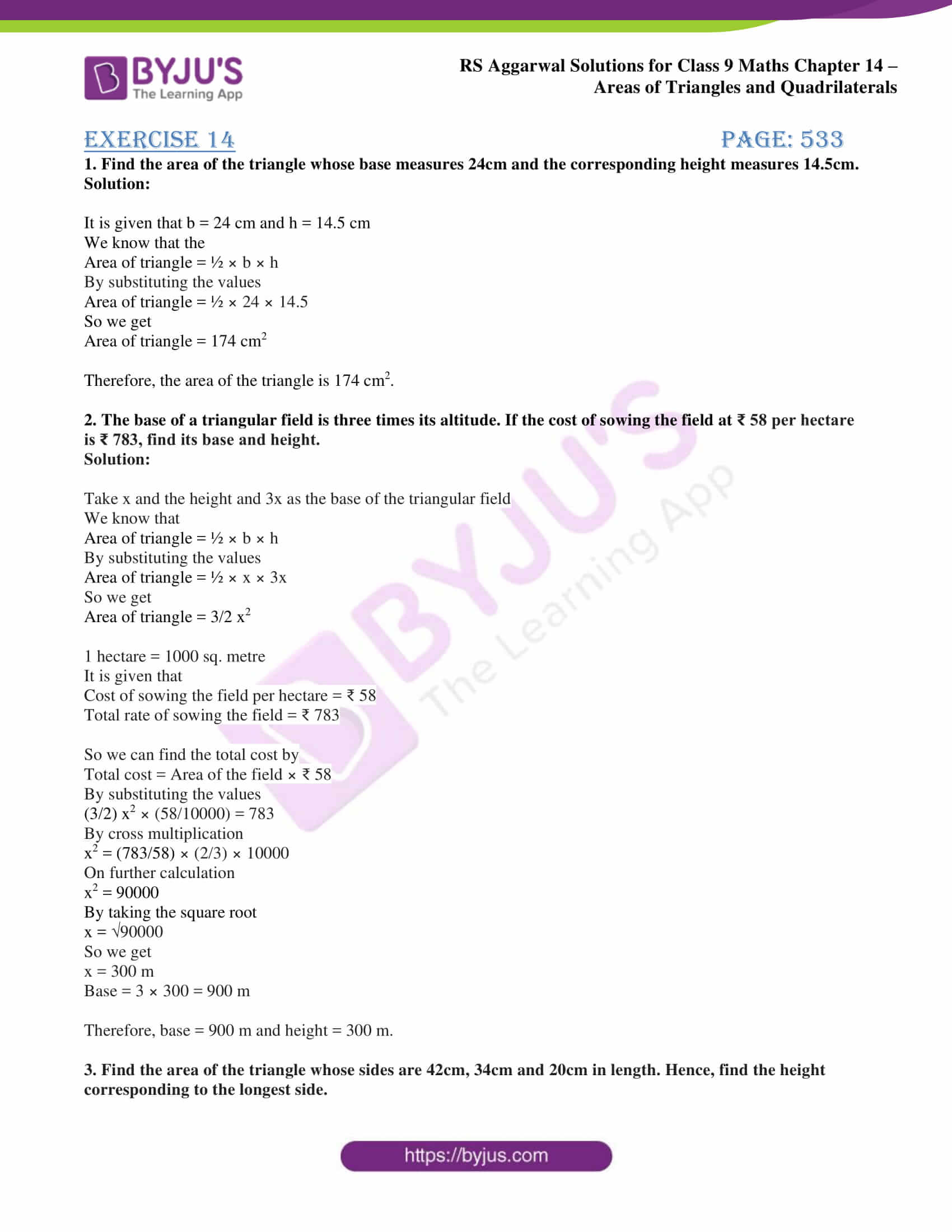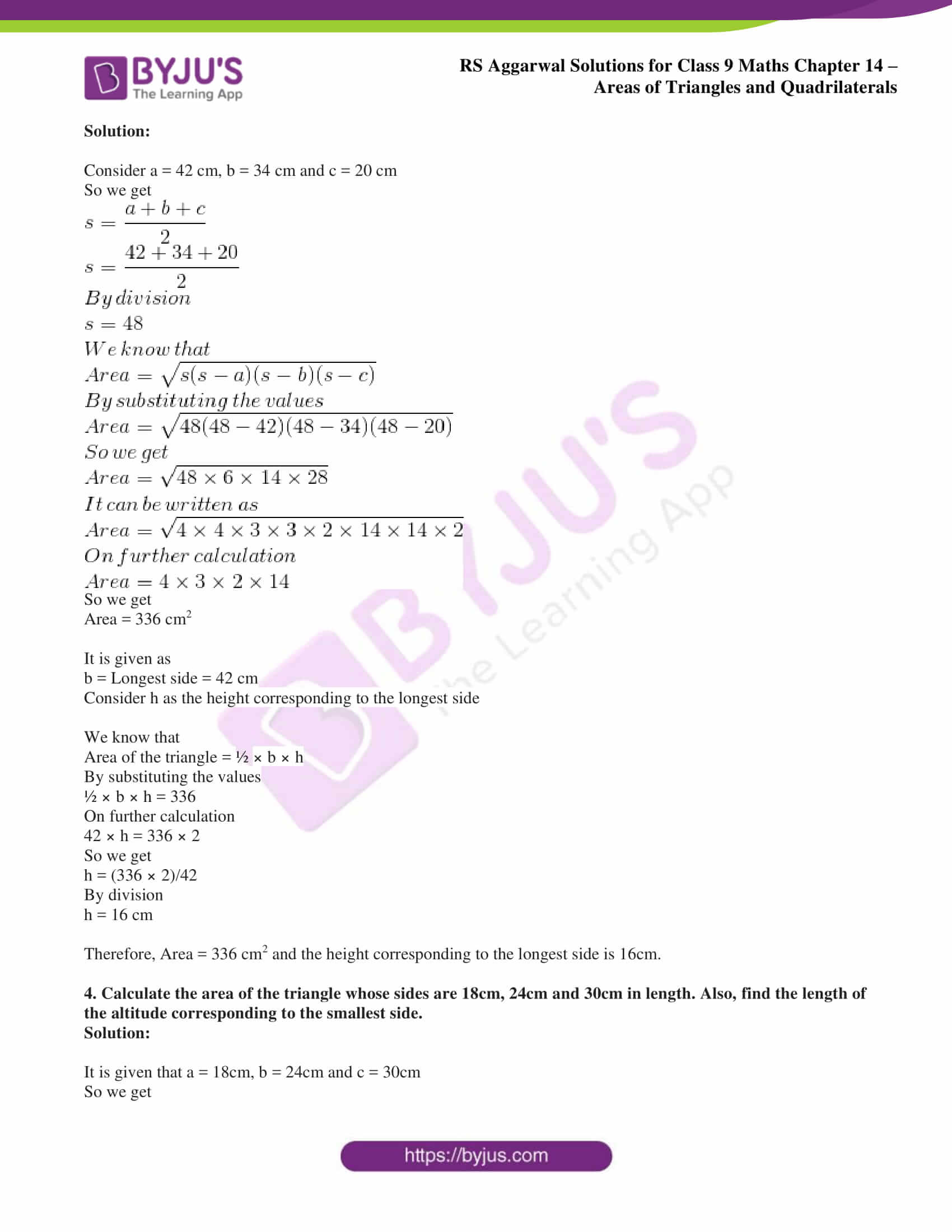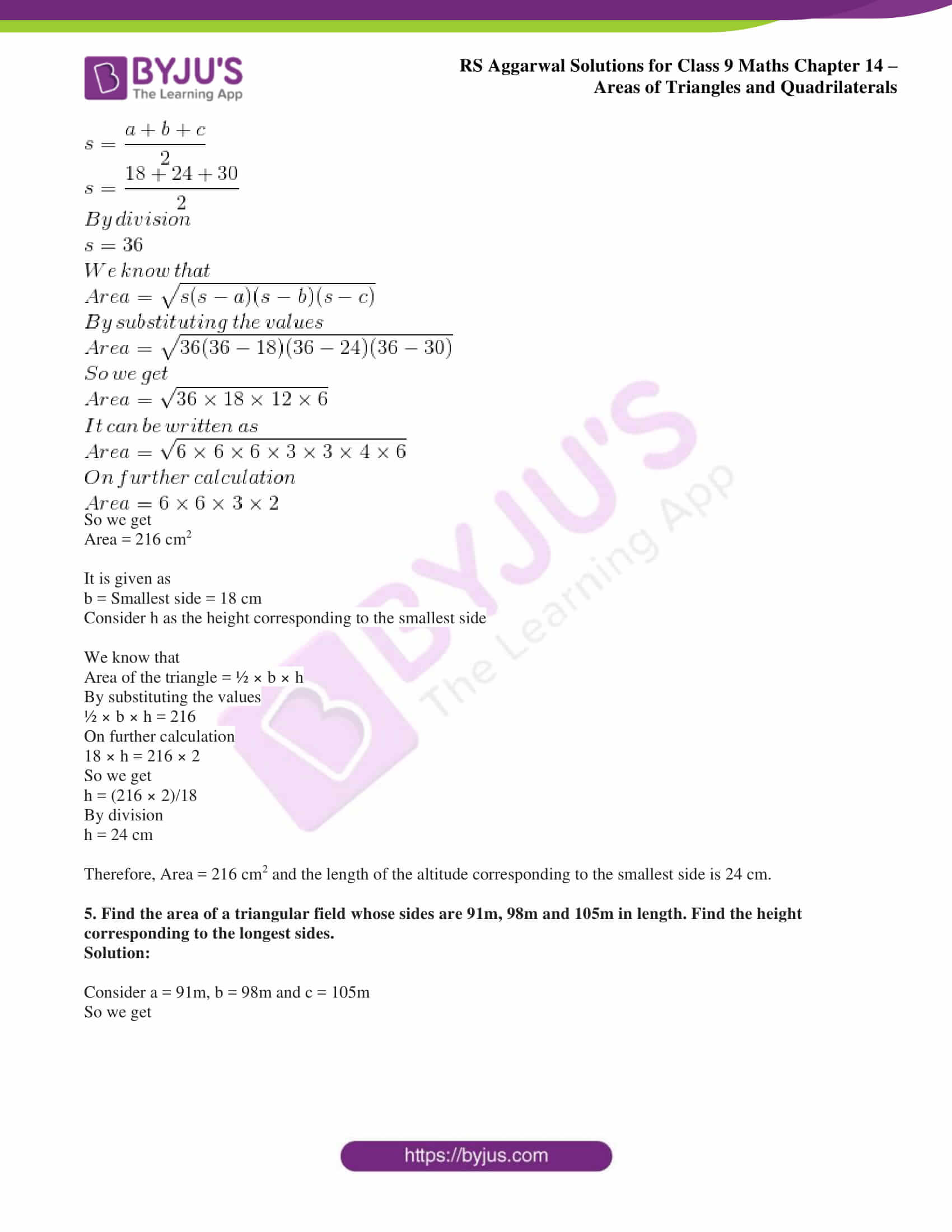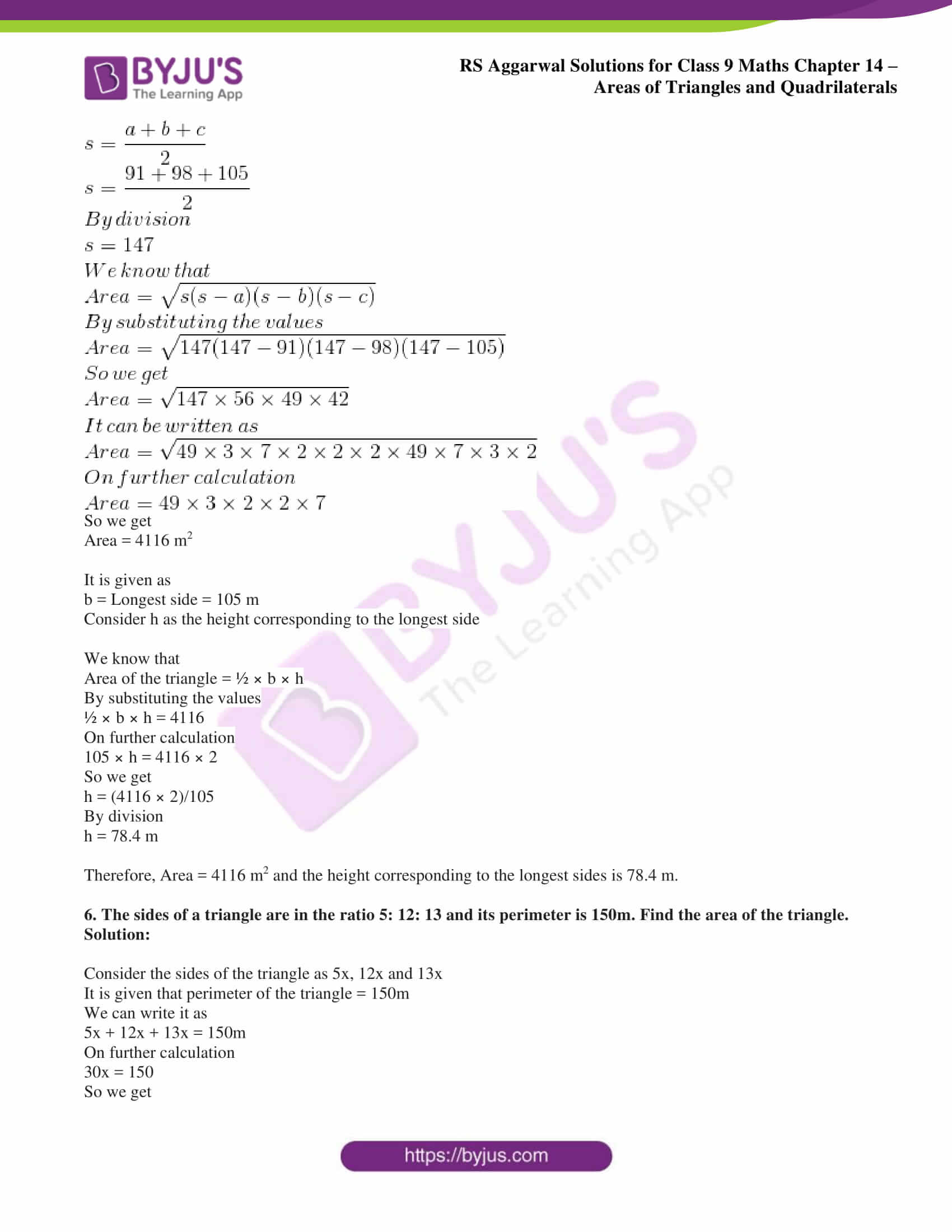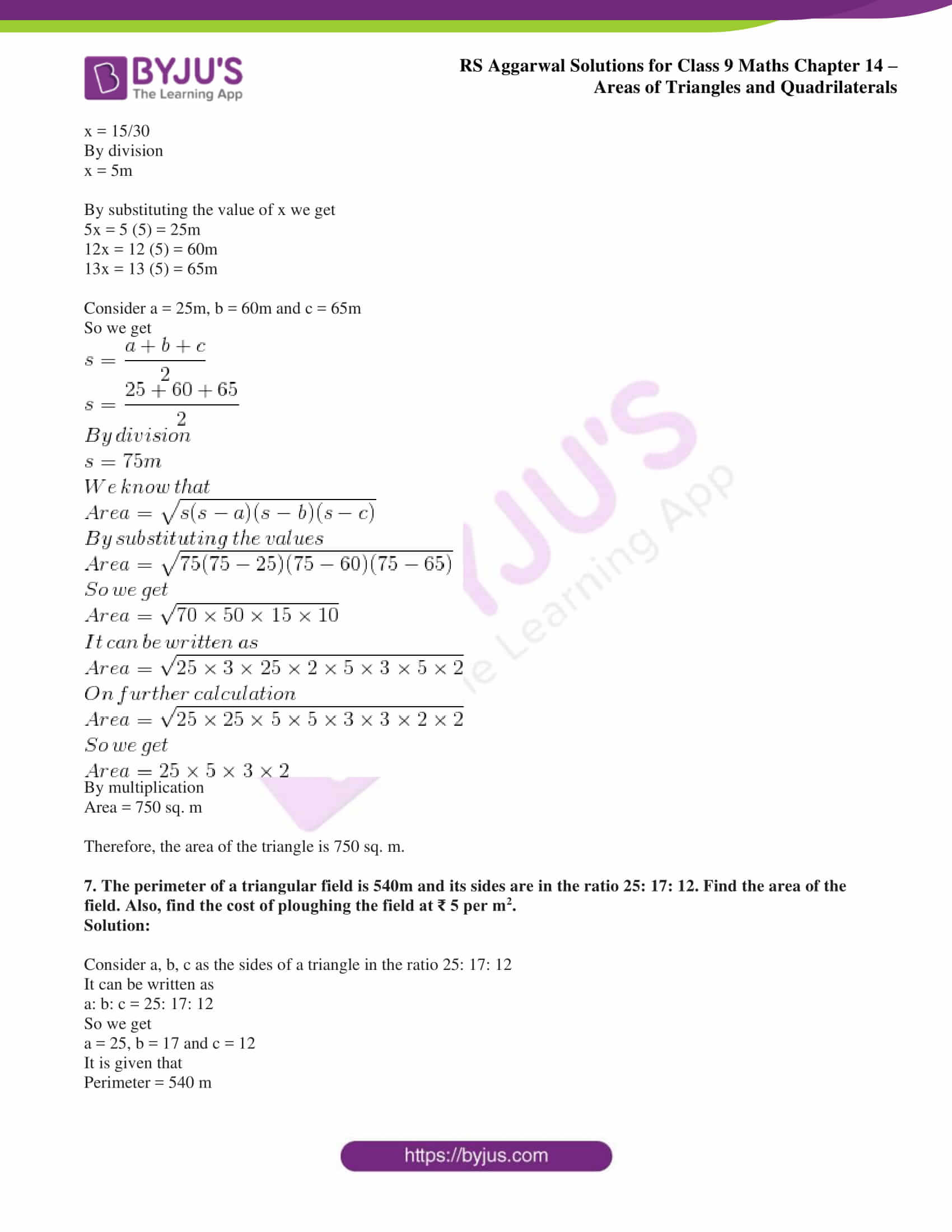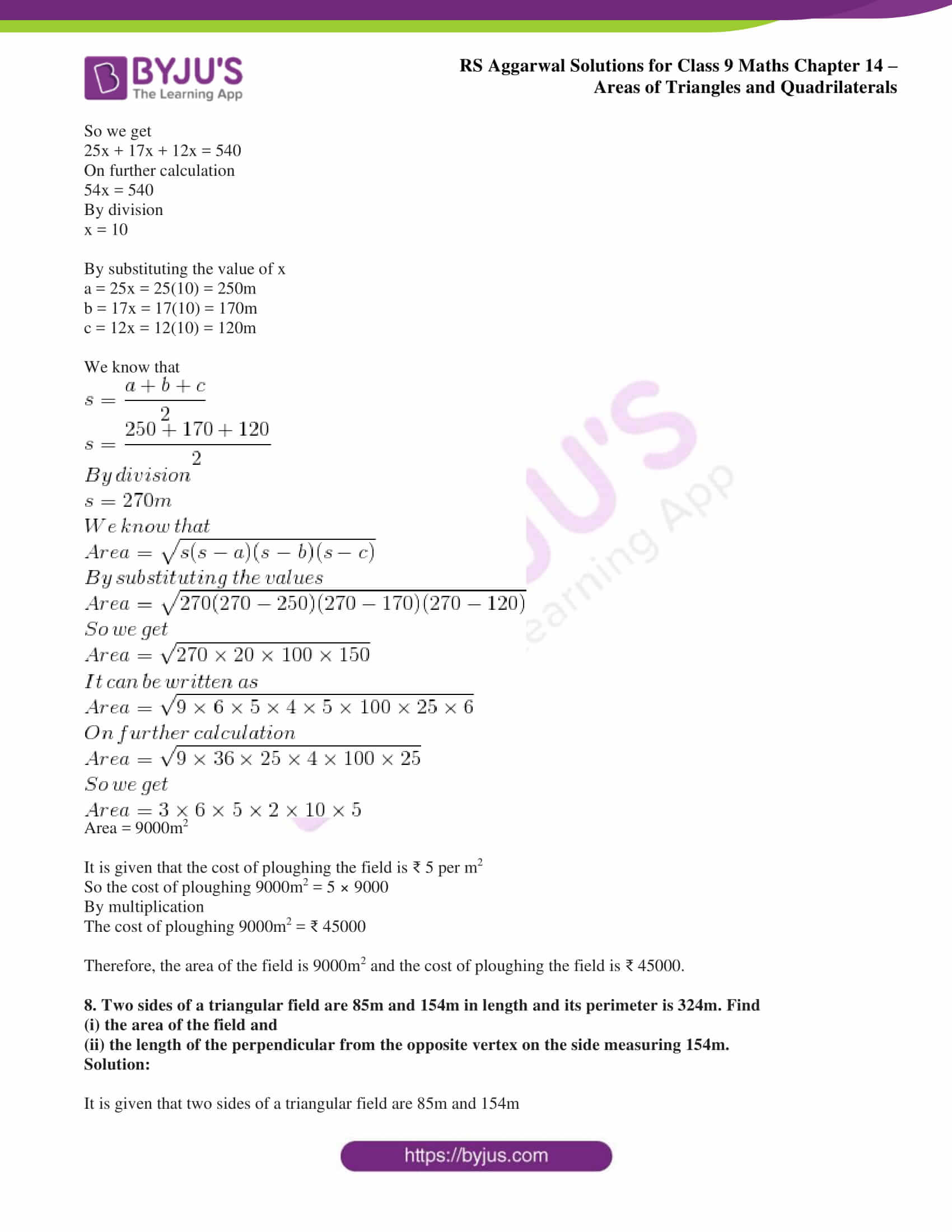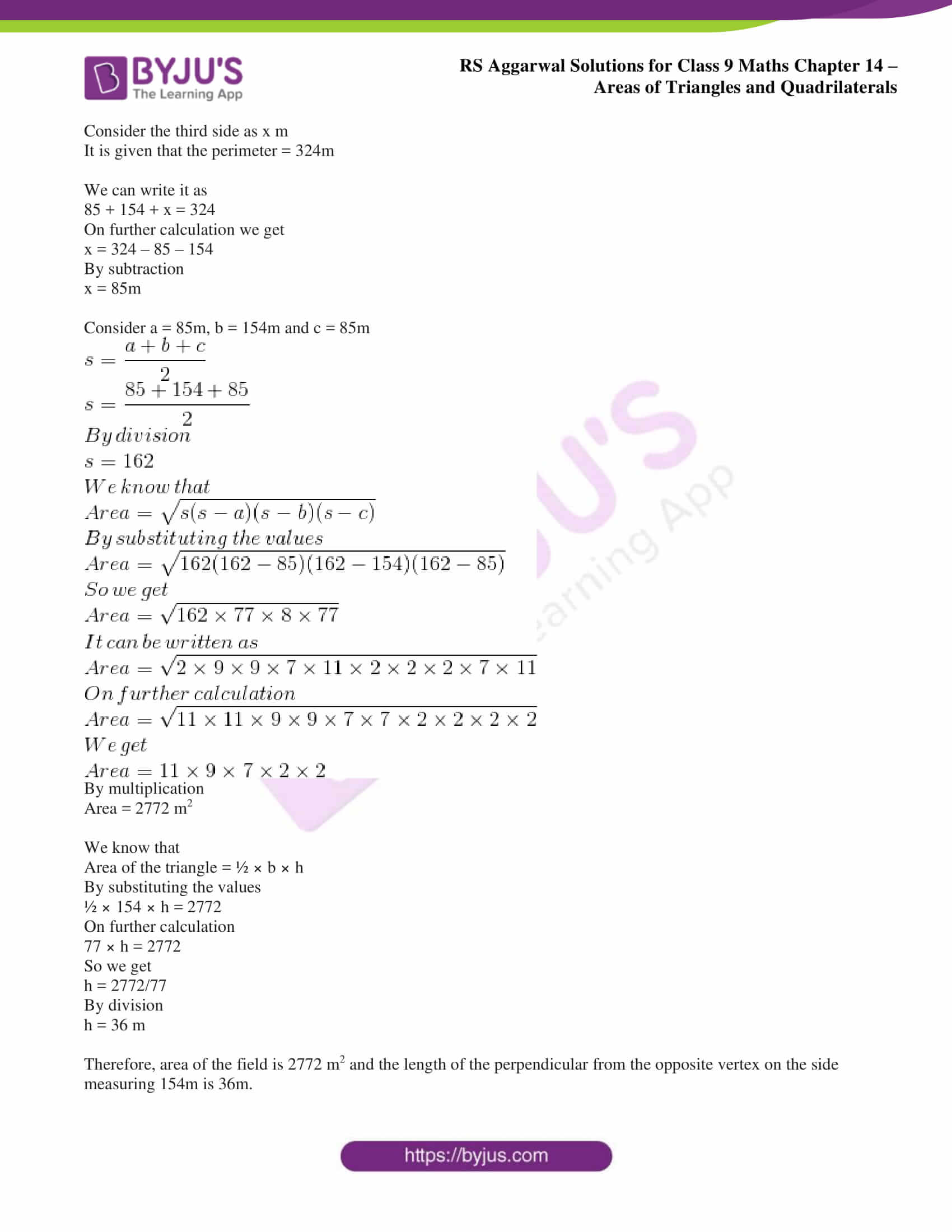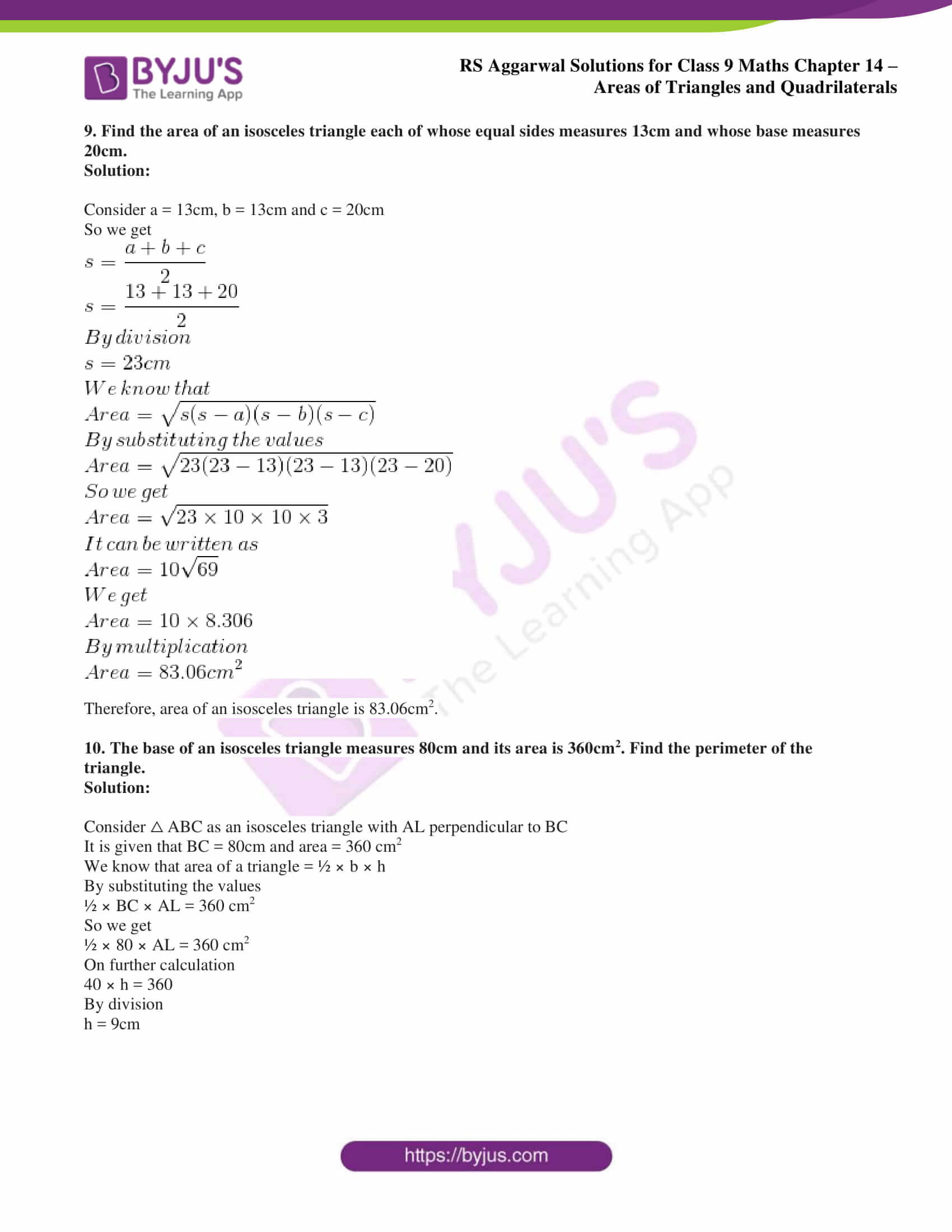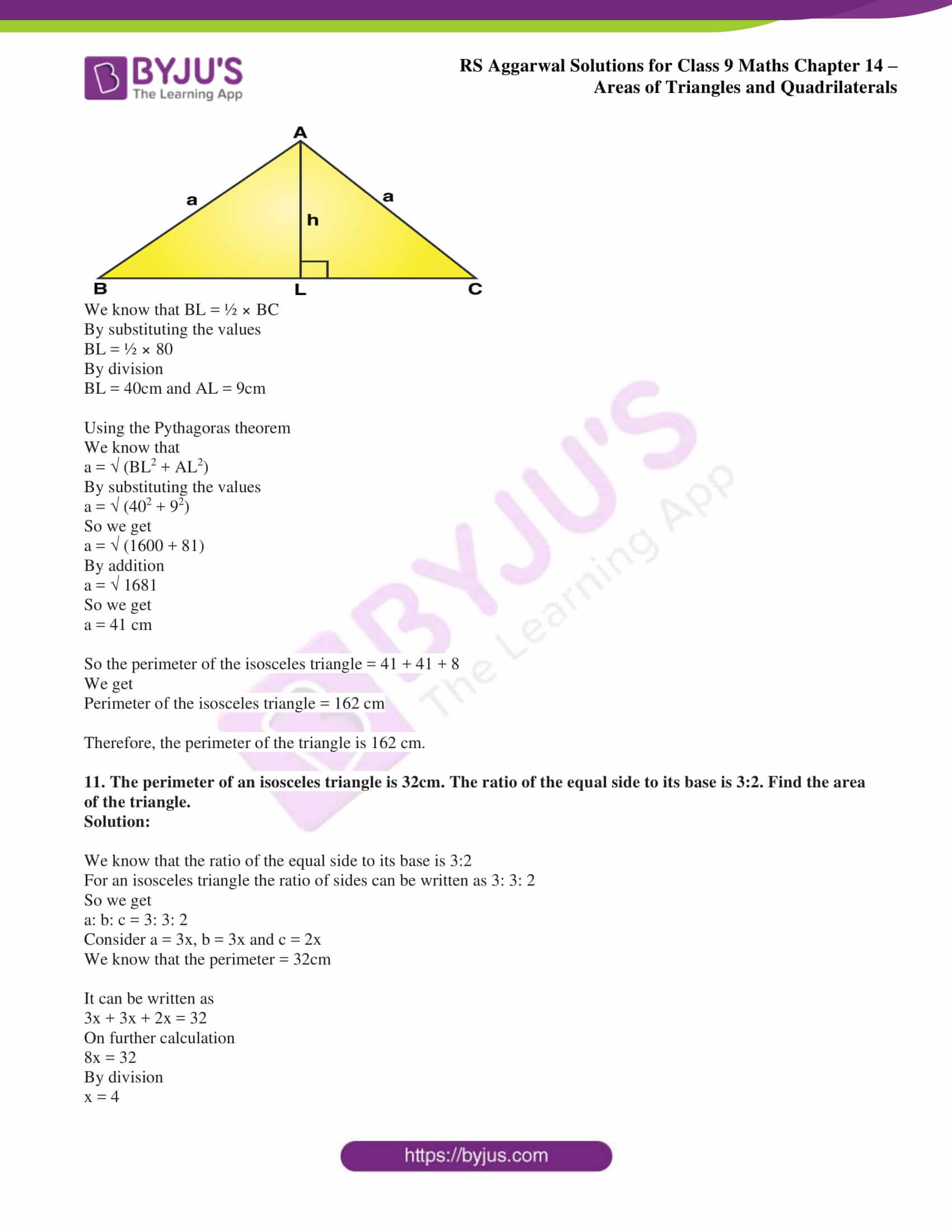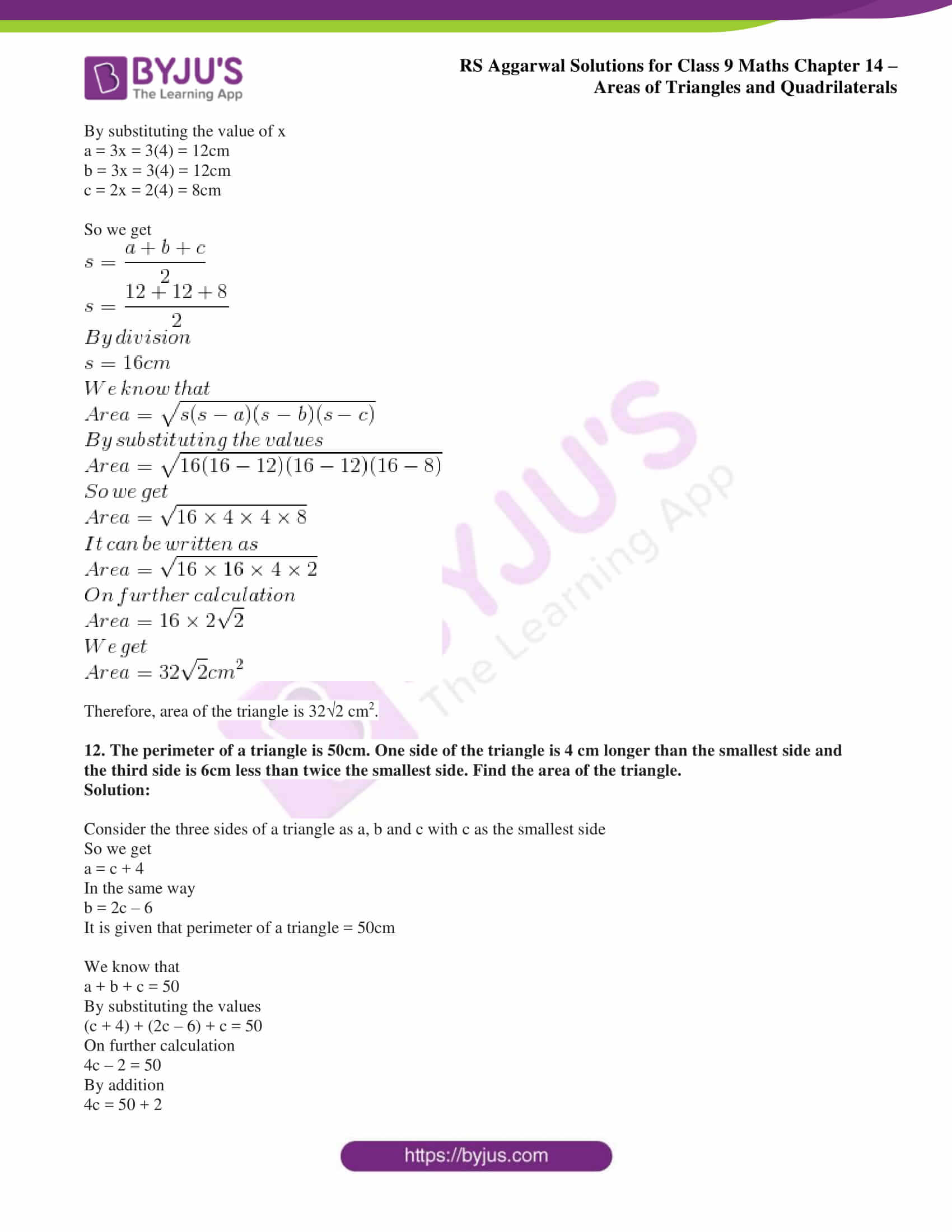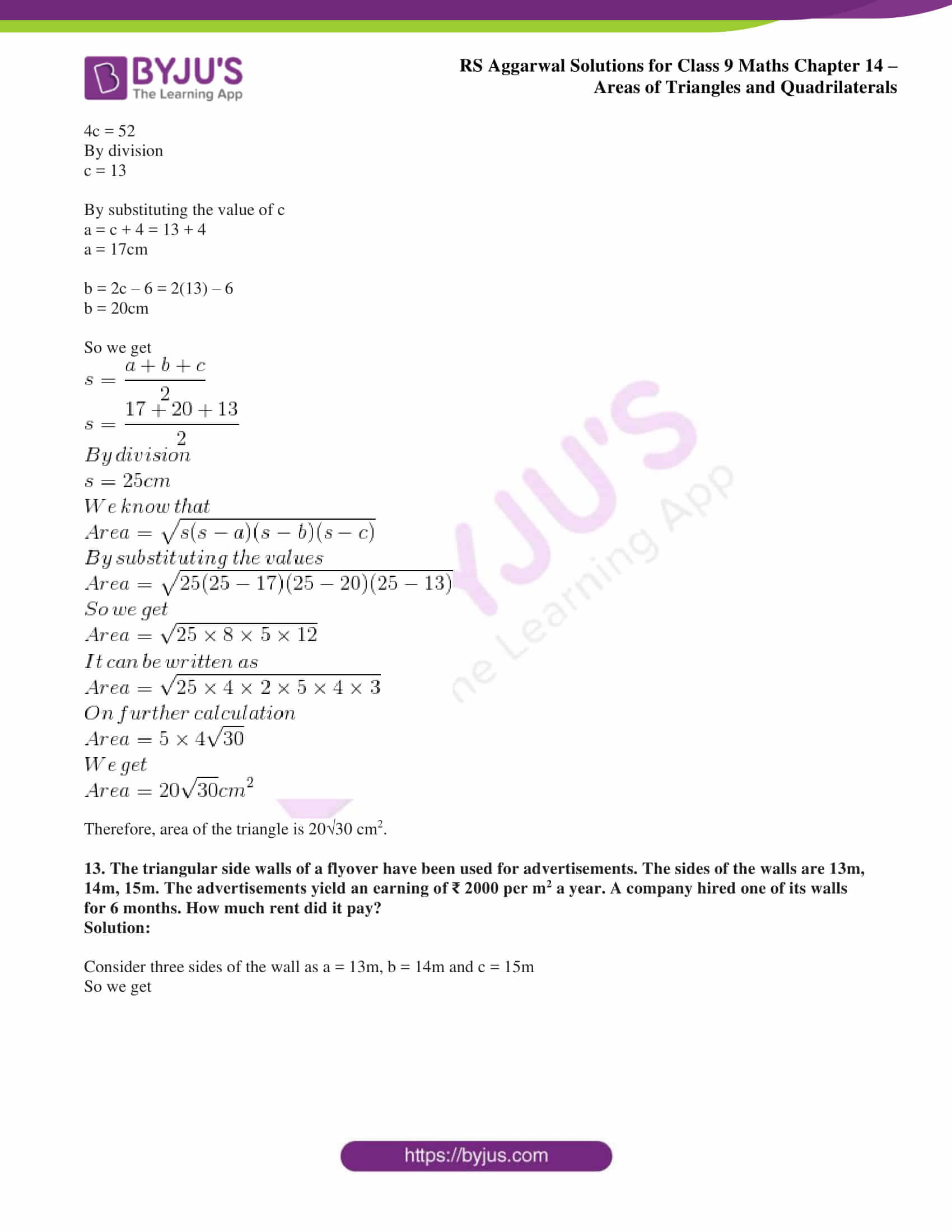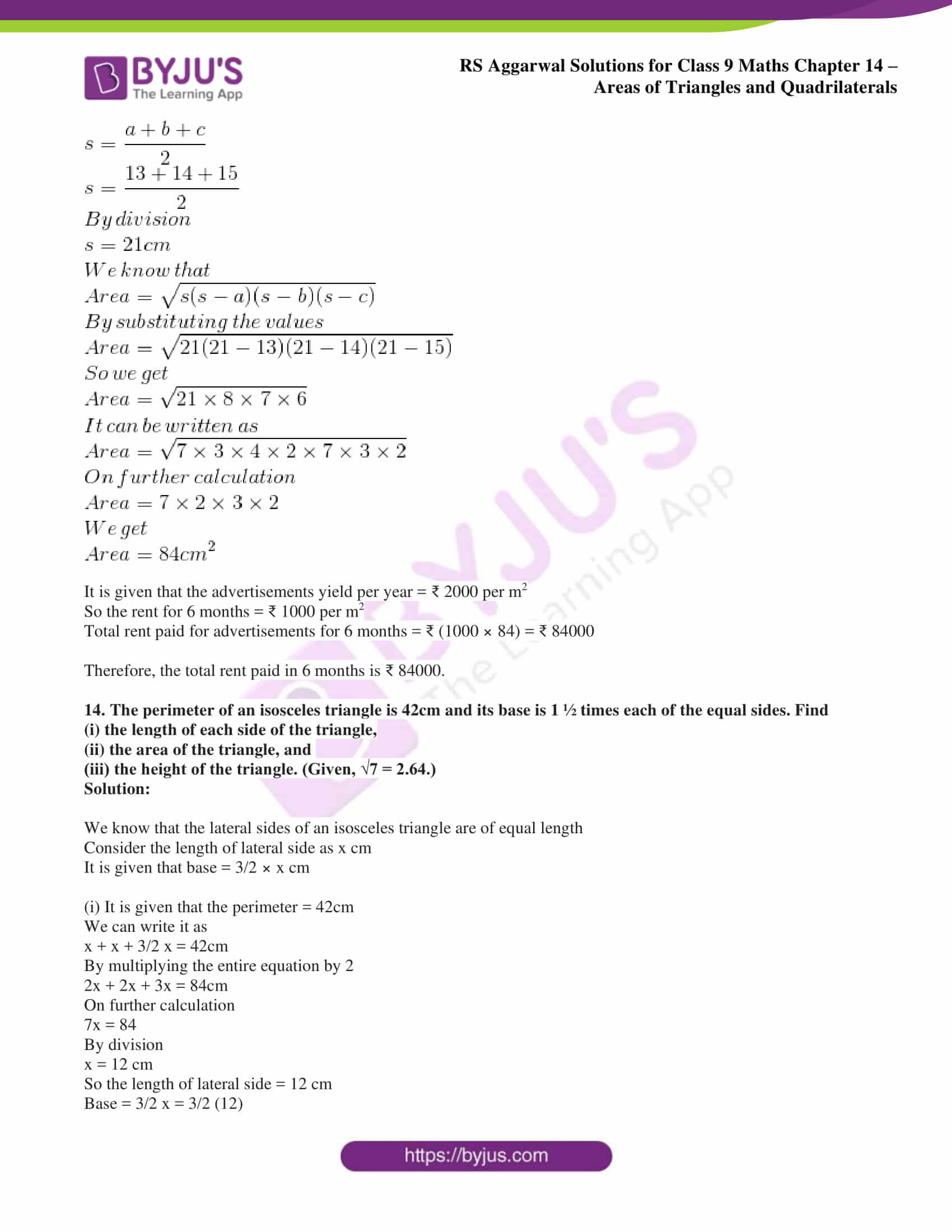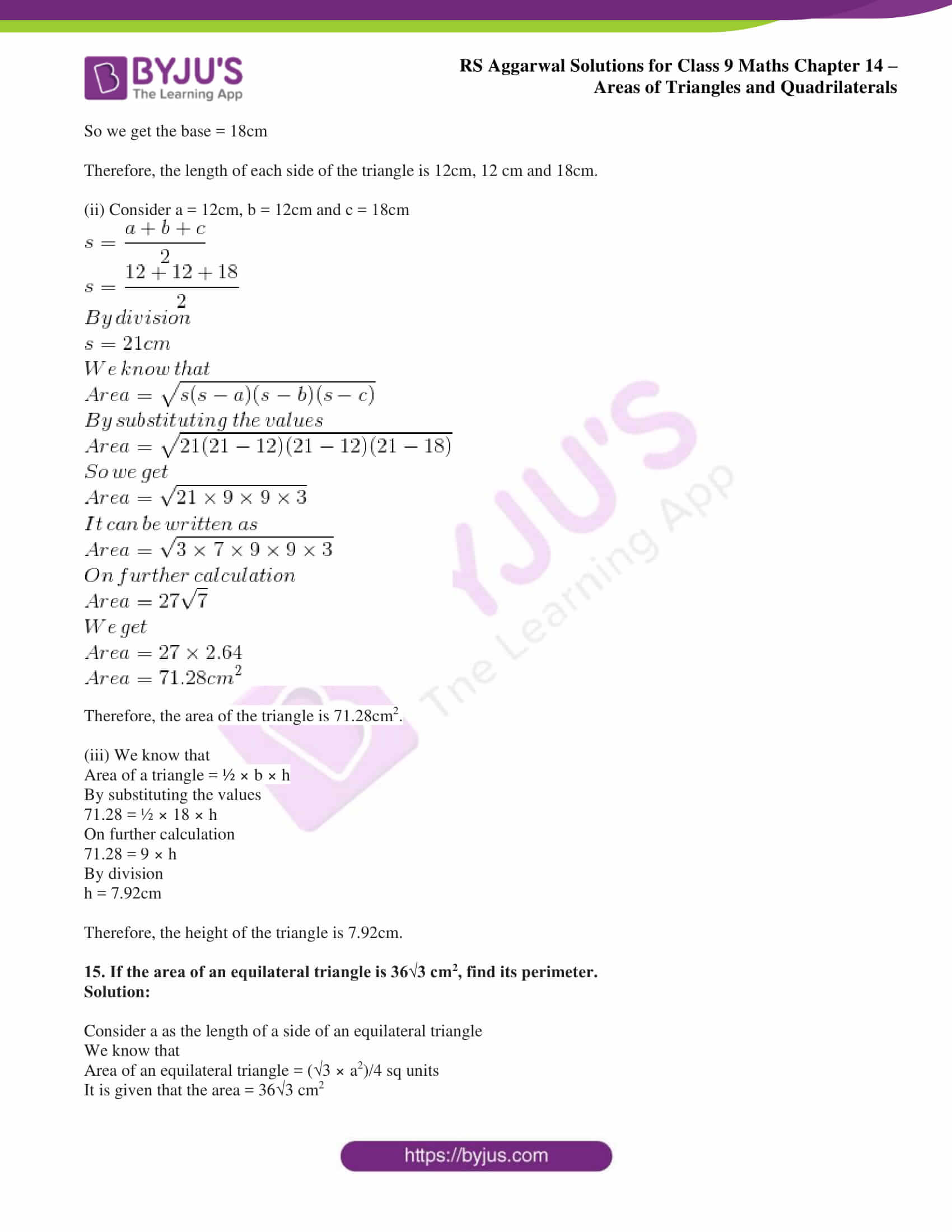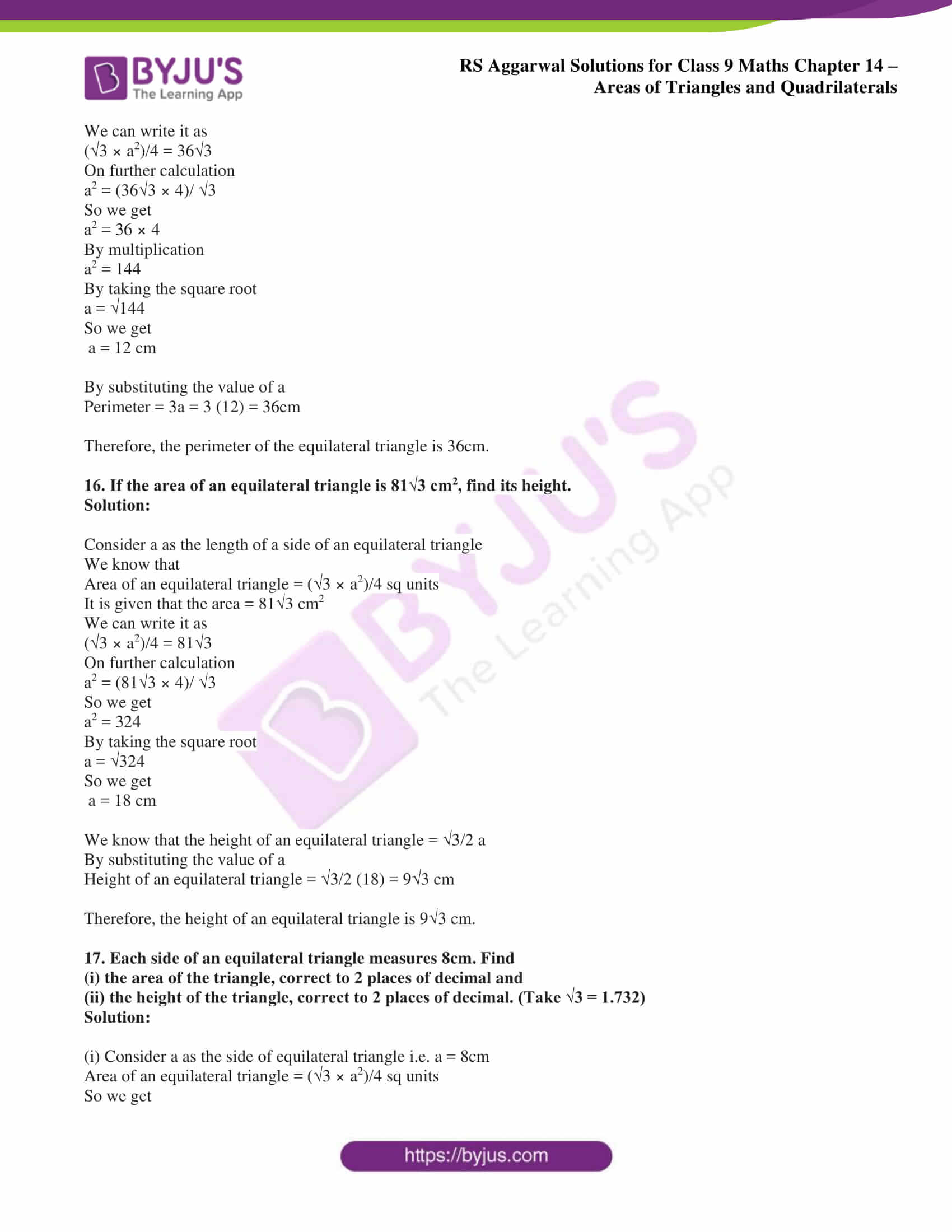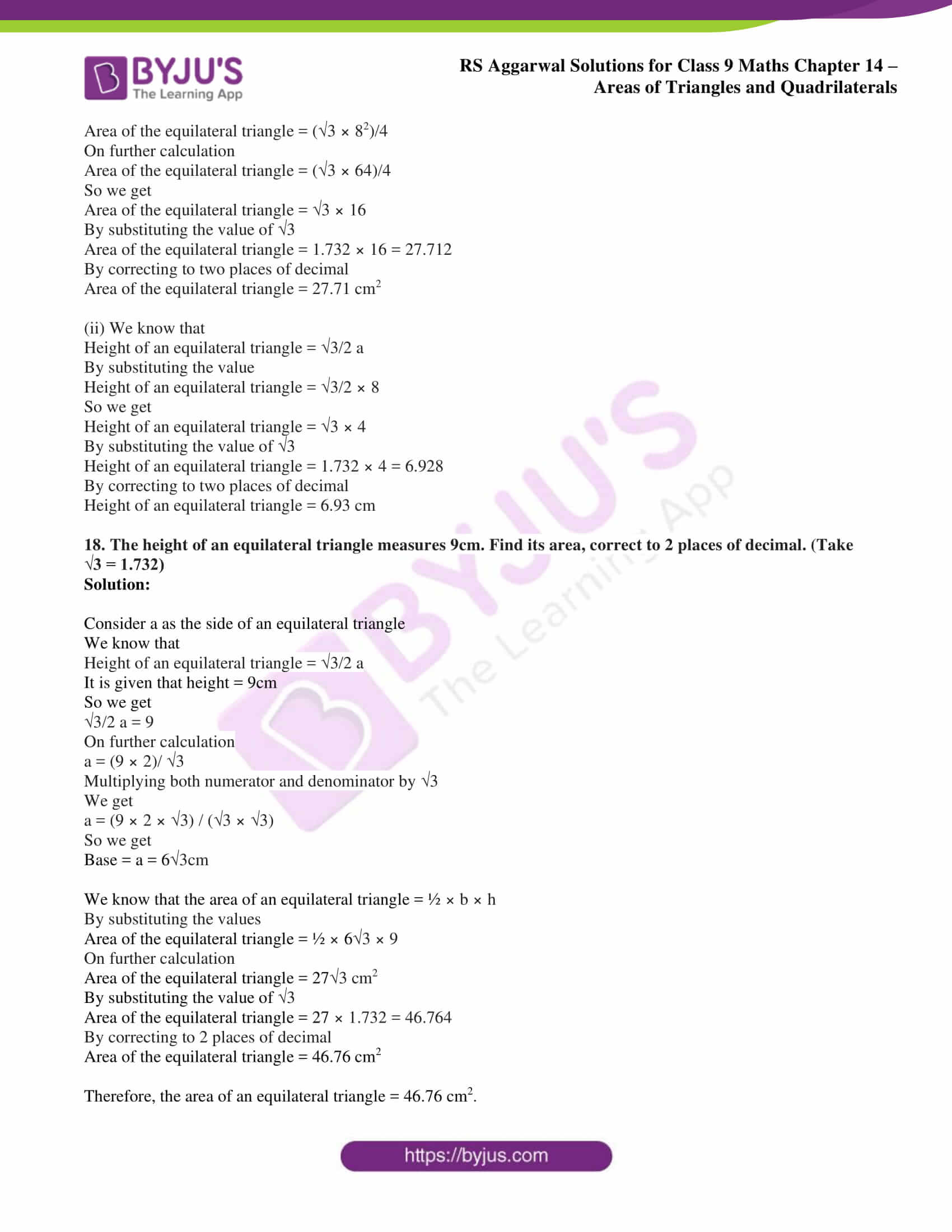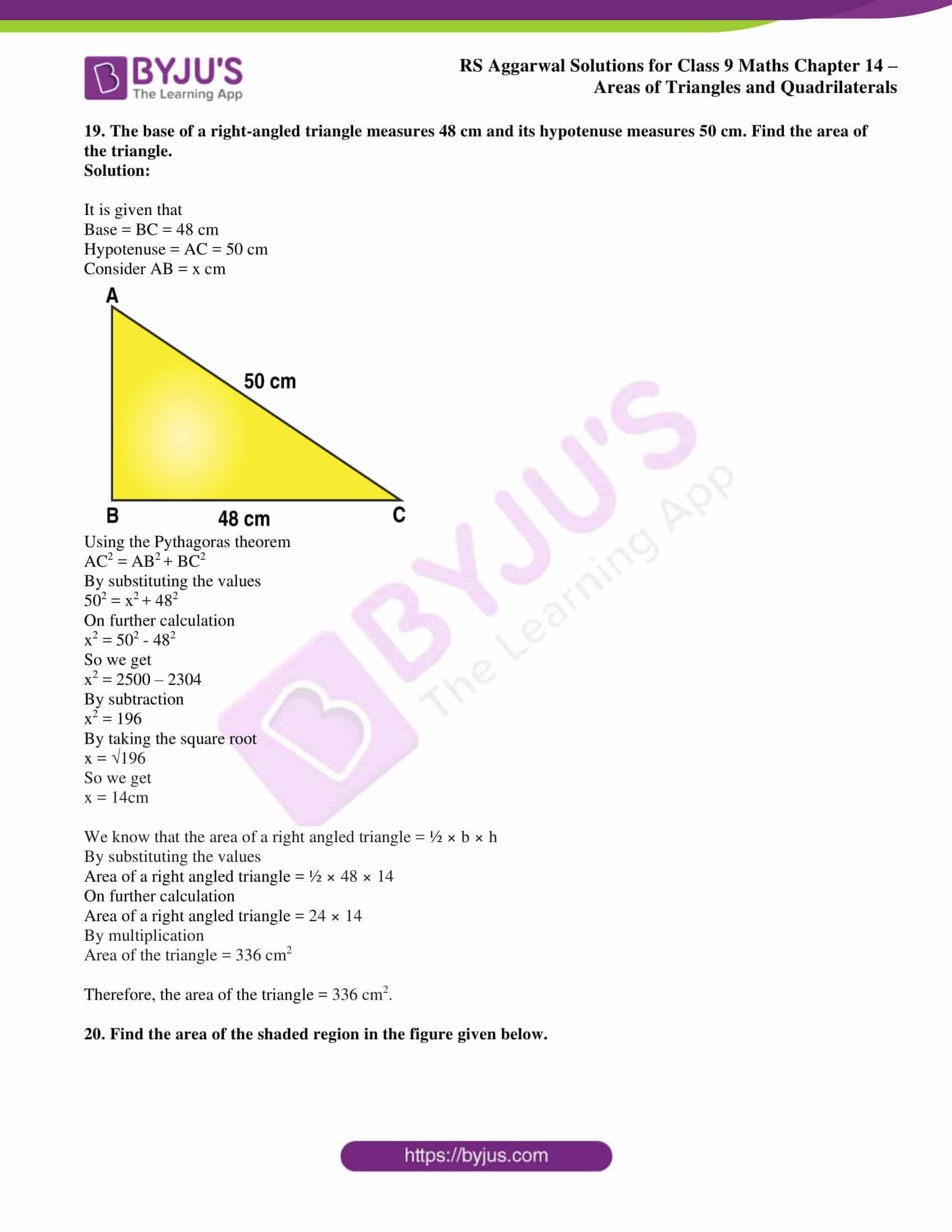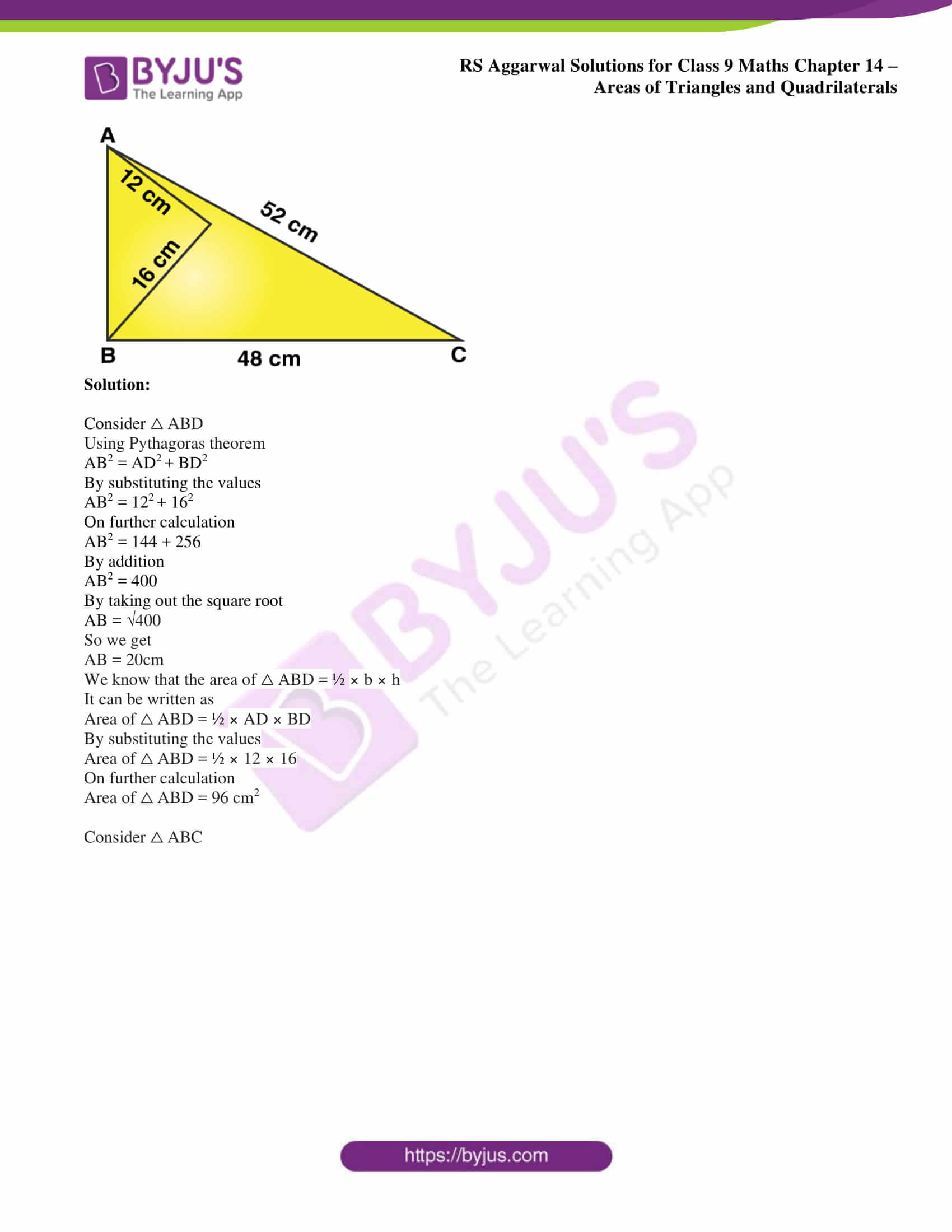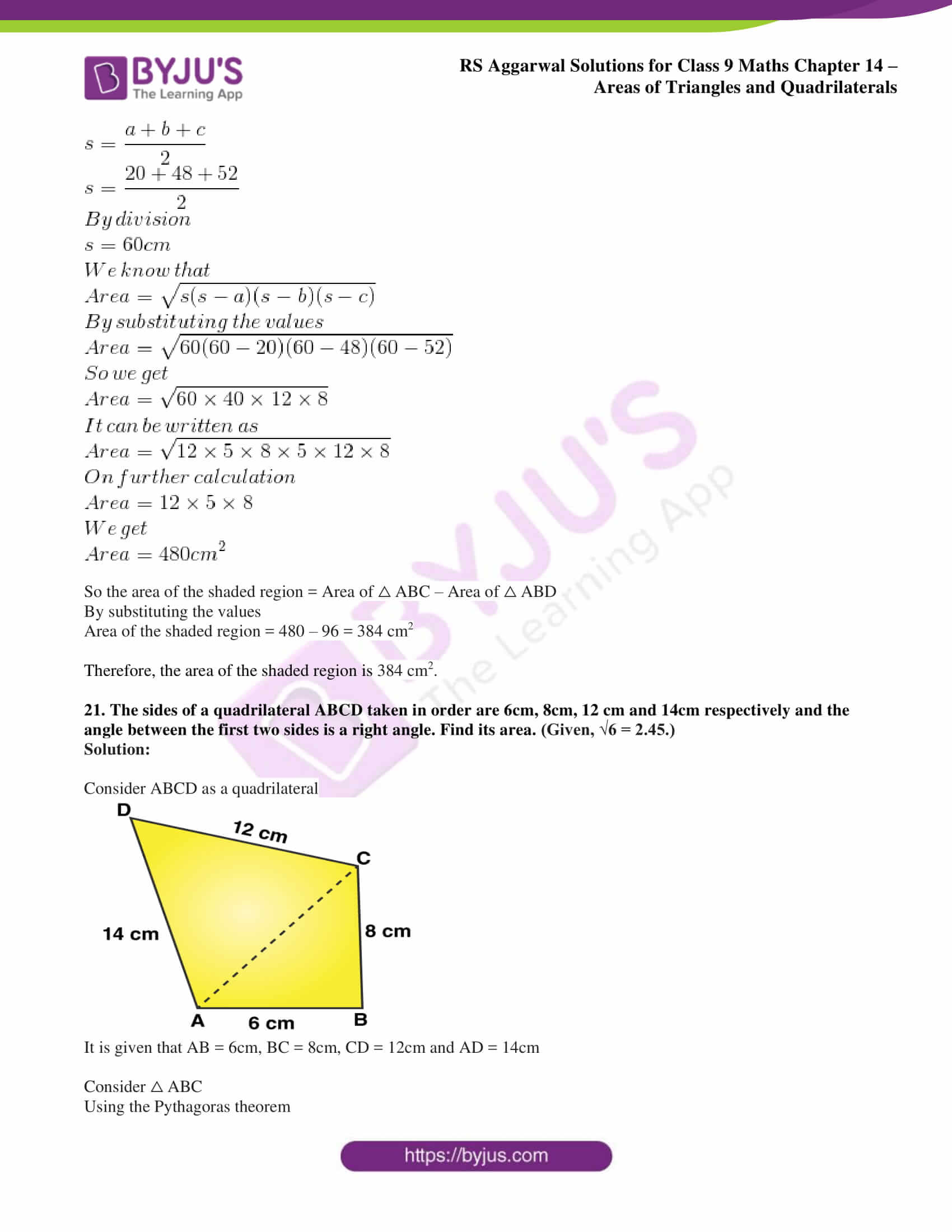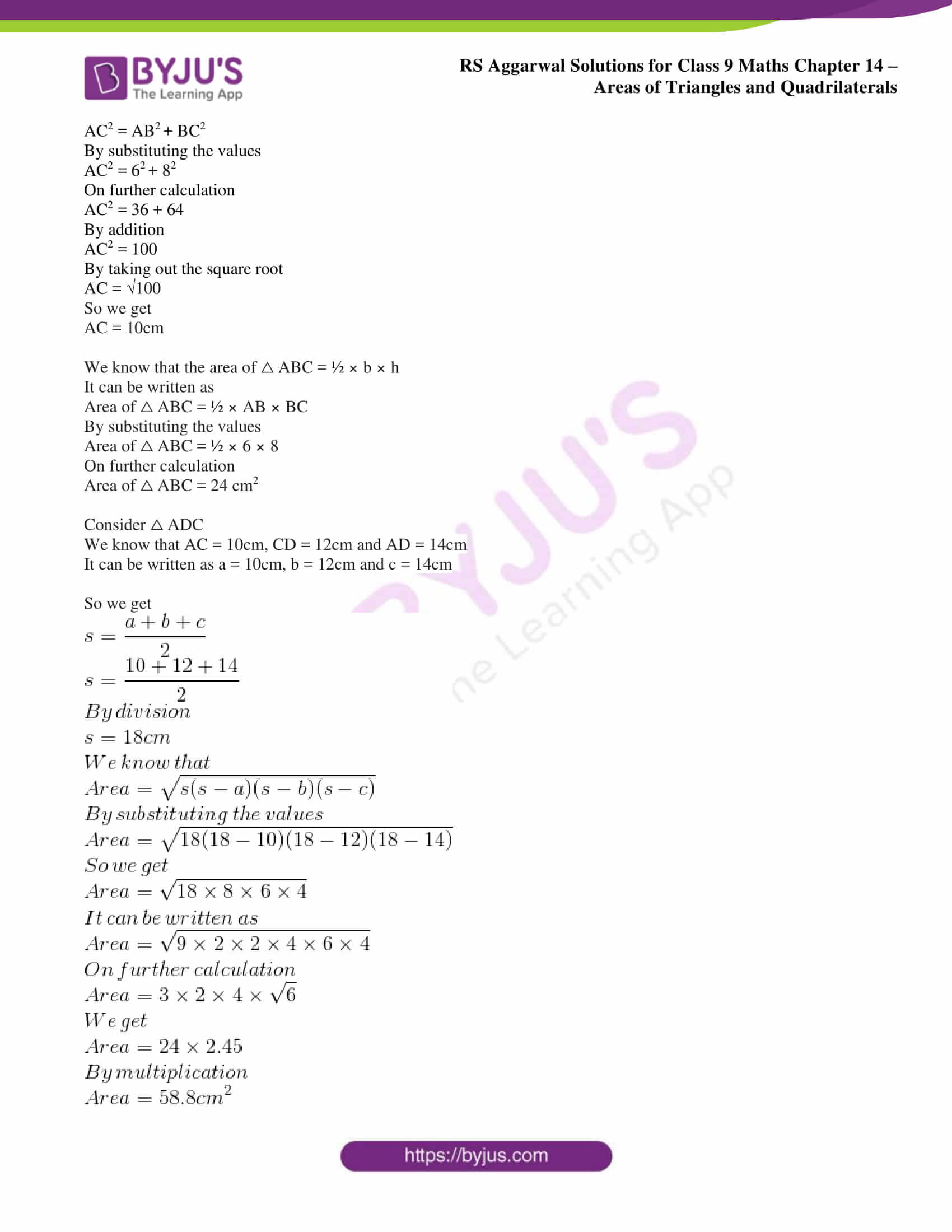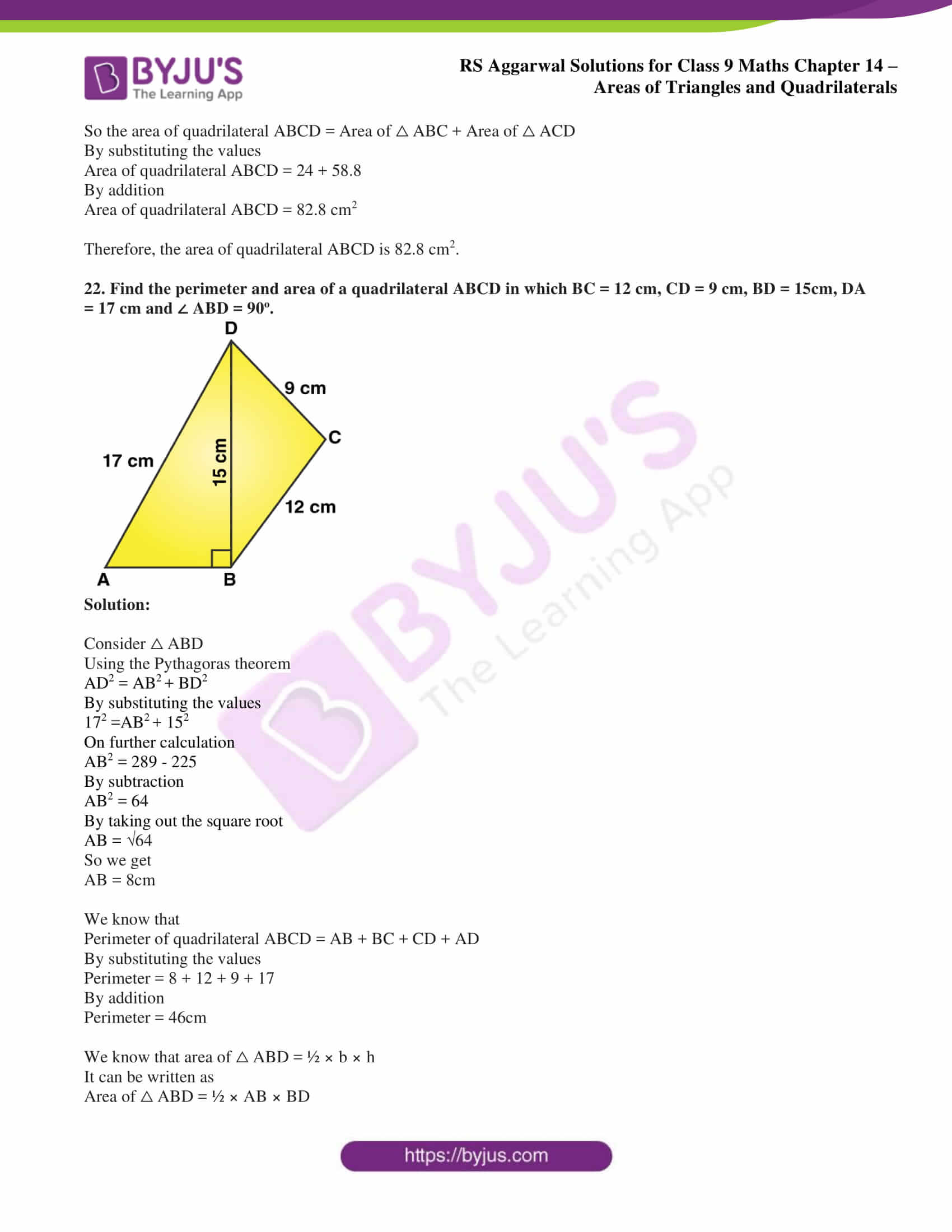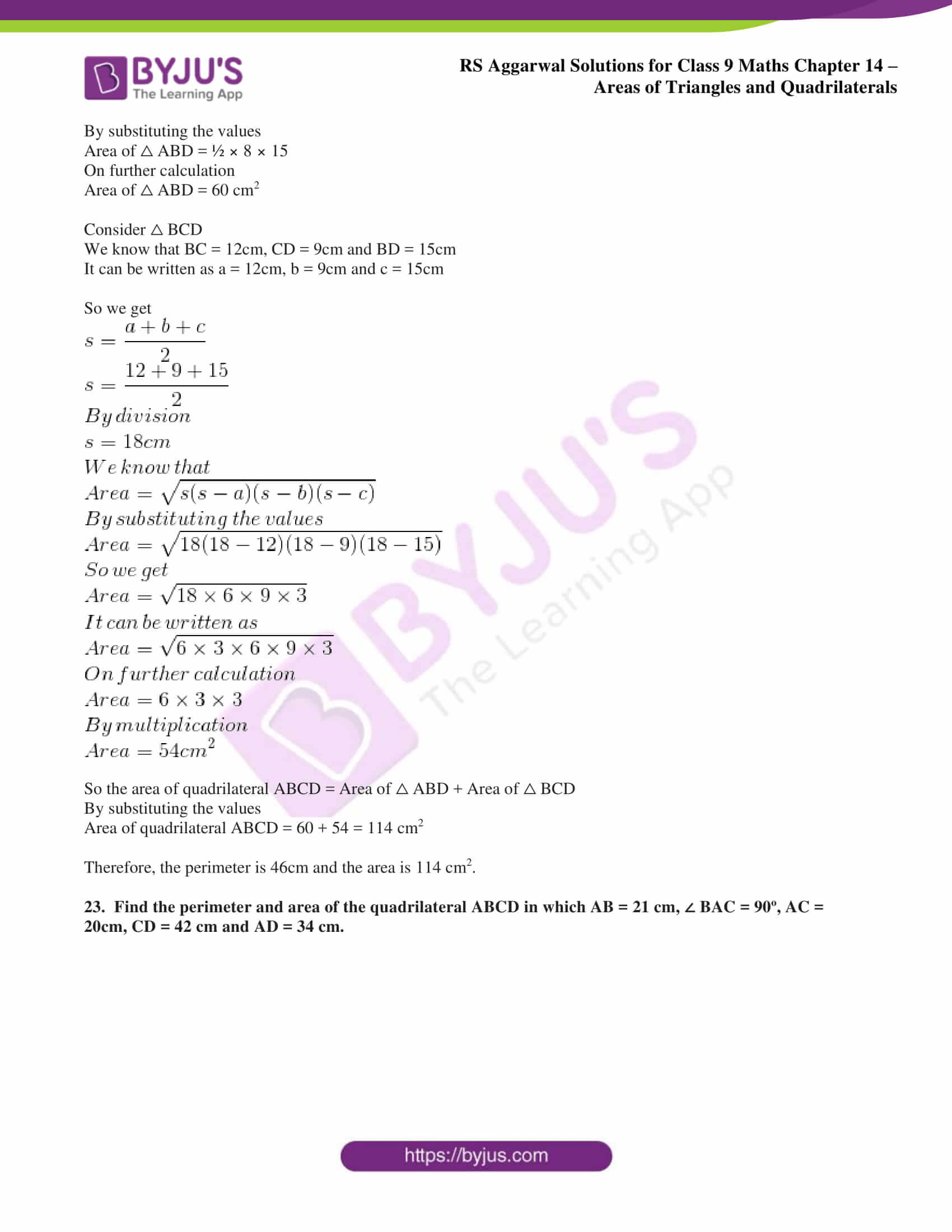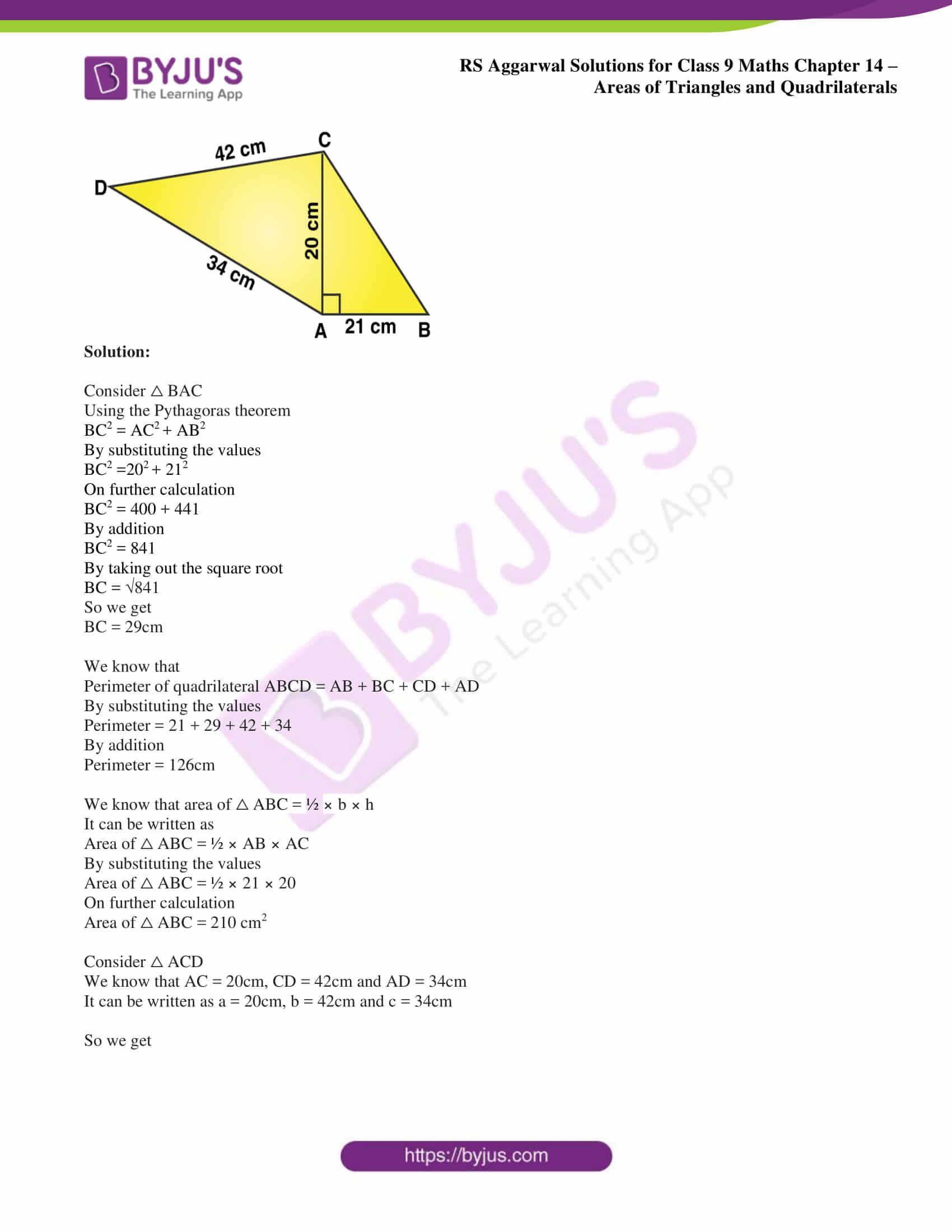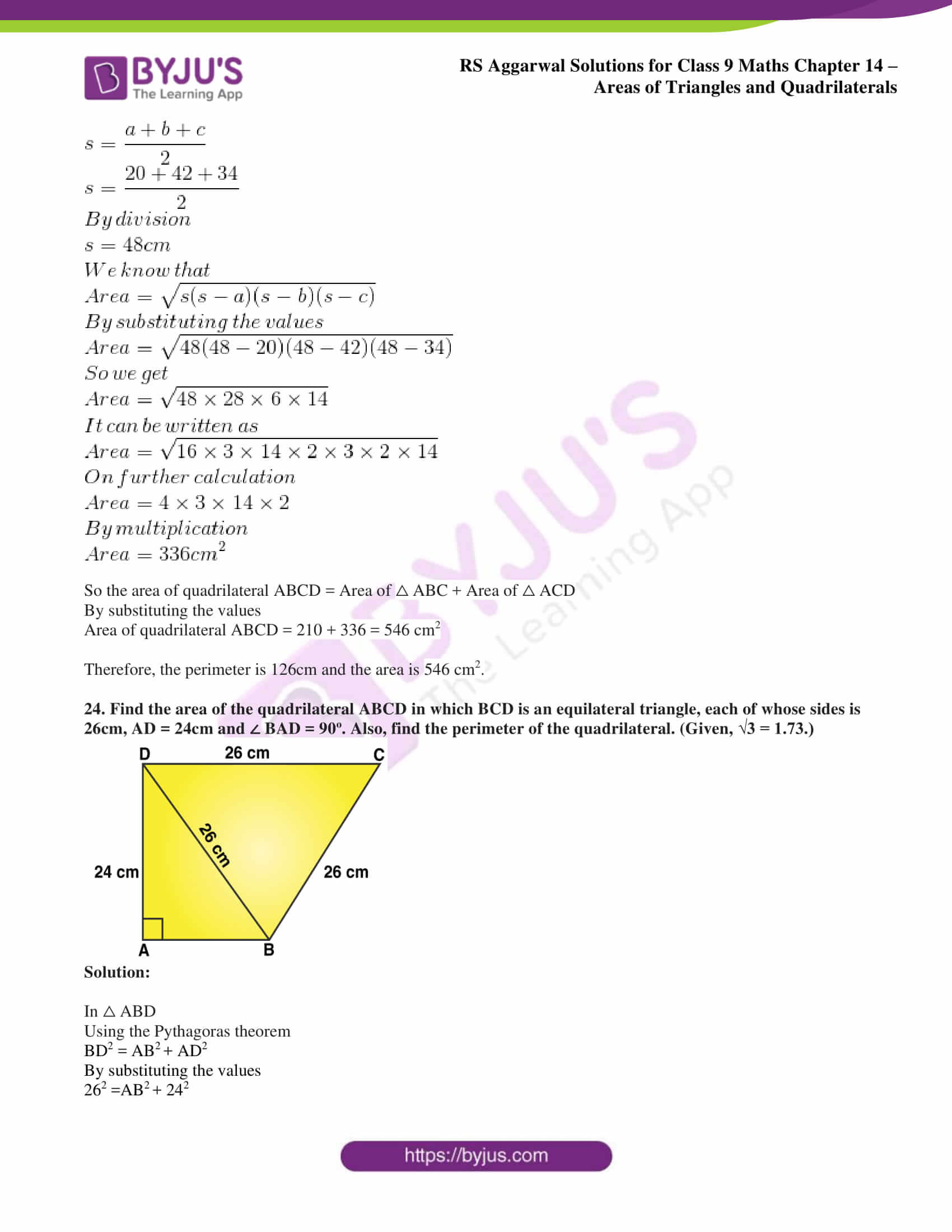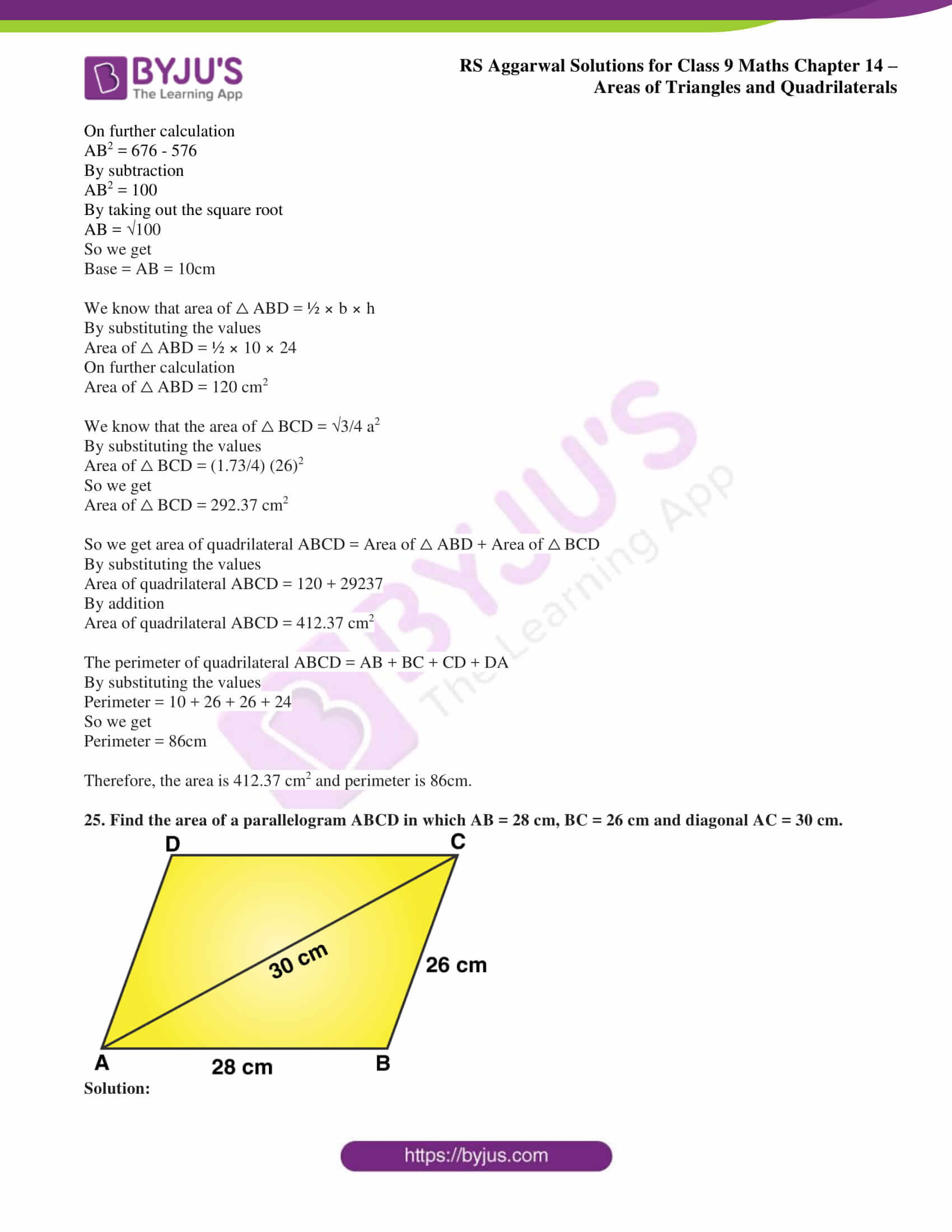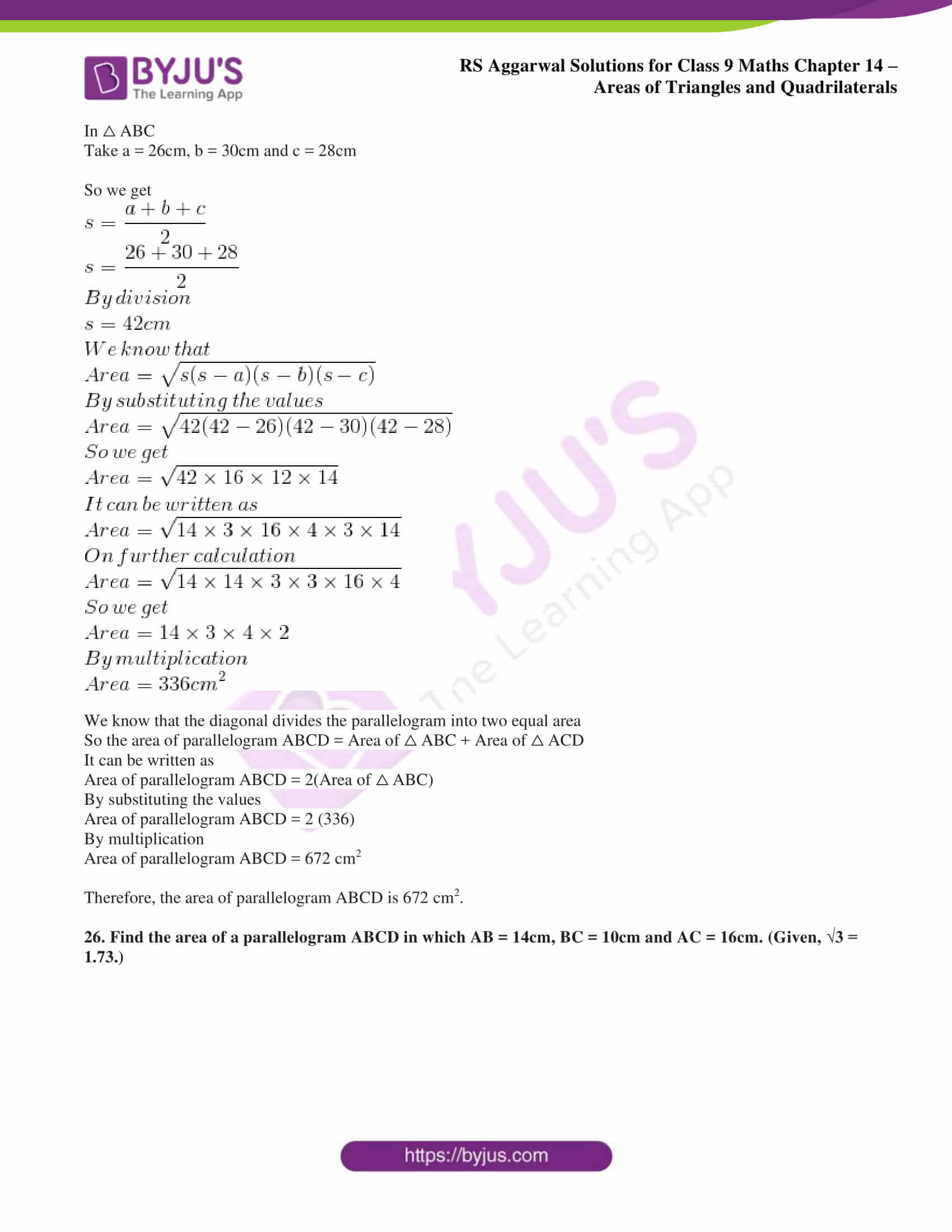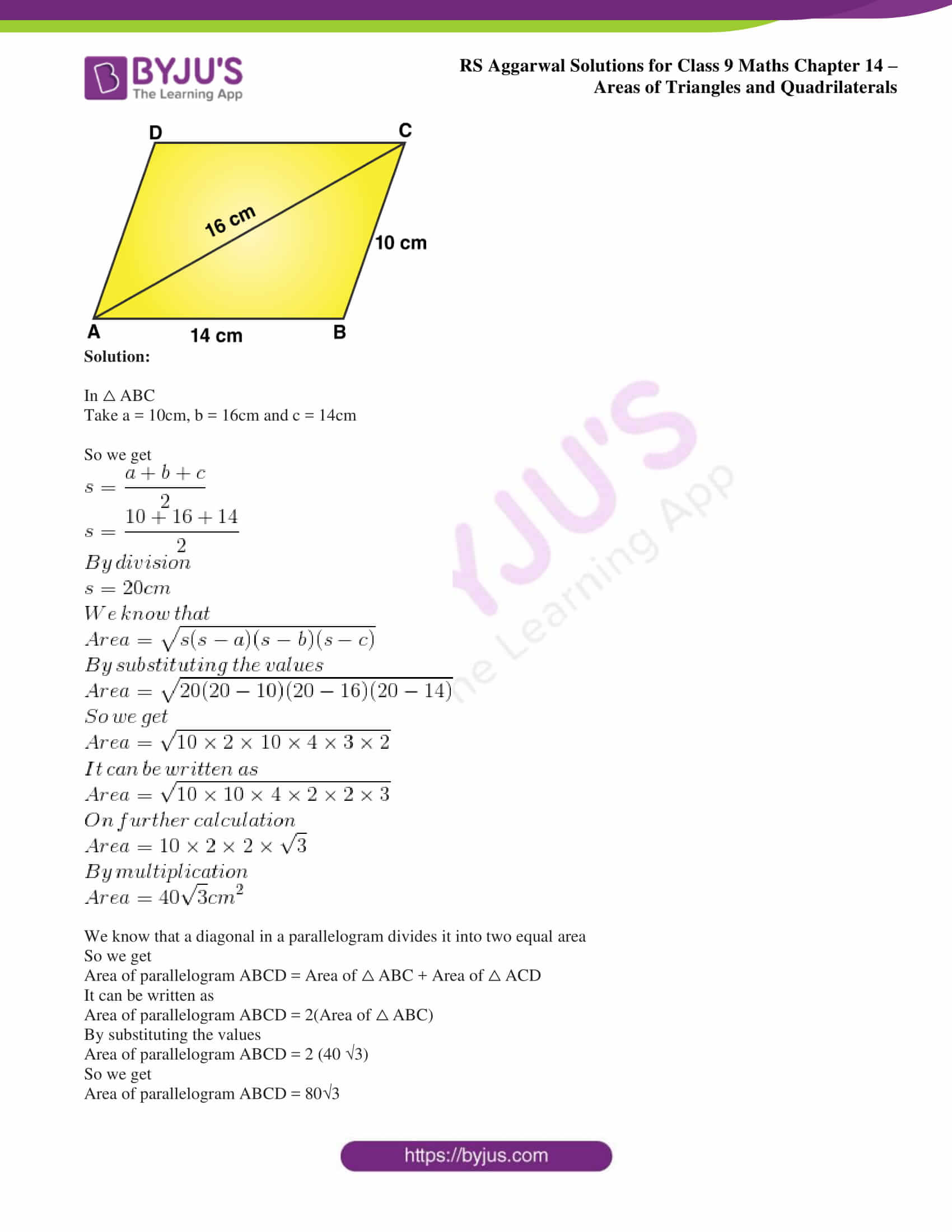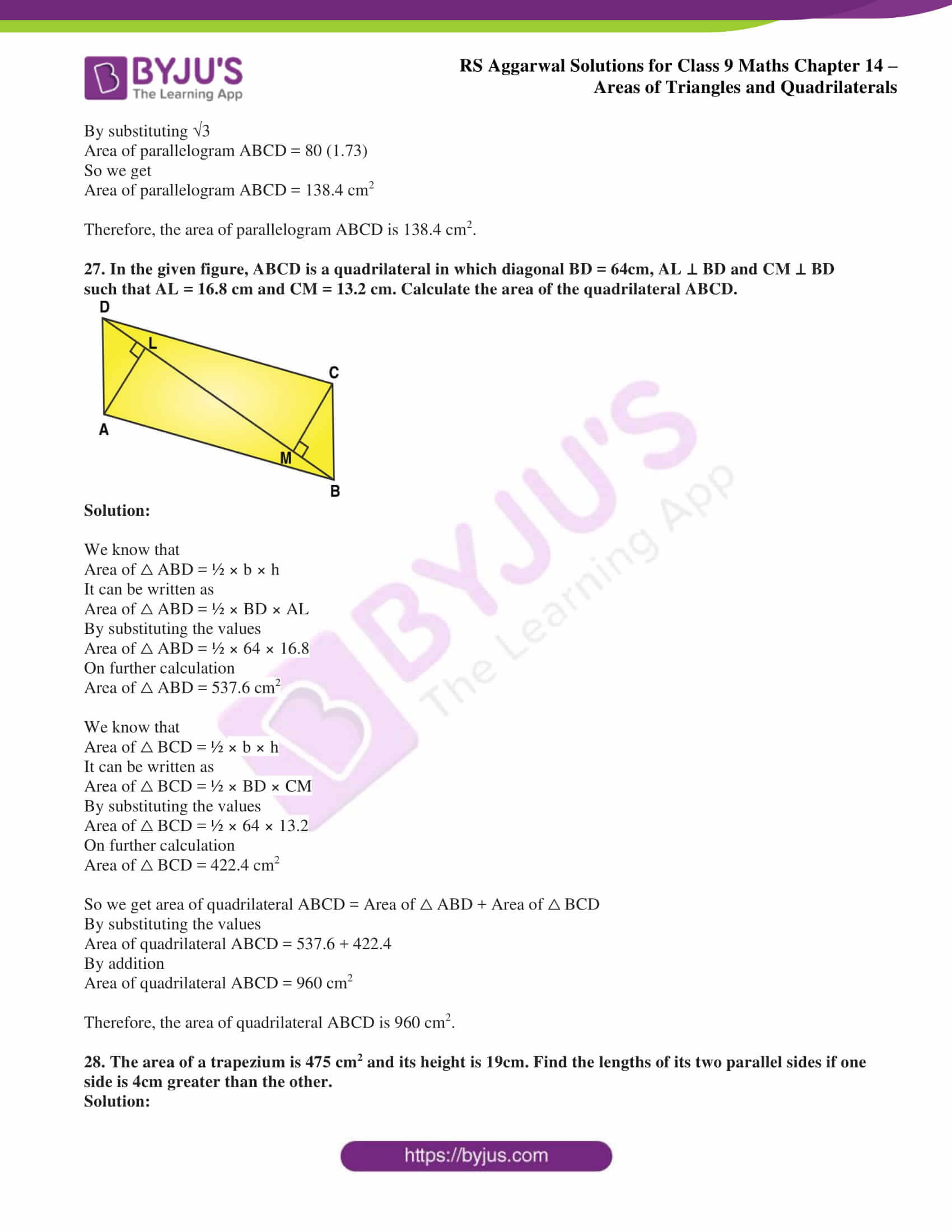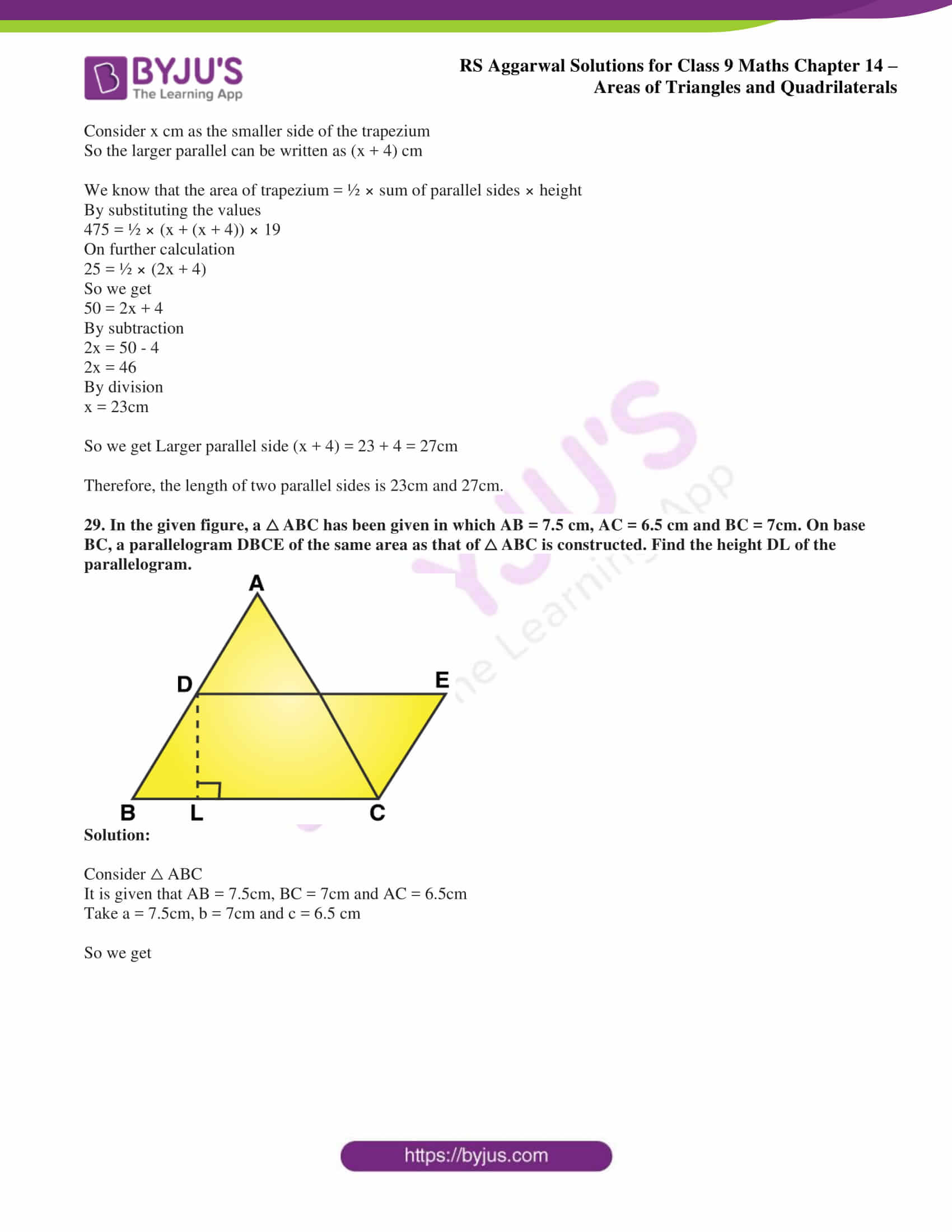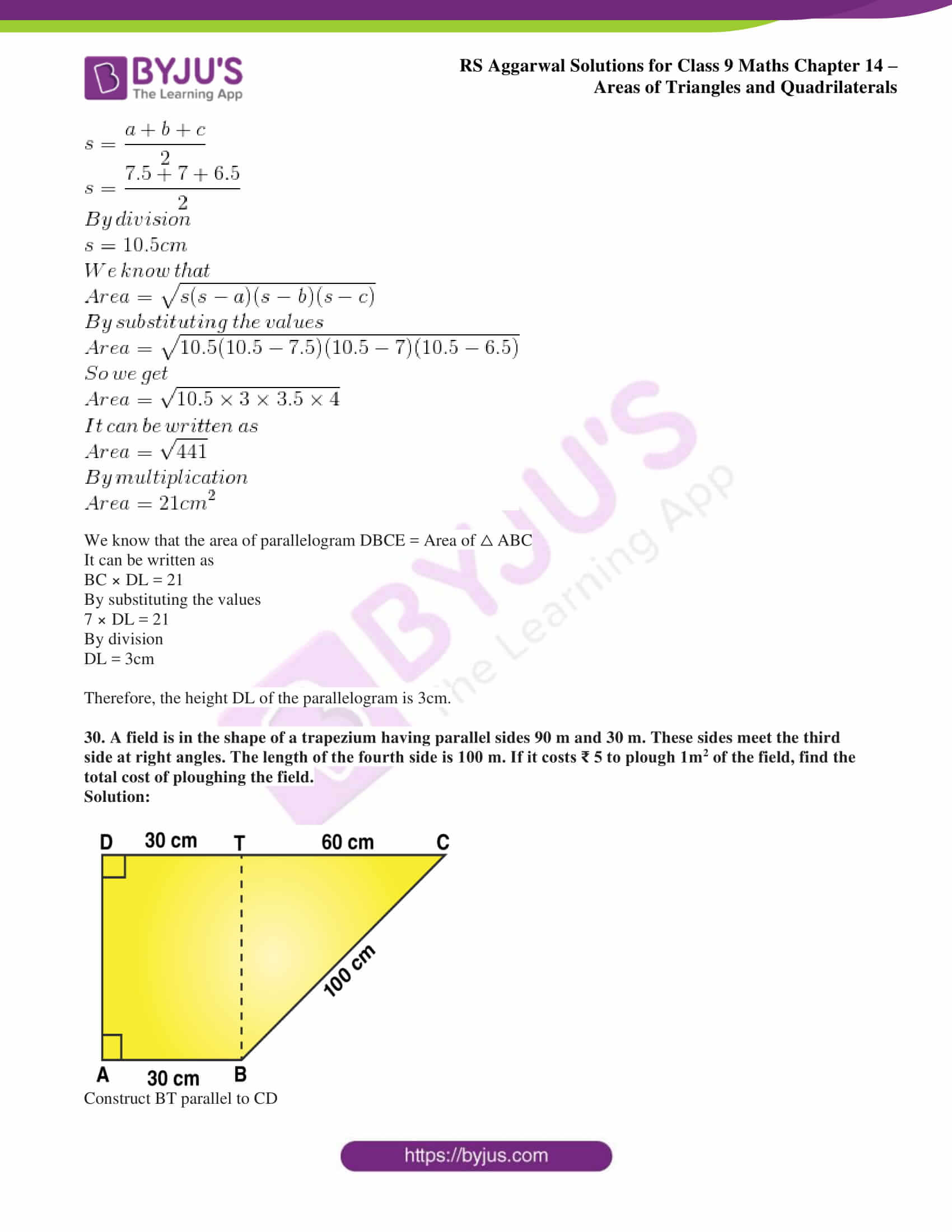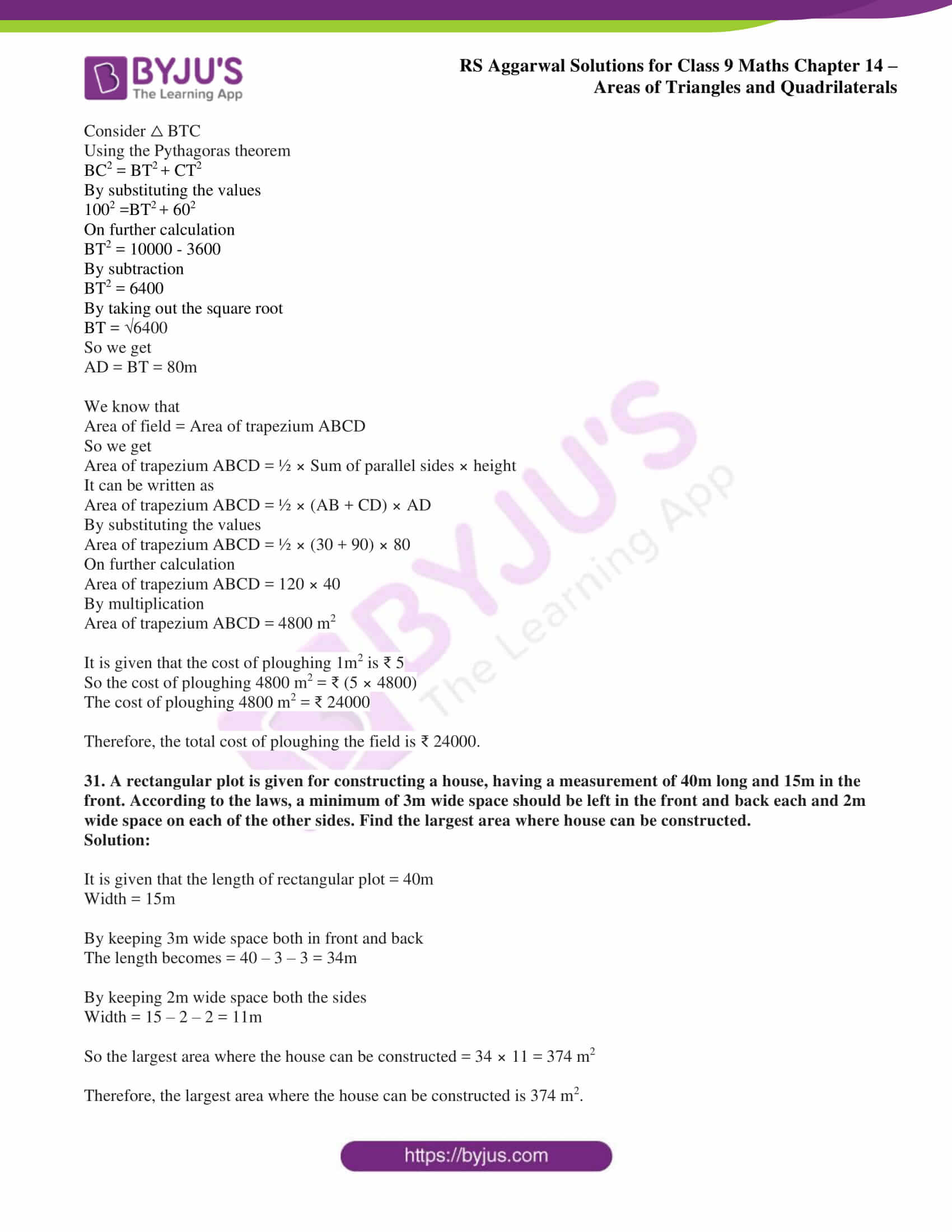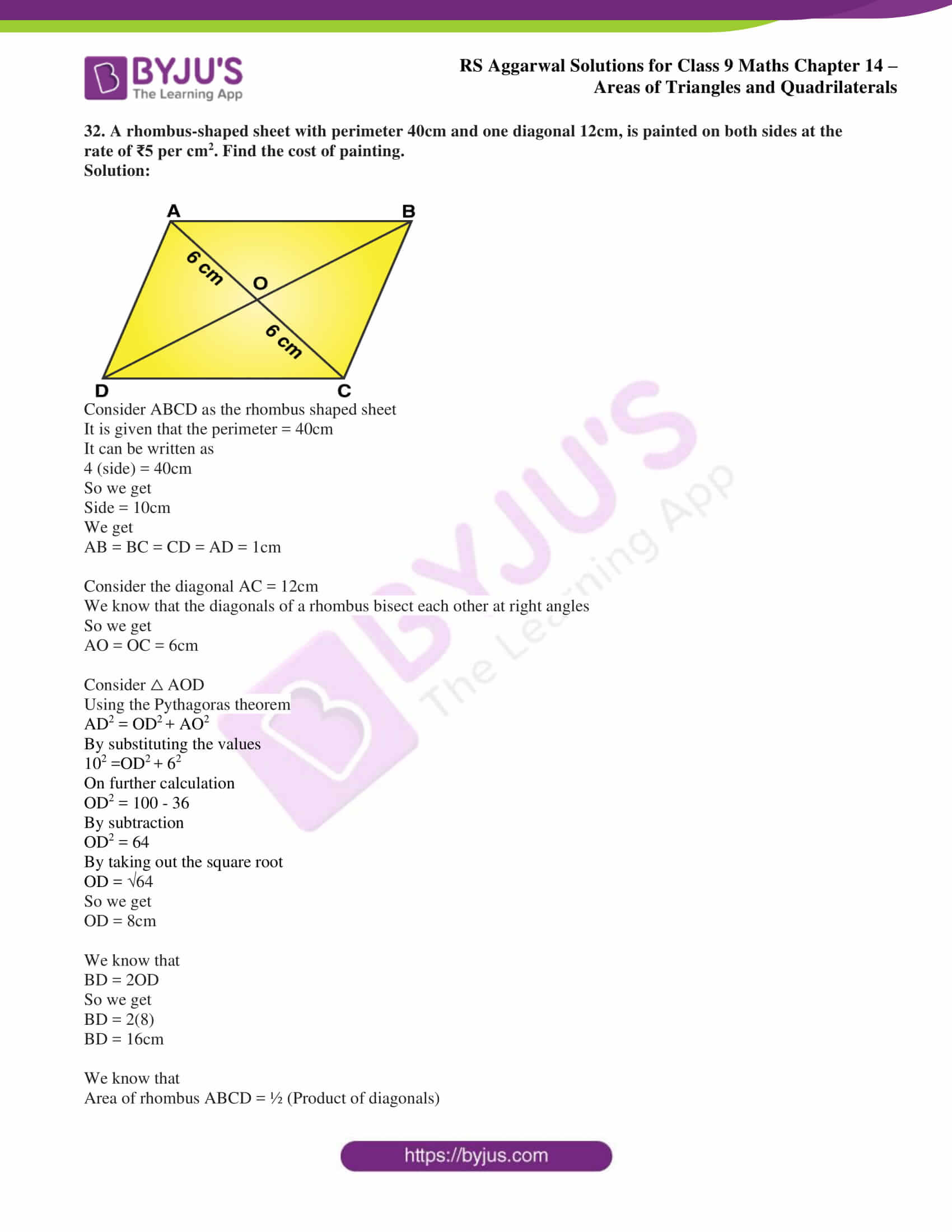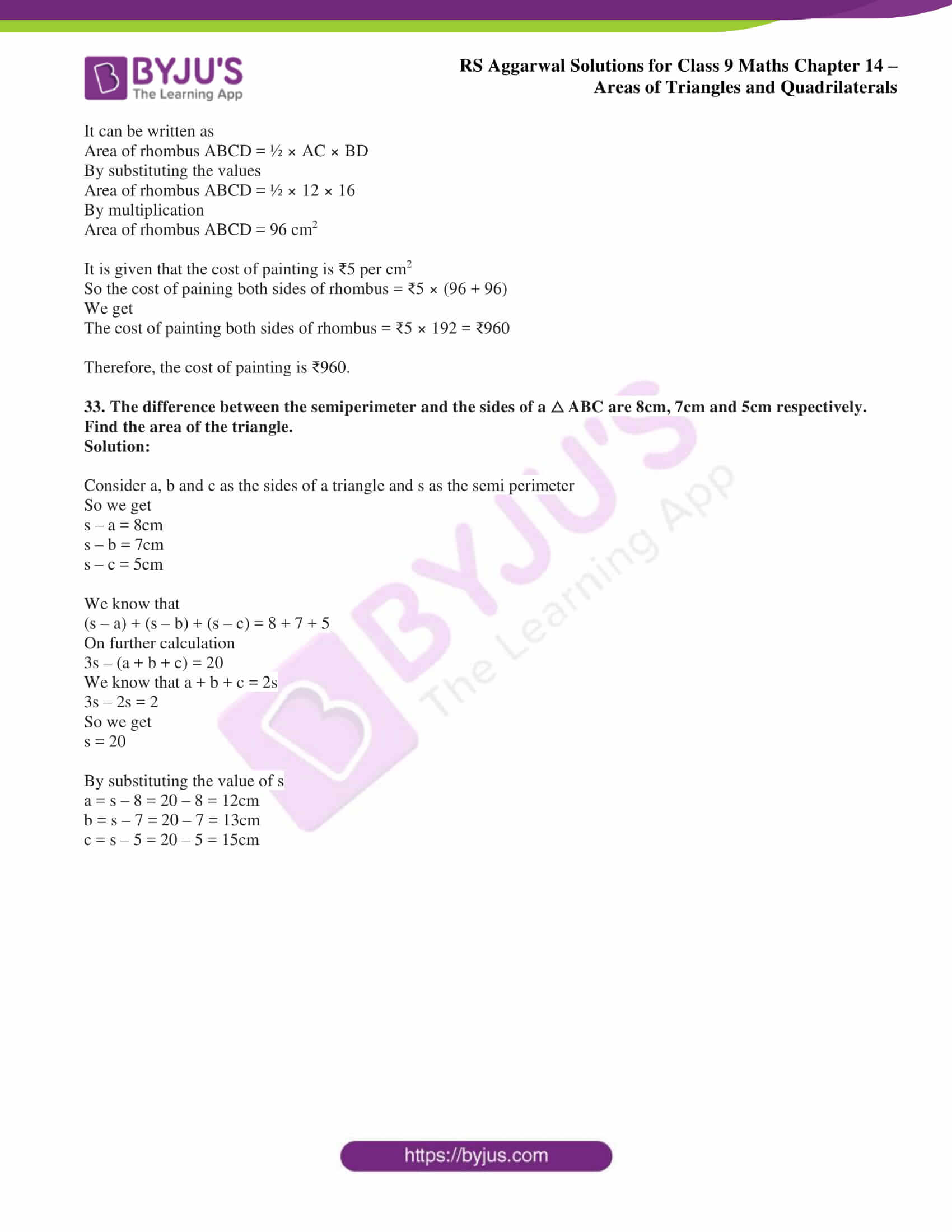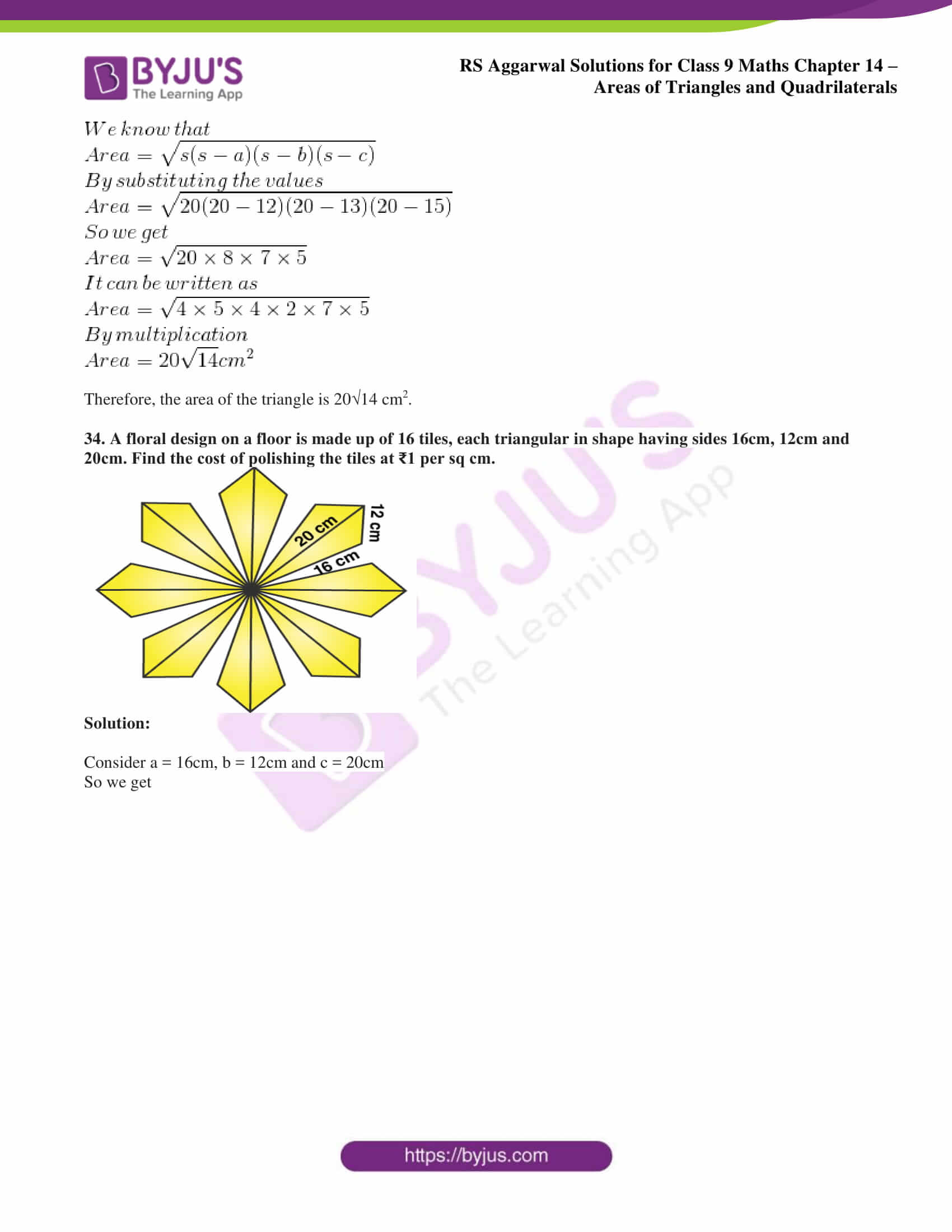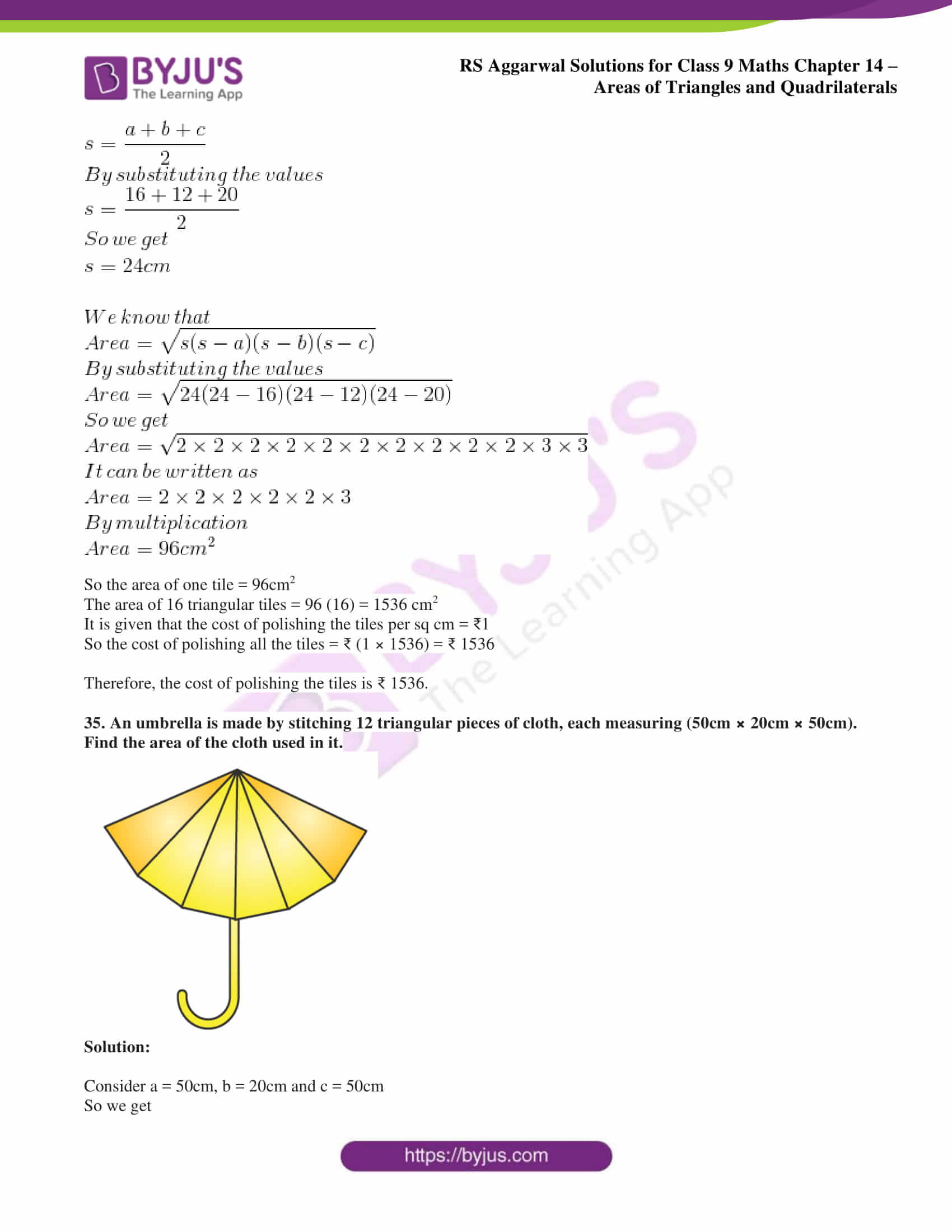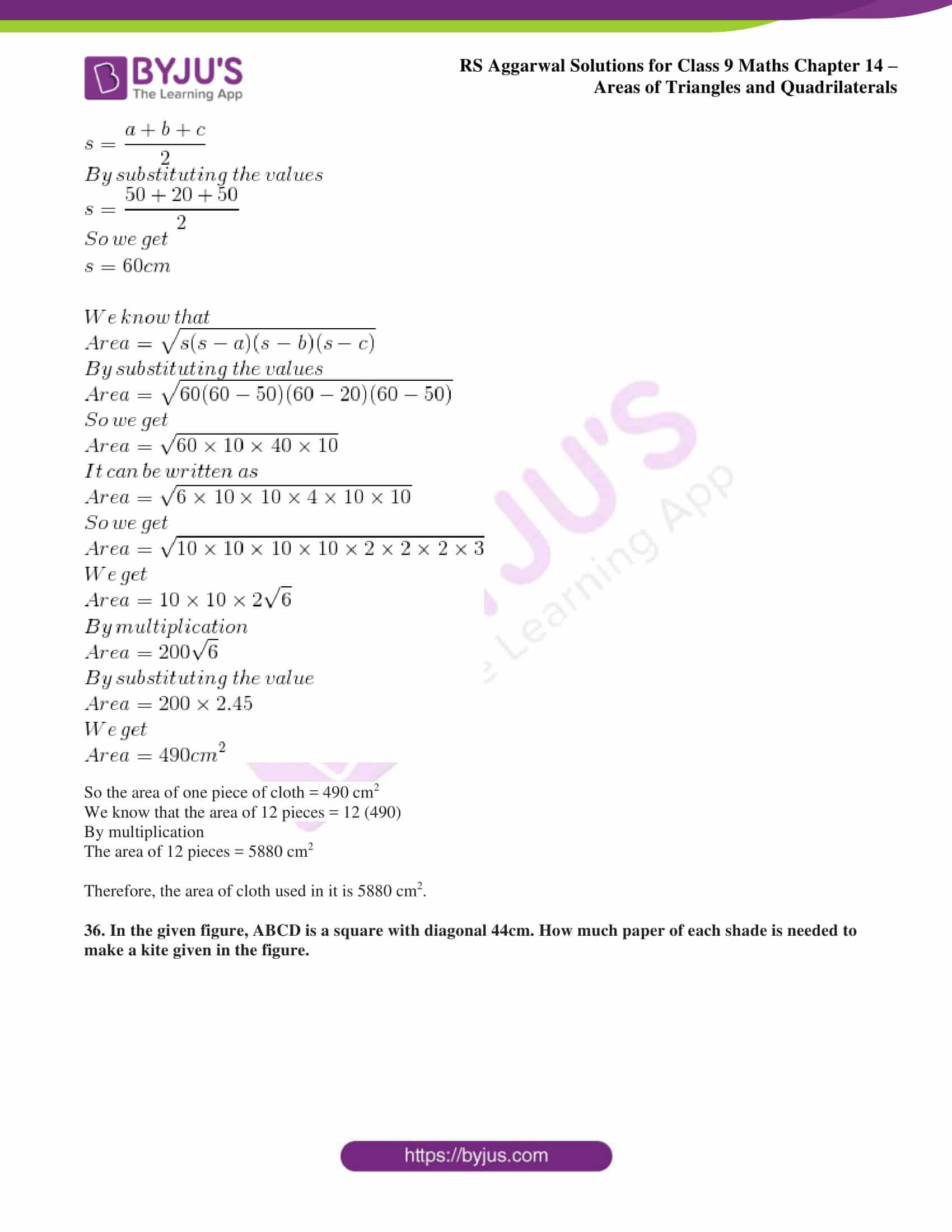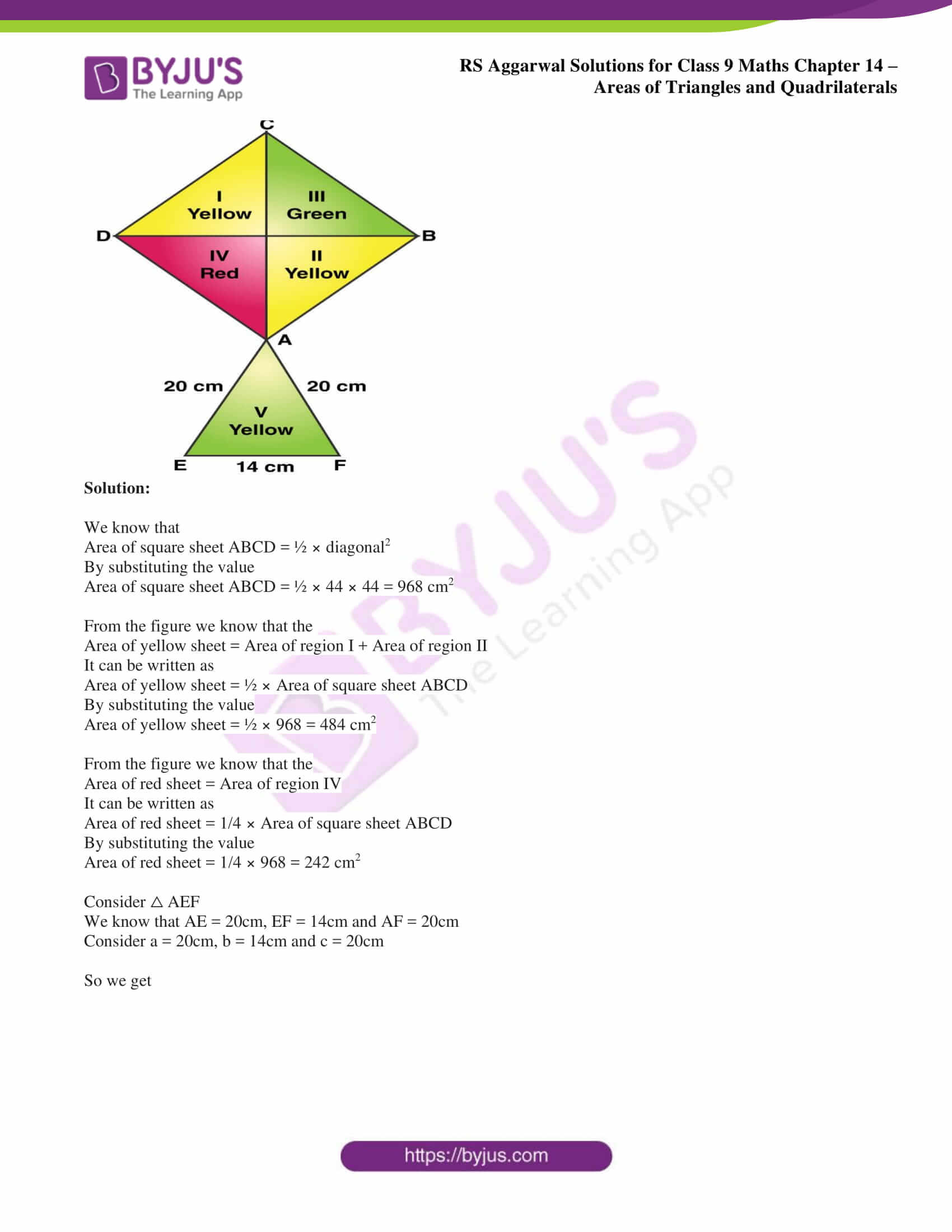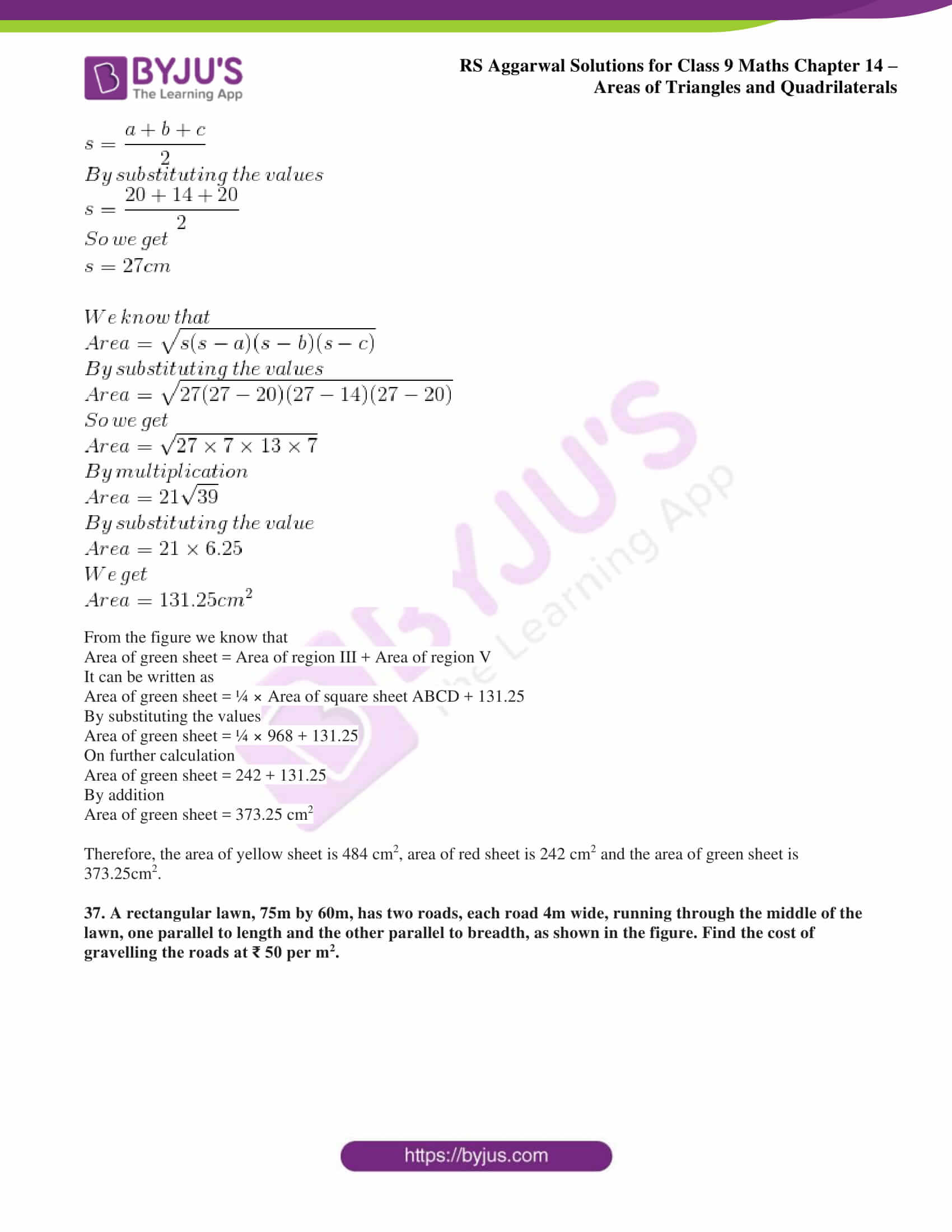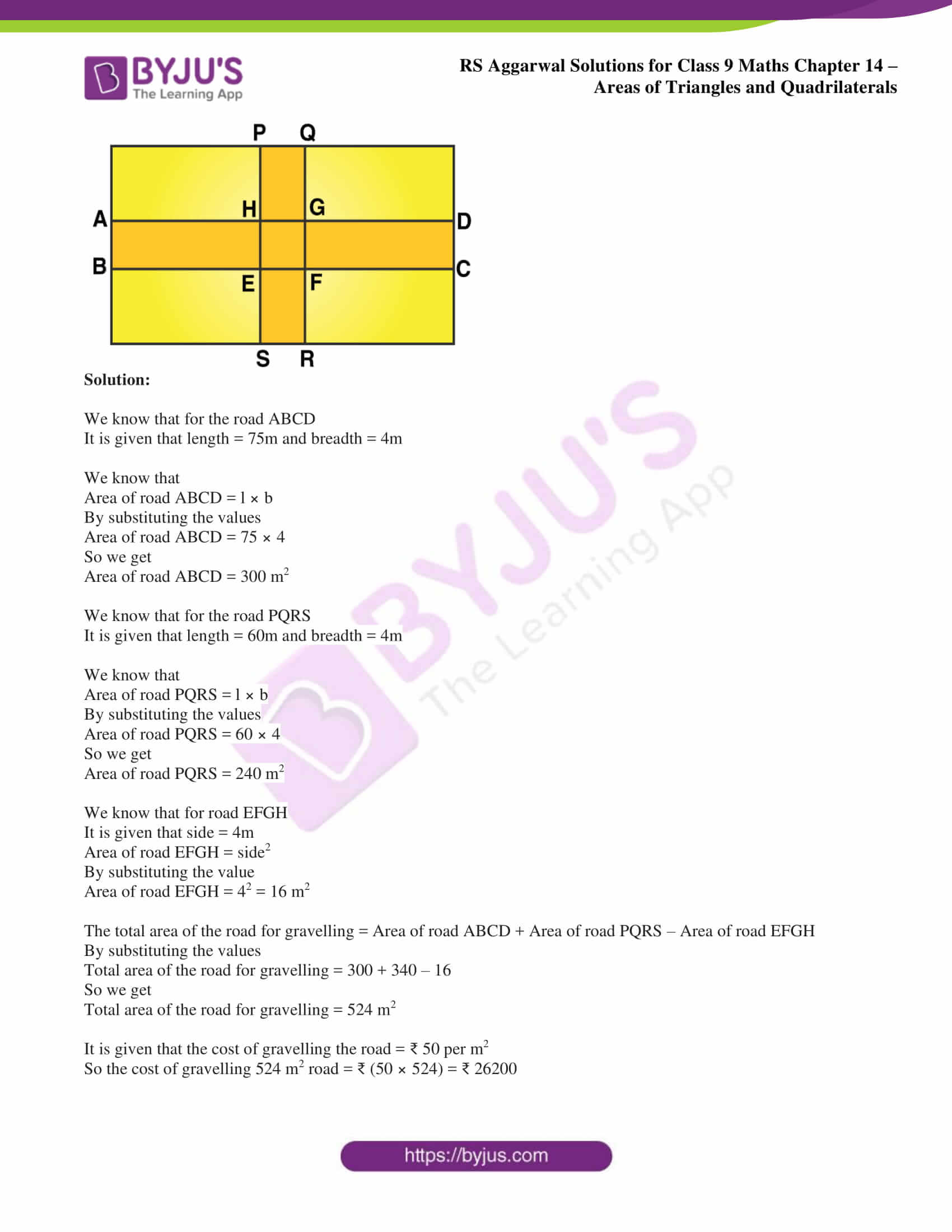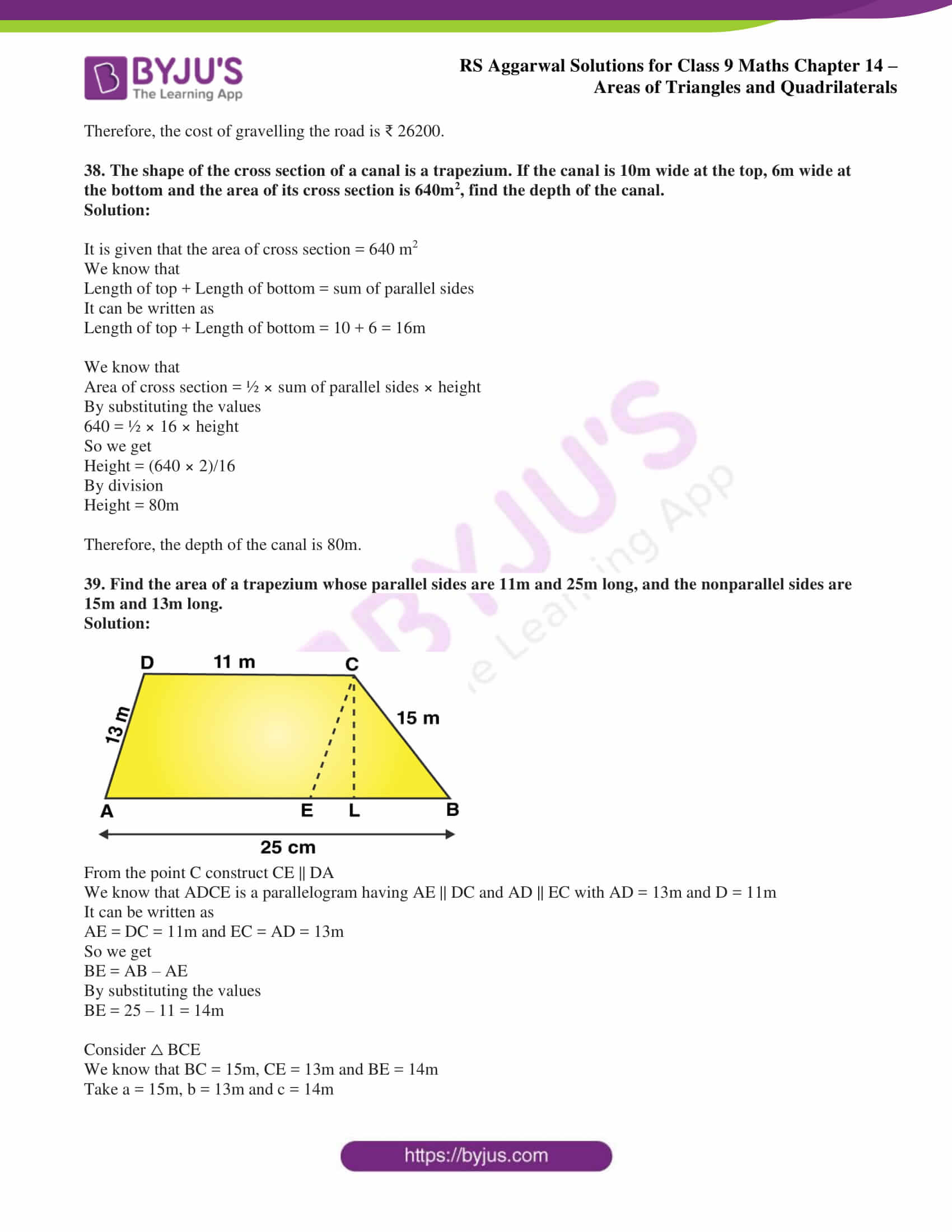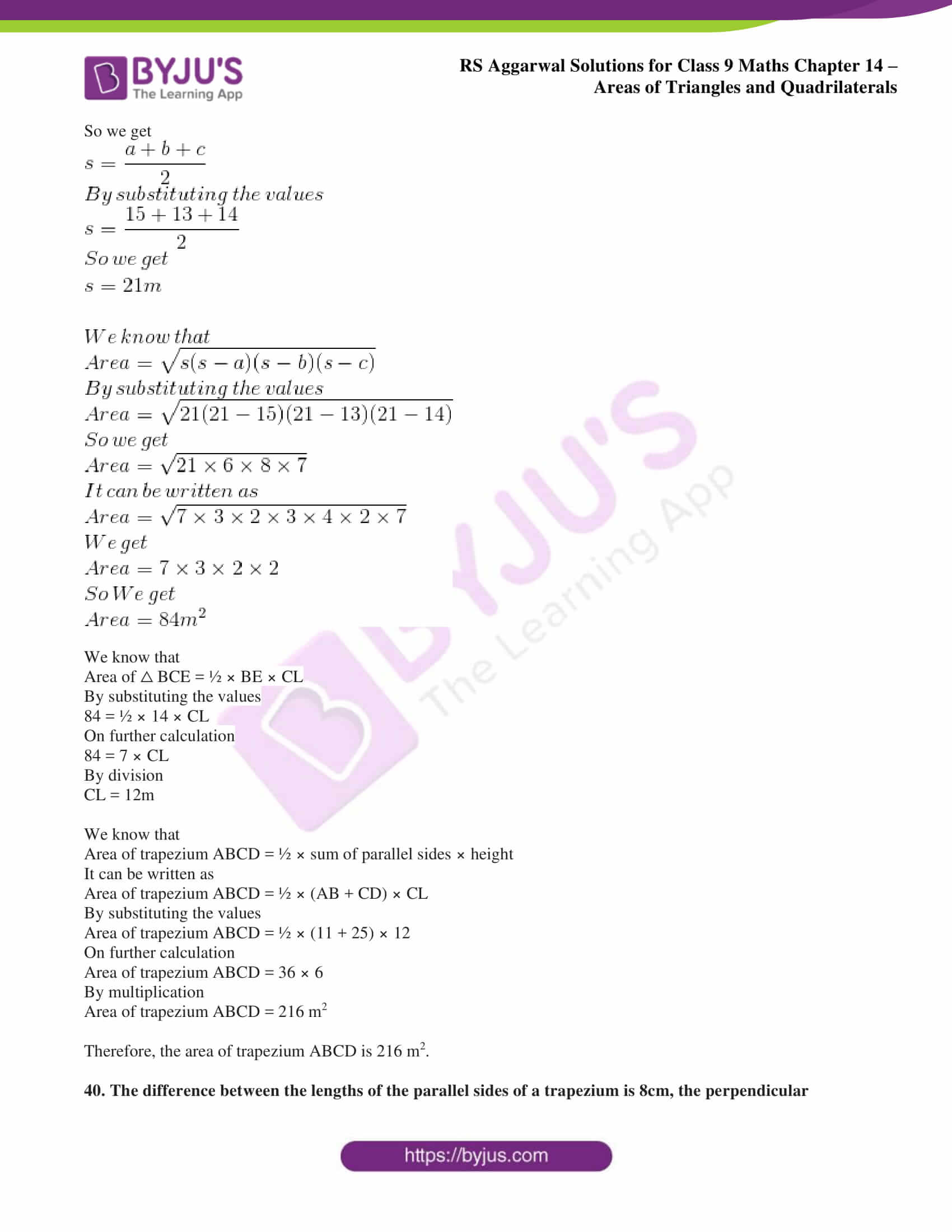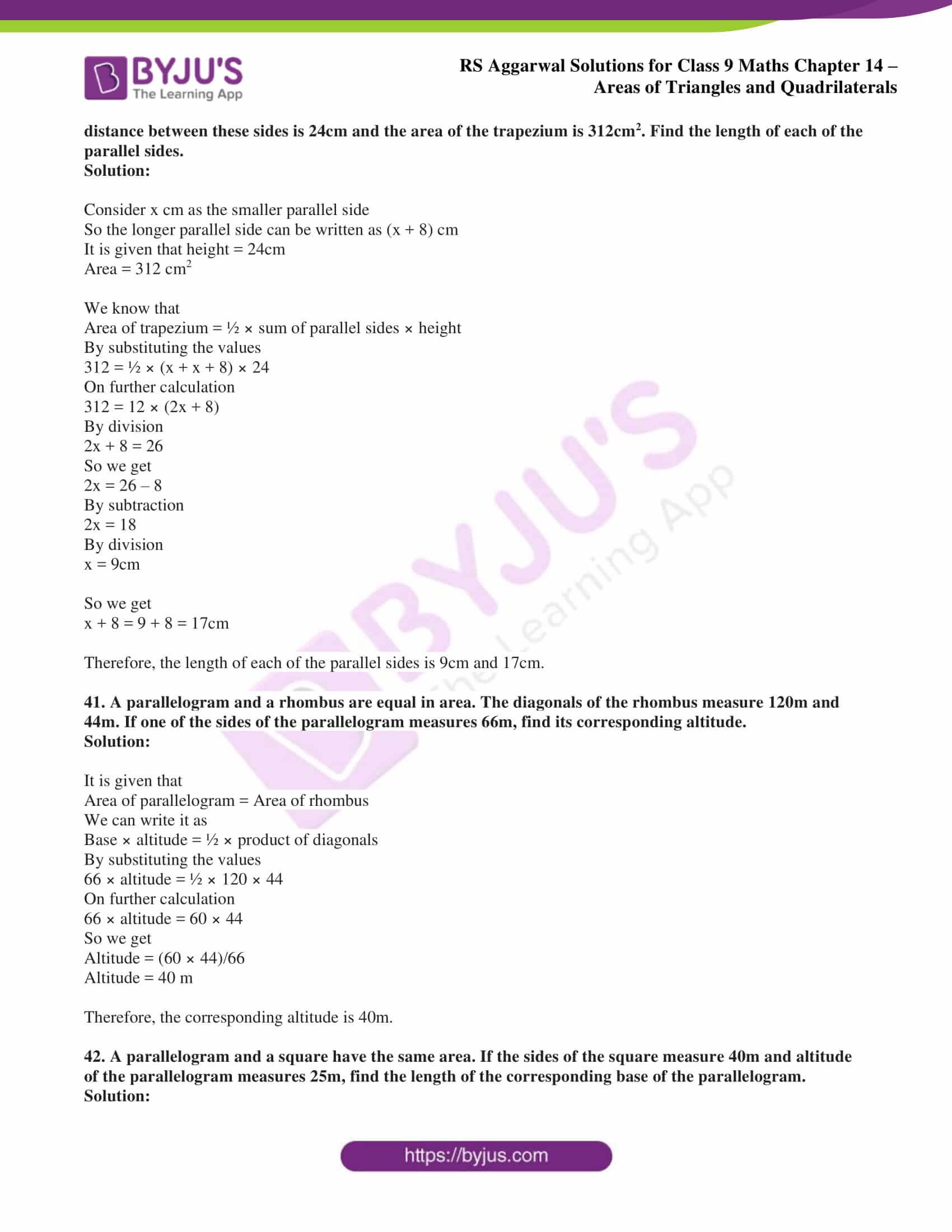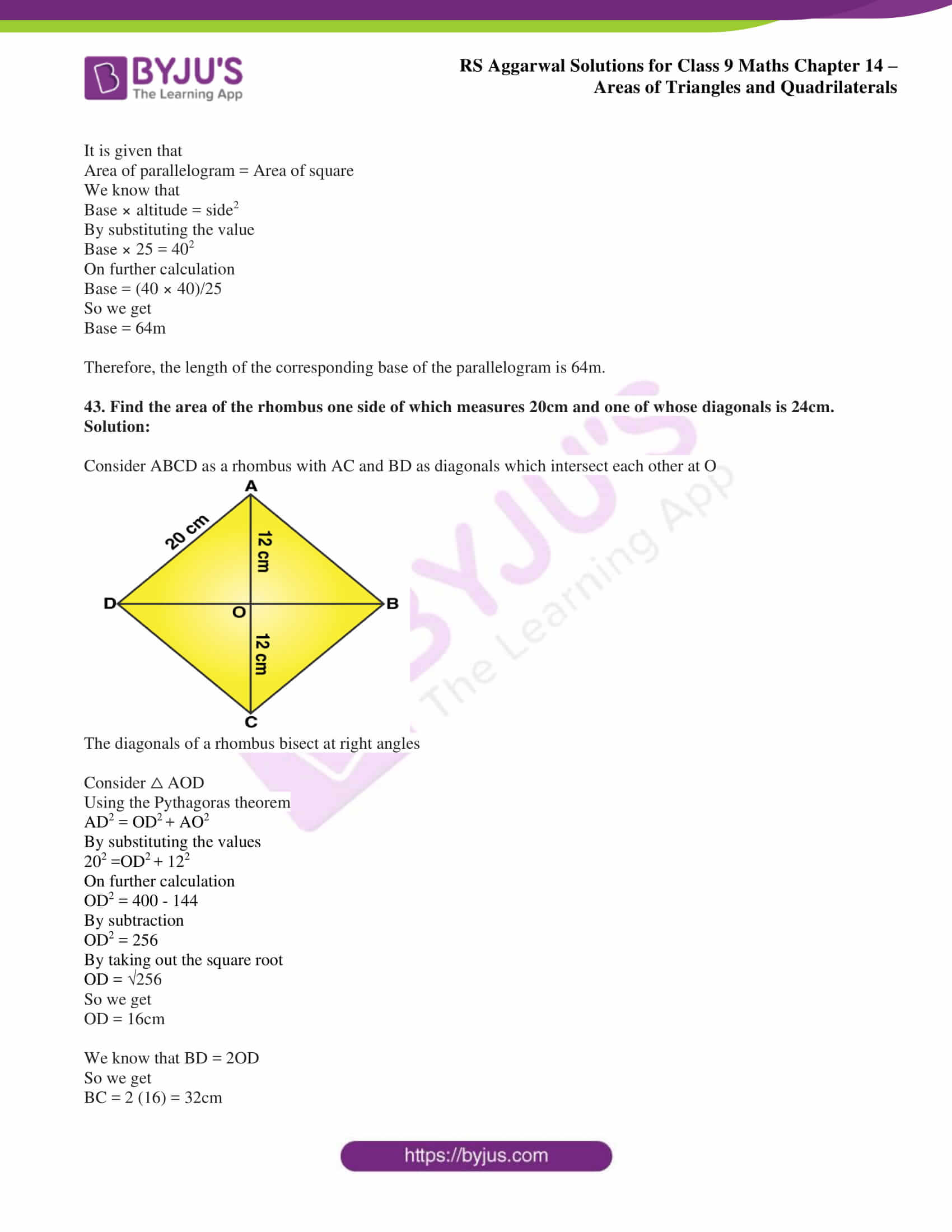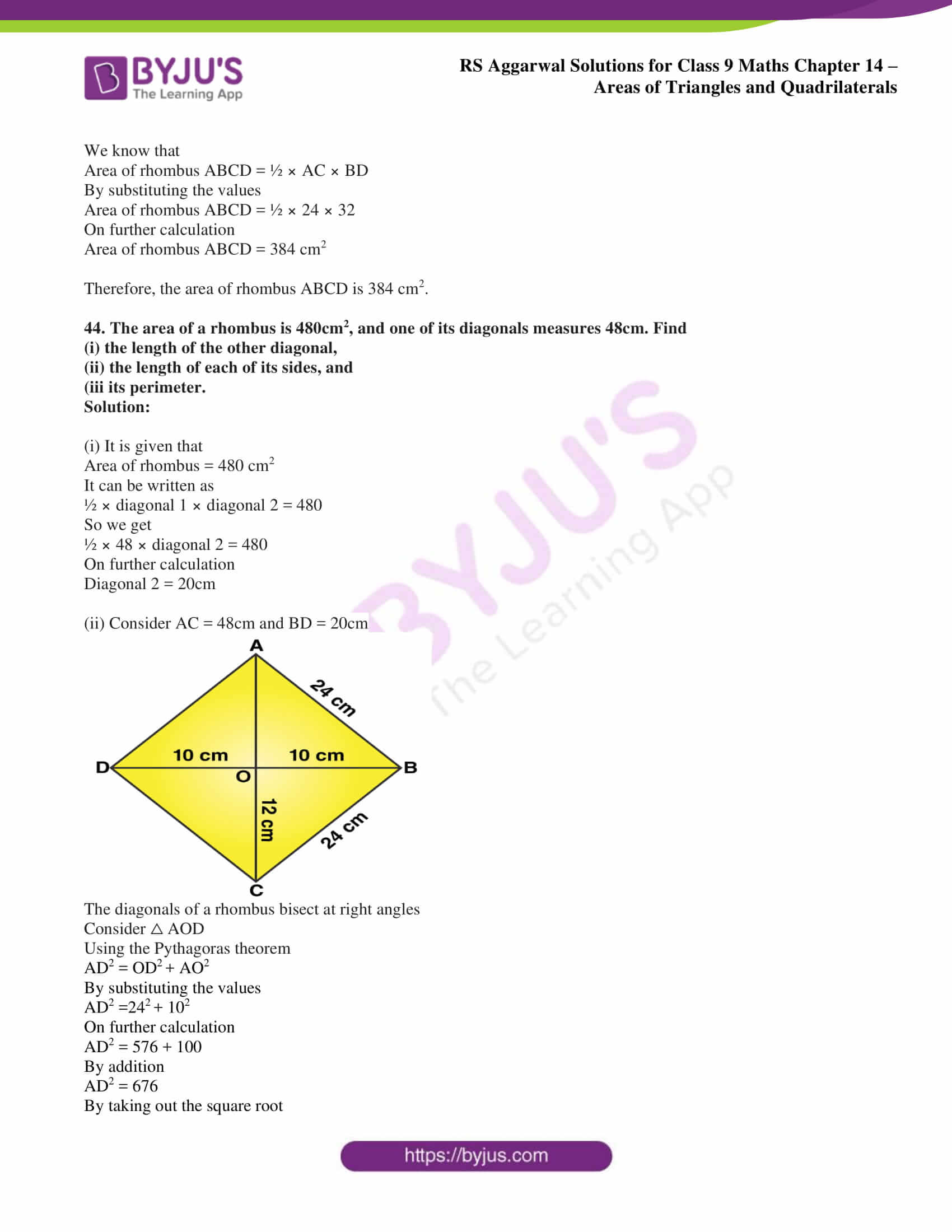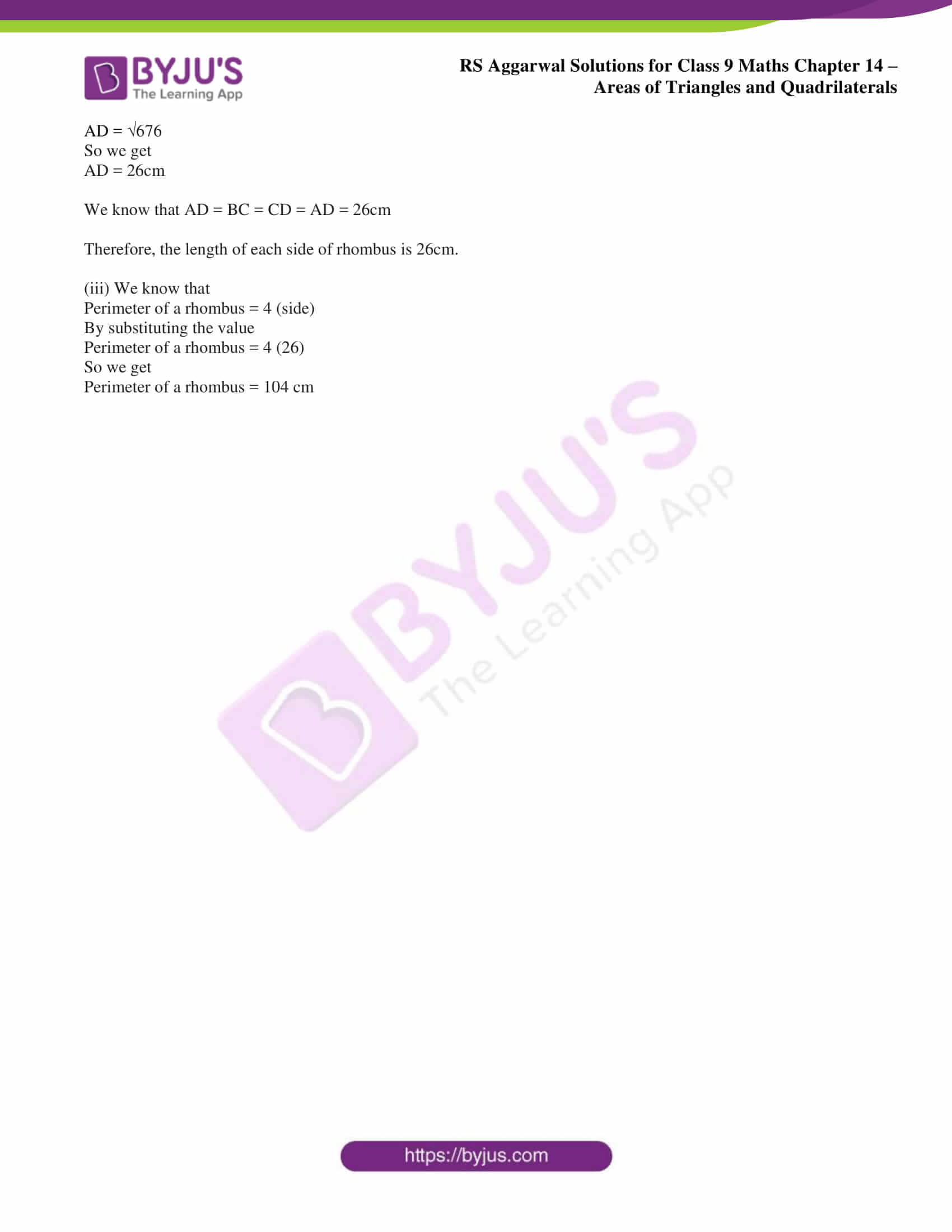## Access RS Aggarwal Solutions for Class 9 Chapter 14: Areas of Triangles and Quadrilaterals

Exercise 14 page: 533

1. Find the area of the triangle whose base measures 24cm and the corresponding height measures 14.5cm.

Solution:

It is given that b = 24 cm and h = 14.5 cm

We know that the

Area of triangle = ½ × b × h

By substituting the values

Area of triangle = ½ × 24 × 14.5

So we get

Area of triangle = 174 cm2

Therefore, the area of the triangle is 174 cm2.

2. The base of a triangular field is three times its altitude. If the cost of sowing the field at ₹ 58 per hectare is ₹ 783, find its base and height.

Solution:

Take x and the height and 3x as the base of the triangular field

We know that

Area of triangle = ½ × b × h

By substituting the values

Area of triangle = ½ × x × 3x

So we get

Area of triangle = 3/2 x2

1 hectare = 1000 sq. metre

It is given that

Cost of sowing the field per hectare = ₹ 58

Total rate of sowing the field = ₹ 783

So we can find the total cost by

Total cost = Area of the field × ₹ 58

By substituting the values

(3/2) x2 × (58/10000) = 783

By cross multiplication

x2 = (783/58) × (2/3) × 10000

On further calculation

x2 = 90000

By taking the square root

x = √90000

So we get

x = 300 m

Base = 3 × 300 = 900 m

Therefore, base = 900 m and height = 300 m.

3. Find the area of the triangle whose sides are 42cm, 34cm and 20cm in length. Hence, find the height corresponding to the longest side.

Solution:

Consider a = 42 cm, b = 34 cm and c = 20 cm

So we get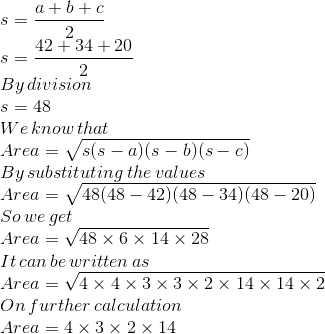So we get

Area = 336 cm2

It is given as

b = Longest side = 42 cm

Consider h as the height corresponding to the longest side

We know that

Area of the triangle = ½ × b × h

By substituting the values

½ × b × h = 336

On further calculation

42 × h = 336 × 2

So we get

h = (336 × 2)/42

By division

h = 16 cm

Therefore, Area = 336 cm2 and the height corresponding to the longest side is 16cm.

4. Calculate the area of the triangle whose sides are 18cm, 24cm and 30cm in length. Also, find the length of the altitude corresponding to the smallest side.

Solution:

It is given that a = 18cm, b = 24cm and c = 30cm

So we getSo we get

Area = 216 cm2

It is given as

b = Smallest side = 18 cm

Consider h as the height corresponding to the smallest side

We know that

Area of the triangle = ½ × b × h

By substituting the values

½ × b × h = 216

On further calculation

18 × h = 216 × 2

So we get

h = (216 × 2)/18

By division

h = 24 cm

Therefore, Area = 216 cm2 and the length of the altitude corresponding to the smallest side is 24 cm.

5. Find the area of a triangular field whose sides are 91m, 98m and 105m in length. Find the height corresponding to the longest sides.

Solution:

Consider a = 91m, b = 98m and c = 105m

So we get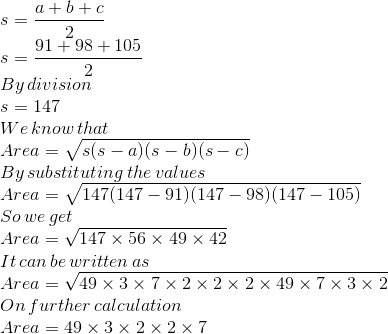So we get

Area = 4116 m2

It is given as

b = Longest side = 105 m

Consider h as the height corresponding to the longest side

We know that

Area of the triangle = ½ × b × h

By substituting the values

½ × b × h = 4116

On further calculation

105 × h = 4116 × 2

So we get

h = (4116 × 2)/105

By division

h = 78.4 m

Therefore, Area = 4116 m2 and the height corresponding to the longest sides is 78.4 m.

6. The sides of a triangle are in the ratio 5: 12: 13 and its perimeter is 150m. Find the area of the triangle.

Solution:

Consider the sides of the triangle as 5x, 12x and 13x

It is given that perimeter of the triangle = 150m

We can write it as

5x + 12x + 13x = 150m

On further calculation

30x = 150

So we get

x = 15/30

By division

x = 5m

By substituting the value of x we get

5x = 5 (5) = 25m

12x = 12 (5) = 60m

13x = 13 (5) = 65m

Consider a = 25m, b = 60m and c = 65m

So we get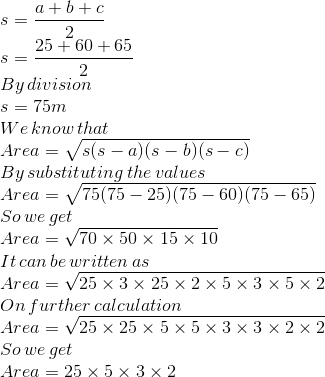By multiplication

Area = 750 sq. m

Therefore, the area of the triangle is 750 sq. m.

7. The perimeter of a triangular field is 540m and its sides are in the ratio 25: 17: 12. Find the area of the field. Also, find the cost of ploughing the field at ₹ 5 per m2.

Solution:

Consider a, b, c as the sides of a triangle in the ratio 25: 17: 12

It can be written as

a: b: c = 25: 17: 12

So we get

a = 25, b = 17 and c = 12

It is given that

Perimeter = 540 m

So we get

25x + 17x + 12x = 540

On further calculation

54x = 540

By division

x = 10

By substituting the value of x

a = 25x = 25(10) = 250m

b = 17x = 17(10) = 170m

c = 12x = 12(10) = 120m

We know that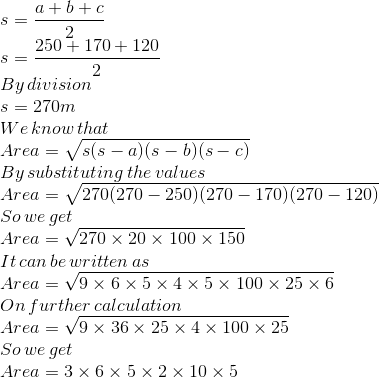Area = 9000m2

It is given that the cost of ploughing the field is ₹ 5 per m2

So the cost of ploughing 9000m2 = 5 × 9000

By multiplication

The cost of ploughing 9000m2 = ₹ 45000

Therefore, the area of the field is 9000m2 and the cost of ploughing the field is ₹ 45000.

8. Two sides of a triangular field are 85m and 154m in length and its perimeter is 324m. Find

(i) the area of the field and

(ii) the length of the perpendicular from the opposite vertex on the side measuring 154m.

Solution:

It is given that two sides of a triangular field are 85m and 154m

Consider the third side as x m

It is given that the perimeter = 324m

We can write it as

85 + 154 + x = 324

On further calculation we get

x = 324 – 85 – 154

By subtraction

x = 85m

Consider a = 85m, b = 154m and c = 85m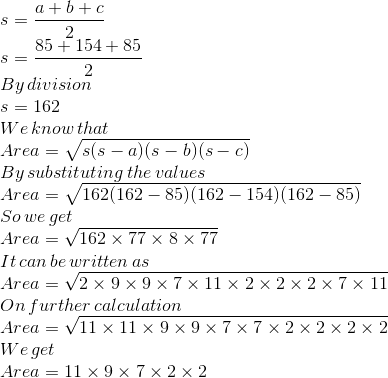By multiplication

Area = 2772 m2

We know that

Area of the triangle = ½ × b × h

By substituting the values

½ × 154 × h = 2772

On further calculation

77 × h = 2772

So we get

h = 2772/77

By division

h = 36 m

Therefore, area of the field is 2772 m2 and the length of the perpendicular from the opposite vertex on the side measuring 154m is 36m.

9. Find the area of an isosceles triangle each of whose equal sides measures 13cm and whose base measures 20cm.

Solution:

Consider a = 13cm, b = 13cm and c = 20cm

So we get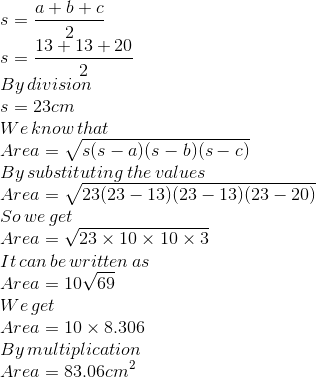Therefore, area of an isosceles triangle is 83.06cm2.

10. The base of an isosceles triangle measures 80cm and its area is 360cm2. Find the perimeter of the triangle.

Solution:

Consider △ ABC as an isosceles triangle with AL perpendicular to BC

It is given that BC = 80cm and area = 360 cm2

We know that area of a triangle = ½ × b × h

By substituting the values

½ × BC × AL = 360 cm2

So we get

½ × 80 × AL = 360 cm2

On further calculation

40 × h = 360

By division

h = 9cm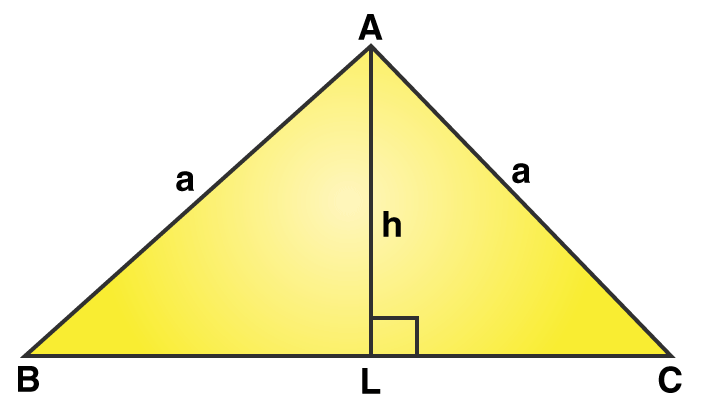We know that BL = ½ × BC

By substituting the values

BL = ½ × 80

By division

BL = 40cm and AL = 9cm

Using the Pythagoras theorem

We know that

a = √ (BL2 + AL2)

By substituting the values

a = √ (402 + 92)

So we get

a = √ (1600 + 81)

a = √ 1681

So we get

a = 41 cm

So the perimeter of the isosceles triangle = 41 + 41 + 8

We get

Perimeter of the isosceles triangle = 162 cm

Therefore, the perimeter of the triangle is 162 cm.

11. The perimeter of an isosceles triangle is 32cm. The ratio of the equal side to its base is 3:2. Find the area of the triangle.

Solution:

We know that the ratio of the equal side to its base is 3:2

For an isosceles triangle the ratio of sides can be written as 3: 3: 2

So we get

a: b: c = 3: 3: 2

Consider a = 3x, b = 3x and c = 2x

We know that the perimeter = 32cm

It can be written as

3x + 3x + 2x = 32

On further calculation

8x = 32

By division

x = 4

By substituting the value of x

a = 3x = 3(4) = 12cm

b = 3x = 3(4) = 12cm

c = 2x = 2(4) = 8cm

So we get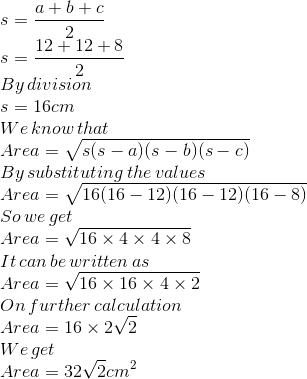Therefore, area of the triangle is 32√2 cm2.

12. The perimeter of a triangle is 50cm. One side of the triangle is 4 cm longer than the smallest side and the third side is 6cm less than twice the smallest side. Find the area of the triangle.

Solution:

Consider the three sides of a triangle as a, b and c with c as the smallest side

So we get

a = c + 4

In the same way

b = 2c – 6

It is given that perimeter of a triangle = 50cm

We know that

a + b + c = 50

By substituting the values

(c + 4) + (2c – 6) + c = 50

On further calculation

4c – 2 = 50

4c = 50 + 2

4c = 52

By division

c = 13

By substituting the value of c

a = c + 4 = 13 + 4

a = 17cm

b = 2c – 6 = 2(13) – 6

b = 20cm

So we get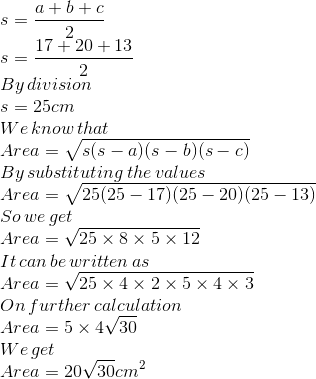Therefore, area of the triangle is 20√30 cm2.

13. The triangular side walls of a flyover have been used for advertisements. The sides of the walls are 13m, 14m, 15m. The advertisements yield an earning of ₹ 2000 per m2 a year. A company hired one of its walls for 6 months. How much rent did it pay?

Solution:

Consider three sides of the wall as a = 13m, b = 14m and c = 15m

So we get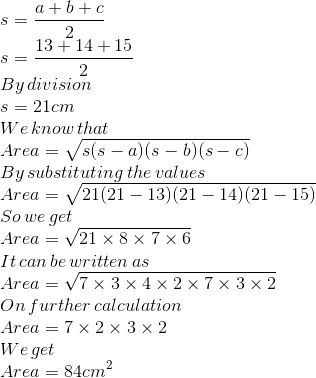It is given that the advertisements yield per year = ₹ 2000 per m2

So the rent for 6 months = ₹ 1000 per m2

Total rent paid for advertisements for 6 months = ₹ (1000 × 84) = ₹ 84000

Therefore, the total rent paid in 6 months is ₹ 84000.

14. The perimeter of an isosceles triangle is 42cm and its base is 1 ½ times each of the equal sides. Find

(i) the length of each side of the triangle,

(ii) the area of the triangle, and

(iii) the height of the triangle. (Given, √7 = 2.64.)

Solution:

We know that the lateral sides of an isosceles triangle are of equal length

Consider the length of lateral side as x cm

It is given that base = 3/2 × x cm

(i) It is given that the perimeter = 42cm

We can write it as

x + x + 3/2 x = 42cm

By multiplying the entire equation by 2

2x + 2x + 3x = 84cm

On further calculation

7x = 84

By division

x = 12 cm

So the length of lateral side = 12 cm

Base = 3/2 x = 3/2 (12)

So we get the base = 18cm

Therefore, the length of each side of the triangle is 12cm, 12 cm and 18cm.

(ii) Consider a = 12cm, b = 12cm and c = 18cm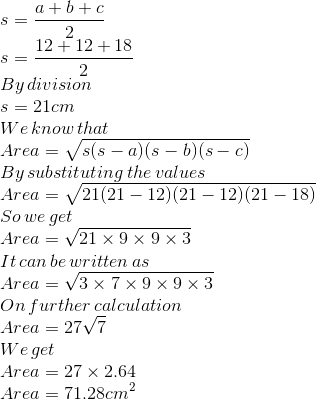Therefore, the area of the triangle is 71.28cm2.

(iii) We know that

Area of a triangle = ½ × b × h

By substituting the values

71.28 = ½ × 18 × h

On further calculation

71.28 = 9 × h

By division

h = 7.92cm

Therefore, the height of the triangle is 7.92cm.

15. If the area of an equilateral triangle is 36√3 cm2, find its perimeter.

Solution:

Consider a as the length of a side of an equilateral triangle

We know that

Area of an equilateral triangle = (√3 × a2)/4 sq units

It is given that the area = 36√3 cm2

We can write it as

(√3 × a2)/4 = 36√3

On further calculation

a2 = (36√3 × 4)/ √3

So we get

a2 = 36 × 4

By multiplication

a2 = 144

By taking the square root

a = √144

So we get

a = 12 cm

By substituting the value of a

Perimeter = 3a = 3 (12) = 36cm

Therefore, the perimeter of the equilateral triangle is 36cm.

16. If the area of an equilateral triangle is 81√3 cm2, find its height.

Solution:

Consider a as the length of a side of an equilateral triangle

We know that

Area of an equilateral triangle = (√3 × a2)/4 sq units

It is given that the area = 81√3 cm2

We can write it as

(√3 × a2)/4 = 81√3

On further calculation

a2 = (81√3 × 4)/ √3

So we get

a2 = 324

By taking the square root

a = √324

So we get

a = 18 cm

We know that the height of an equilateral triangle = √3/2 a

By substituting the value of a

Height of an equilateral triangle = √3/2 (18) = 9√3 cm

Therefore, the height of an equilateral triangle is 9√3 cm.

17. Each side of an equilateral triangle measures 8cm. Find

(i) the area of the triangle, correct to 2 places of decimal and

(ii) the height of the triangle, correct to 2 places of decimal. (Take √3 = 1.732)

Solution:

(i) Consider a as the side of equilateral triangle i.e. a = 8cm

Area of an equilateral triangle = (√3 × a2)/4 sq units

So we get

Area of the equilateral triangle = (√3 × 82)/4

On further calculation

Area of the equilateral triangle = (√3 × 64)/4

So we get

Area of the equilateral triangle = √3 × 16

By substituting the value of √3

Area of the equilateral triangle = 1.732 × 16 = 27.712

By correcting to two places of decimal

Area of the equilateral triangle = 27.71 cm2

(ii) We know that

Height of an equilateral triangle = √3/2 a

By substituting the value

Height of an equilateral triangle = √3/2 × 8

So we get

Height of an equilateral triangle = √3 × 4

By substituting the value of √3

Height of an equilateral triangle = 1.732 × 4 = 6.928

By correcting to two places of decimal

Height of an equilateral triangle = 6.93 cm

18. The height of an equilateral triangle measures 9cm. Find its area, correct to 2 places of decimal. (Take √3 = 1.732)

Solution:

Consider a as the side of an equilateral triangle

We know that

Height of an equilateral triangle = √3/2 a

It is given that height = 9cm

So we get

√3/2 a = 9

On further calculation

a = (9 × 2)/ √3

Multiplying both numerator and denominator by √3

We get

a = (9 × 2 × √3) / (√3 × √3)

So we get

Base = a = 6√3cm

We know that the area of an equilateral triangle = ½ × b × h

By substituting the values

Area of the equilateral triangle = ½ × 6√3 × 9

On further calculation

Area of the equilateral triangle = 27√3 cm2

By substituting the value of √3

Area of the equilateral triangle = 27 × 1.732 = 46.764

By correcting to 2 places of decimal

Area of the equilateral triangle = 46.76 cm2

Therefore, the area of an equilateral triangle = 46.76 cm2.

19. The base of a right-angled triangle measures 48 cm and its hypotenuse measures 50 cm. Find the area of the triangle.

Solution:

It is given that

Base = BC = 48 cm

Hypotenuse = AC = 50 cm

Consider AB = x cm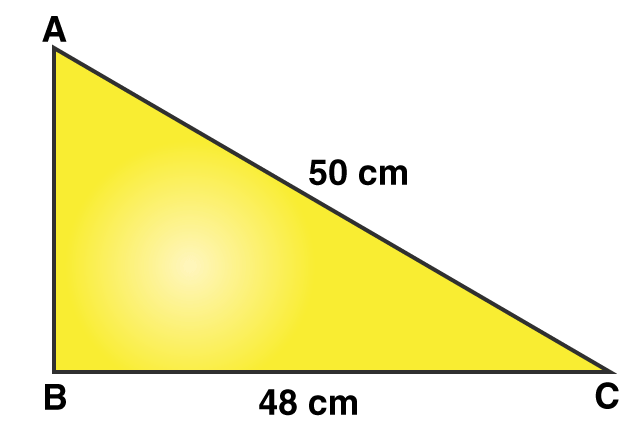Using the Pythagoras theorem

AC2 = AB2 + BC2

By substituting the values

502 = x2 + 482

On further calculation

x2 = 502 – 482

So we get

x2 = 2500 – 2304

By subtraction

x2 = 196

By taking the square root

x = √196

So we get

x = 14cm

We know that the area of a right angled triangle = ½ × b × h

By substituting the values

Area of a right angled triangle = ½ × 48 × 14

On further calculation

Area of a right angled triangle = 24 × 14

By multiplication

Area of the triangle = 336 cm2

Therefore, the area of the triangle = 336 cm2.

20. Find the area of the shaded region in the figure given below.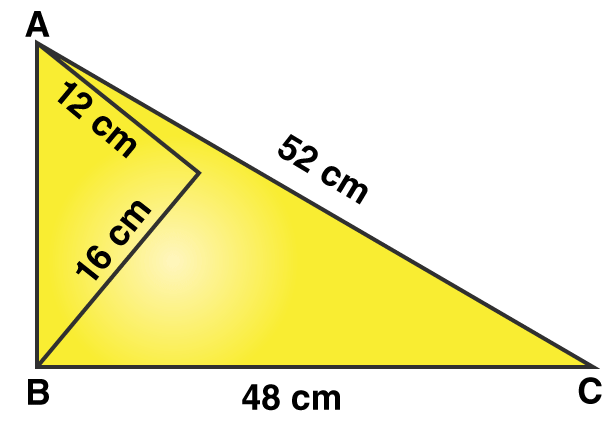Solution:

Consider △ ABD

Using Pythagoras theorem

By substituting the values

AB2 = 122 + 162

On further calculation

AB2 = 144 + 256

AB2 = 400

By taking out the square root

AB = √400

So we get

AB = 20cm

We know that the area of △ ABD = ½ × b × h

It can be written as

Area of △ ABD = ½ × AD × BD

By substituting the values

Area of △ ABD = ½ × 12 × 16

On further calculation

Area of △ ABD = 96 cm2

Consider △ ABC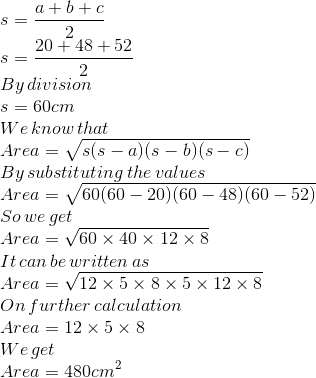So the area of the shaded region = Area of △ ABC – Area of △ ABD

By substituting the values

Area of the shaded region = 480 – 96 = 384 cm2

Therefore, the area of the shaded region is 384 cm2.

21. The sides of a quadrilateral ABCD taken in order are 6cm, 8cm, 12 cm and 14cm respectively and the angle between the first two sides is a right angle. Find its area. (Given, √6 = 2.45.)

Solution: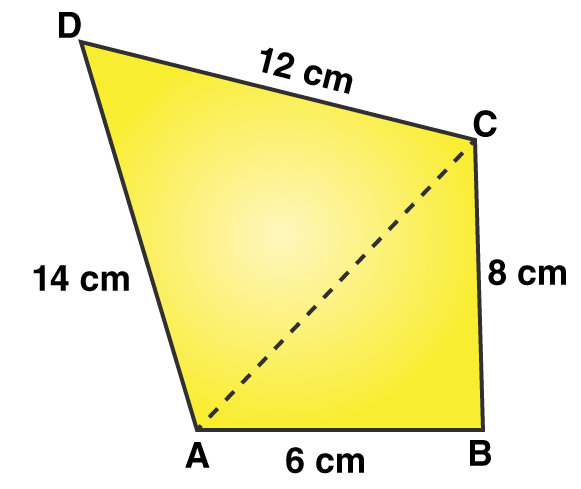It is given that AB = 6cm, BC = 8cm, CD = 12cm and AD = 14cm

Consider △ ABC

Using the Pythagoras theorem

AC2 = AB2 + BC2

By substituting the values

AC2 = 62 + 82

On further calculation

AC2 = 36 + 64

AC2 = 100

By taking out the square root

AC = √100

So we get

AC = 10cm

We know that the area of △ ABC = ½ × b × h

It can be written as

Area of △ ABC = ½ × AB × BC

By substituting the values

Area of △ ABC = ½ × 6 × 8

On further calculation

Area of △ ABC = 24 cm2

We know that AC = 10cm, CD = 12cm and AD = 14cm

It can be written as a = 10cm, b = 12cm and c = 14cm

So we get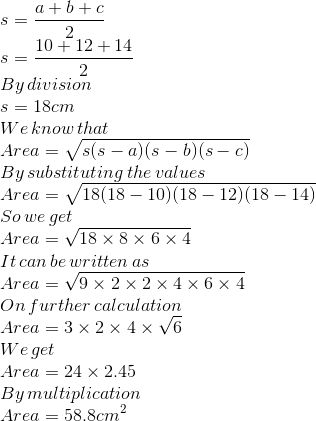So the area of quadrilateral ABCD = Area of △ ABC + Area of △ ACD

By substituting the values

Area of quadrilateral ABCD = 24 + 58.8

Area of quadrilateral ABCD = 82.8 cm2

Therefore, the area of quadrilateral ABCD is 82.8 cm2.

22. Find the perimeter and area of a quadrilateral ABCD in which BC = 12 cm, CD = 9 cm, BD = 15cm, DA = 17 cm and ∠ ABD = 90o.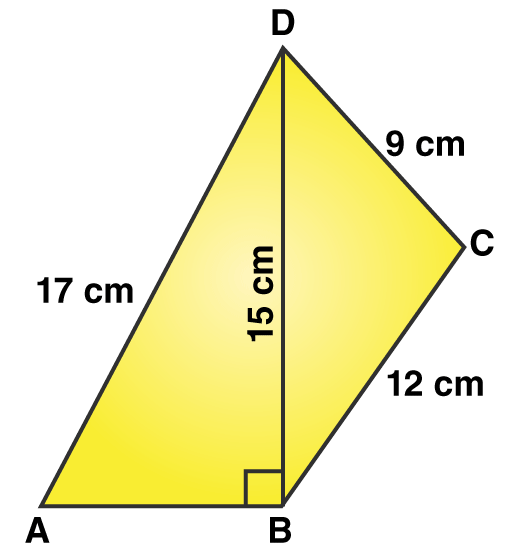Solution:

Consider △ ABD

Using the Pythagoras theorem

By substituting the values

172 =AB2 + 152

On further calculation

AB2 = 289 – 225

By subtraction

AB2 = 64

By taking out the square root

AB = √64

So we get

AB = 8cm

We know that

Perimeter of quadrilateral ABCD = AB + BC + CD + AD

By substituting the values

Perimeter = 8 + 12 + 9 + 17

Perimeter = 46cm

We know that area of △ ABD = ½ × b × h

It can be written as

Area of △ ABD = ½ × AB × BD

By substituting the values

Area of △ ABD = ½ × 8 × 15

On further calculation

Area of △ ABD = 60 cm2

Consider △ BCD

We know that BC = 12cm, CD = 9cm and BD = 15cm

It can be written as a = 12cm, b = 9cm and c = 15cm

So we get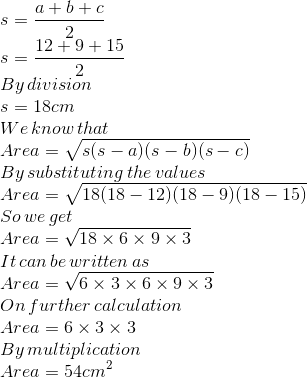So the area of quadrilateral ABCD = Area of △ ABD + Area of △ BCD

By substituting the values

Area of quadrilateral ABCD = 60 + 54 = 114 cm2

Therefore, the perimeter is 46cm and the area is 114 cm2.

23. Find the perimeter and area of the quadrilateral ABCD in which AB = 21 cm, ∠ BAC = 90o, AC = 20cm, CD = 42 cm and AD = 34 cm.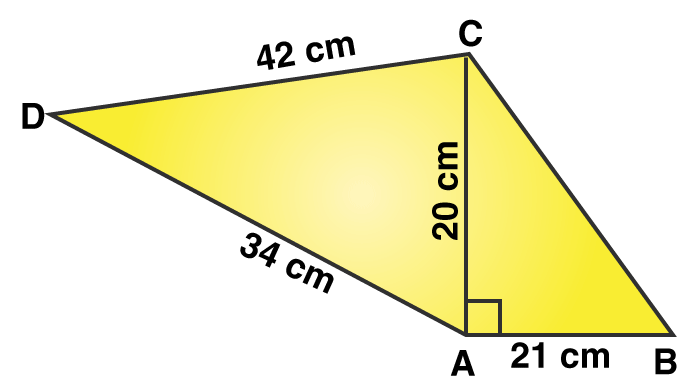Solution:

Consider △ BAC

Using the Pythagoras theorem

BC2 = AC2 + AB2

By substituting the values

BC2 =202 + 212

On further calculation

BC2 = 400 + 441

BC2 = 841

By taking out the square root

BC = √841

So we get

BC = 29cm

We know that

Perimeter of quadrilateral ABCD = AB + BC + CD + AD

By substituting the values

Perimeter = 21 + 29 + 42 + 34

Perimeter = 126cm

We know that area of △ ABC = ½ × b × h

It can be written as

Area of △ ABC = ½ × AB × AC

By substituting the values

Area of △ ABC = ½ × 21 × 20

On further calculation

Area of △ ABC = 210 cm2

Consider △ ACD

We know that AC = 20cm, CD = 42cm and AD = 34cm

It can be written as a = 20cm, b = 42cm and c = 34cm

So we get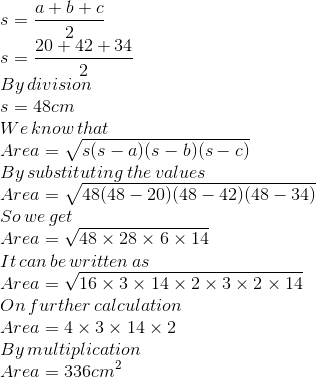So the area of quadrilateral ABCD = Area of △ ABC + Area of △ ACD

By substituting the values

Area of quadrilateral ABCD = 210 + 336 = 546 cm2

Therefore, the perimeter is 126cm and the area is 546 cm2.

24. Find the area of the quadrilateral ABCD in which BCD is an equilateral triangle, each of whose sides is 26cm, AD = 24cm and ∠ BAD = 90o. Also, find the perimeter of the quadrilateral. (Given, √3 = 1.73.)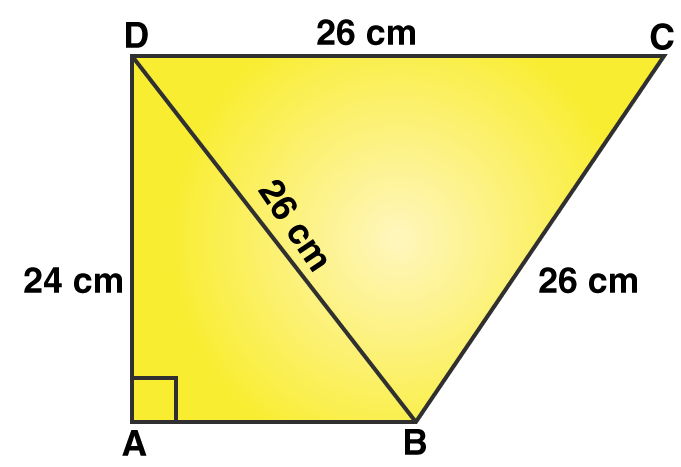Solution:

In △ ABD

Using the Pythagoras theorem

By substituting the values

262 =AB2 + 242

On further calculation

AB2 = 676 – 576

By subtraction

AB2 = 100

By taking out the square root

AB = √100

So we get

Base = AB = 10cm

We know that area of △ ABD = ½ × b × h

By substituting the values

Area of △ ABD = ½ × 10 × 24

On further calculation

Area of △ ABD = 120 cm2

We know that the area of △ BCD = √3/4 a2

By substituting the values

Area of △ BCD = (1.73/4) (26)2

So we get

Area of △ BCD = 292.37 cm2

So we get area of quadrilateral ABCD = Area of △ ABD + Area of △ BCD

By substituting the values

Area of quadrilateral ABCD = 120 + 29237

Area of quadrilateral ABCD = 412.37 cm2

The perimeter of quadrilateral ABCD = AB + BC + CD + DA

By substituting the values

Perimeter = 10 + 26 + 26 + 24

So we get

Perimeter = 86cm

Therefore, the area is 412.37 cm2 and perimeter is 86cm.

25. Find the area of a parallelogram ABCD in which AB = 28 cm, BC = 26 cm and diagonal AC = 30 cm.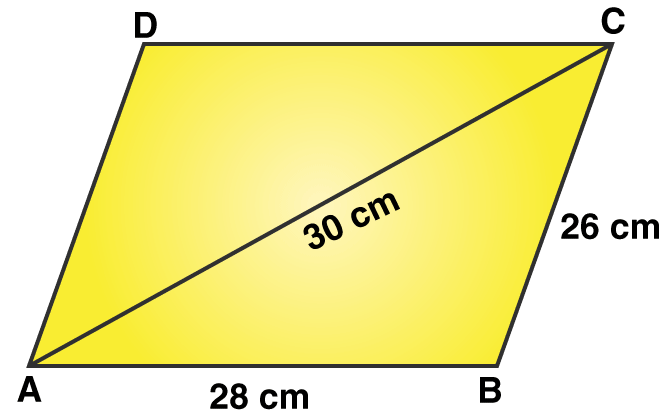Solution:

In △ ABC

Take a = 26cm, b = 30cm and c = 28cm

So we get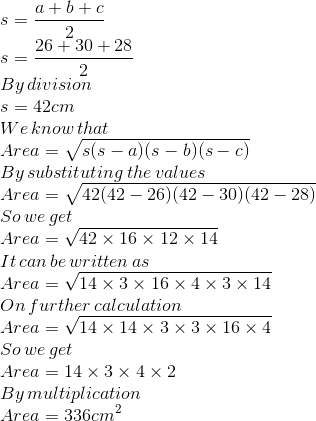We know that the diagonal divides the parallelogram into two equal area

So the area of parallelogram ABCD = Area of △ ABC + Area of △ ACD

It can be written as

Area of parallelogram ABCD = 2(Area of △ ABC)

By substituting the values

Area of parallelogram ABCD = 2 (336)

By multiplication

Area of parallelogram ABCD = 672 cm2

Therefore, the area of parallelogram ABCD is 672 cm2.

26. Find the area of a parallelogram ABCD in which AB = 14cm, BC = 10cm and AC = 16cm. (Given, √3 = 1.73.)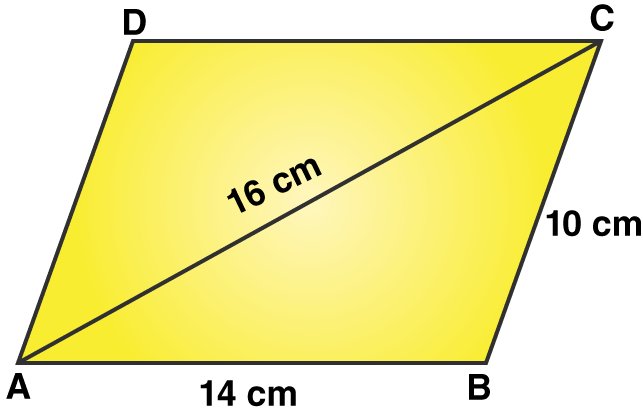Solution:

In △ ABC

Take a = 10cm, b = 16cm and c = 14cm

So we get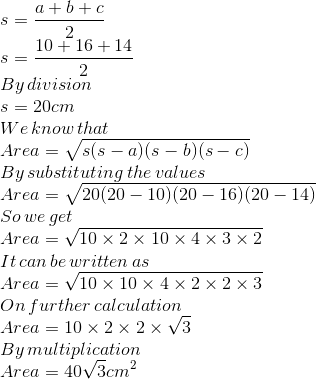We know that a diagonal in a parallelogram divides it into two equal area

So we get

Area of parallelogram ABCD = Area of △ ABC + Area of △ ACD

It can be written as

Area of parallelogram ABCD = 2(Area of △ ABC)

By substituting the values

Area of parallelogram ABCD = 2 (40 √3)

So we get

Area of parallelogram ABCD = 80√3

By substituting √3

Area of parallelogram ABCD = 80 (1.73)

So we get

Area of parallelogram ABCD = 138.4 cm2

Therefore, the area of parallelogram ABCD is 138.4 cm2.

27. In the given figure, ABCD is a quadrilateral in which diagonal BD = 64cm, AL ⊥ BD and CM ⊥ BD such that AL = 16.8 cm and CM = 13.2 cm. Calculate the area of the quadrilateral ABCD.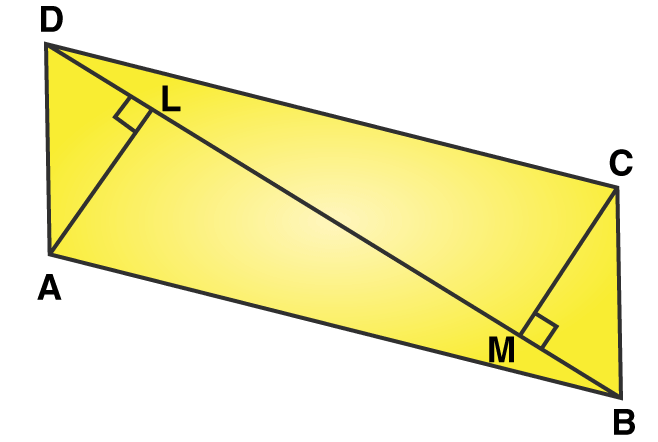Solution:

We know that

Area of △ ABD = ½ × b × h

It can be written as

Area of △ ABD = ½ × BD × AL

By substituting the values

Area of △ ABD = ½ × 64 × 16.8

On further calculation

Area of △ ABD = 537.6 cm2

We know that

Area of △ BCD = ½ × b × h

It can be written as

Area of △ BCD = ½ × BD × CM

By substituting the values

Area of △ BCD = ½ × 64 × 13.2

On further calculation

Area of △ BCD = 422.4 cm2

So we get area of quadrilateral ABCD = Area of △ ABD + Area of △ BCD

By substituting the values

Area of quadrilateral ABCD = 537.6 + 422.4

Area of quadrilateral ABCD = 960 cm2

Therefore, the area of quadrilateral ABCD is 960 cm2.

28. The area of a trapezium is 475 cm2 and its height is 19cm. Find the lengths of its two parallel sides if one side is 4cm greater than the other.

Solution:

Consider x cm as the smaller side of the trapezium

So the larger parallel can be written as (x + 4) cm

We know that the area of trapezium = ½ × sum of parallel sides × height

By substituting the values

475 = ½ × (x + (x + 4)) × 19

On further calculation

25 = ½ × (2x + 4)

So we get

50 = 2x + 4

By subtraction

2x = 50 – 4

2x = 46

By division

x = 23cm

So we get Larger parallel side (x + 4) = 23 + 4 = 27cm

Therefore, the length of two parallel sides is 23cm and 27cm.

29. In the given figure, a △ ABC has been given in which AB = 7.5 cm, AC = 6.5 cm and BC = 7cm. On base BC, a parallelogram DBCE of the same area as that of △ ABC is constructed. Find the height DL of the parallelogram.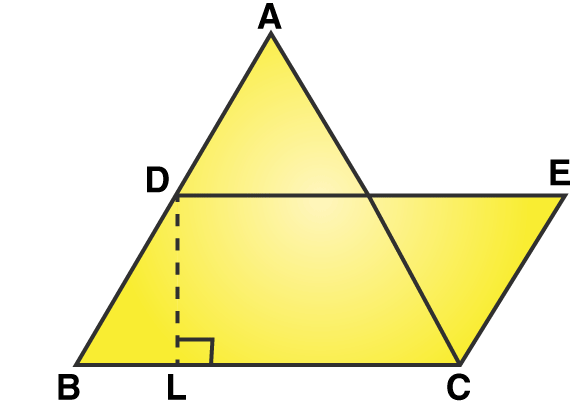Solution:

Consider △ ABC

It is given that AB = 7.5cm, BC = 7cm and AC = 6.5cm

Take a = 7.5cm, b = 7cm and c = 6.5 cm

So we get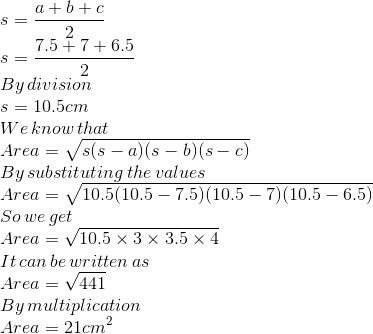We know that the area of parallelogram DBCE = Area of △ ABC

It can be written as

BC × DL = 21

By substituting the values

7 × DL = 21

By division

DL = 3cm

Therefore, the height DL of the parallelogram is 3cm.

30. A field is in the shape of a trapezium having parallel sides 90 m and 30 m. These sides meet the third side at right angles. The length of the fourth side is 100 m. If it costs ₹ 5 to plough 1m2 of the field, find the total cost of ploughing the field.

Solution: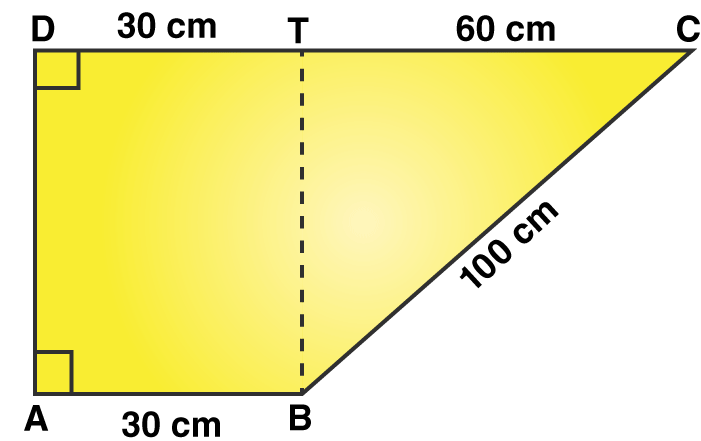Construct BT parallel to CD

Consider △ BTC

Using the Pythagoras theorem

BC2 = BT2 + CT2

By substituting the values

1002 =BT2 + 602

On further calculation

BT2 = 10000 – 3600

By subtraction

BT2 = 6400

By taking out the square root

BT = √6400

So we get

We know that

Area of field = Area of trapezium ABCD

So we get

Area of trapezium ABCD = ½ × Sum of parallel sides × height

It can be written as

Area of trapezium ABCD = ½ × (AB + CD) × AD

By substituting the values

Area of trapezium ABCD = ½ × (30 + 90) × 80

On further calculation

Area of trapezium ABCD = 120 × 40

By multiplication

Area of trapezium ABCD = 4800 m2

It is given that the cost of ploughing 1m2 is ₹ 5

So the cost of ploughing 4800 m2 = ₹ (5 × 4800)

The cost of ploughing 4800 m2 = ₹ 24000

Therefore, the total cost of ploughing the field is ₹ 24000.

31. A rectangular plot is given for constructing a house, having a measurement of 40m long and 15m in the front. According to the laws, a minimum of 3m wide space should be left in the front and back each and 2m wide space on each of the other sides. Find the largest area where house can be constructed.

Solution:

It is given that the length of rectangular plot = 40m

Width = 15m

By keeping 3m wide space both in front and back

The length becomes = 40 – 3 – 3 = 34m

By keeping 2m wide space both the sides

Width = 15 – 2 – 2 = 11m

So the largest area where the house can be constructed = 34 × 11 = 374 m2

Therefore, the largest area where the house can be constructed is 374 m2.

32. A rhombus-shaped sheet with perimeter 40cm and one diagonal 12cm, is painted on both sides at the rate of ₹5 per cm2. Find the cost of painting.

Solution: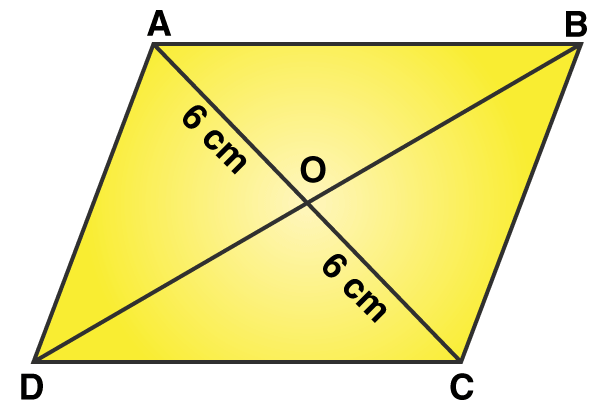Consider ABCD as the rhombus shaped sheet

It is given that the perimeter = 40cm

It can be written as

4 (side) = 40cm

So we get

Side = 10cm

We get

AB = BC = CD = AD = 1cm

Consider the diagonal AC = 12cm

We know that the diagonals of a rhombus bisect each other at right angles

So we get

AO = OC = 6cm

Consider △ AOD

Using the Pythagoras theorem

By substituting the values

102 =OD2 + 62

On further calculation

OD2 = 100 – 36

By subtraction

OD2 = 64

By taking out the square root

OD = √64

So we get

OD = 8cm

We know that

BD = 2OD

So we get

BD = 2(8)

BD = 16cm

We know that

Area of rhombus ABCD = ½ (Product of diagonals)

It can be written as

Area of rhombus ABCD = ½ × AC × BD

By substituting the values

Area of rhombus ABCD = ½ × 12 × 16

By multiplication

Area of rhombus ABCD = 96 cm2

It is given that the cost of painting is ₹5 per cm2

So the cost of paining both sides of rhombus = ₹5 × (96 + 96)

We get

The cost of painting both sides of rhombus = ₹5 × 192 = ₹960

Therefore, the cost of painting is ₹960.

33. The difference between the semiperimeter and the sides of a △ ABC are 8cm, 7cm and 5cm respectively. Find the area of the triangle.

Solution:

Consider a, b and c as the sides of a triangle and s as the semi perimeter

So we get

s – a = 8cm

s – b = 7cm

s – c = 5cm

We know that

(s – a) + (s – b) + (s – c) = 8 + 7 + 5

On further calculation

3s – (a + b + c) = 20

We know that a + b + c = 2s

3s – 2s = 2

So we get

s = 20

By substituting the value of s

a = s – 8 = 20 – 8 = 12cm

b = s – 7 = 20 – 7 = 13cm

c = s – 5 = 20 – 5 = 15cm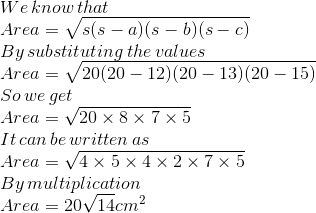Therefore, the area of the triangle is 20√14 cm2.

34. A floral design on a floor is made up of 16 tiles, each triangular in shape having sides 16cm, 12cm and 20cm. Find the cost of polishing the tiles at ₹1 per sq cm.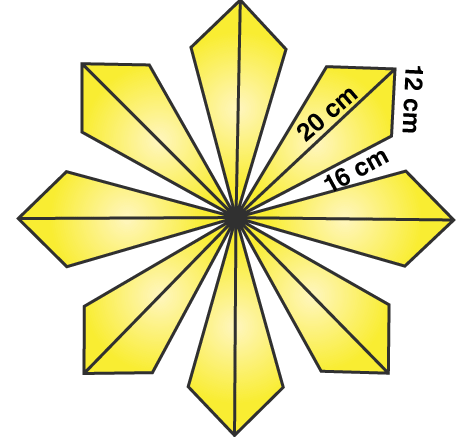Solution:

Consider a = 16cm, b = 12cm and c = 20cm

So we get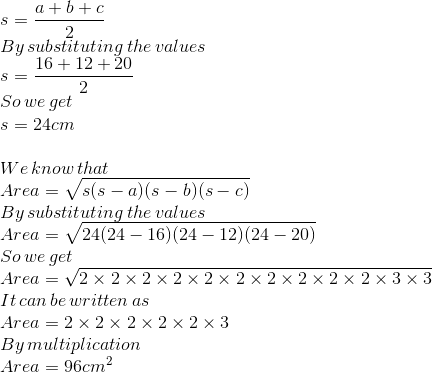So the area of one tile = 96cm2

The area of 16 triangular tiles = 96 (16) = 1536 cm2

It is given that the cost of polishing the tiles per sq cm = ₹1

So the cost of polishing all the tiles = ₹ (1 × 1536) = ₹ 1536

Therefore, the cost of polishing the tiles is ₹ 1536.

35. An umbrella is made by stitching 12 triangular pieces of cloth, each measuring (50cm × 20cm × 50cm). Find the area of the cloth used in it.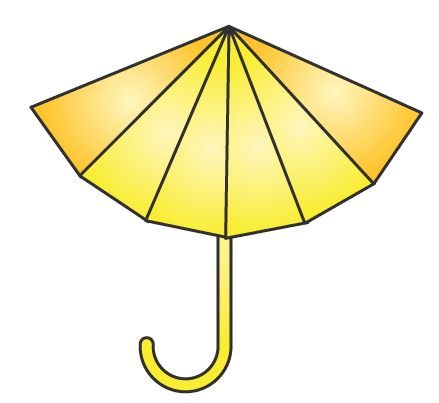Solution:

Consider a = 50cm, b = 20cm and c = 50cm

So we get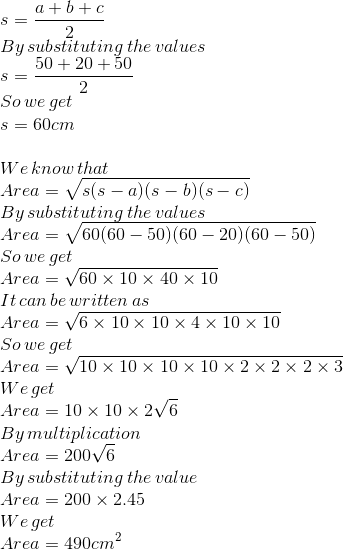So the area of one piece of cloth = 490 cm2

We know that the area of 12 pieces = 12 (490)

By multiplication

The area of 12 pieces = 5880 cm2

Therefore, the area of cloth used in it is 5880 cm2.

36. In the given figure, ABCD is a square with diagonal 44cm. How much paper of each shade is needed to make a kite given in the figure.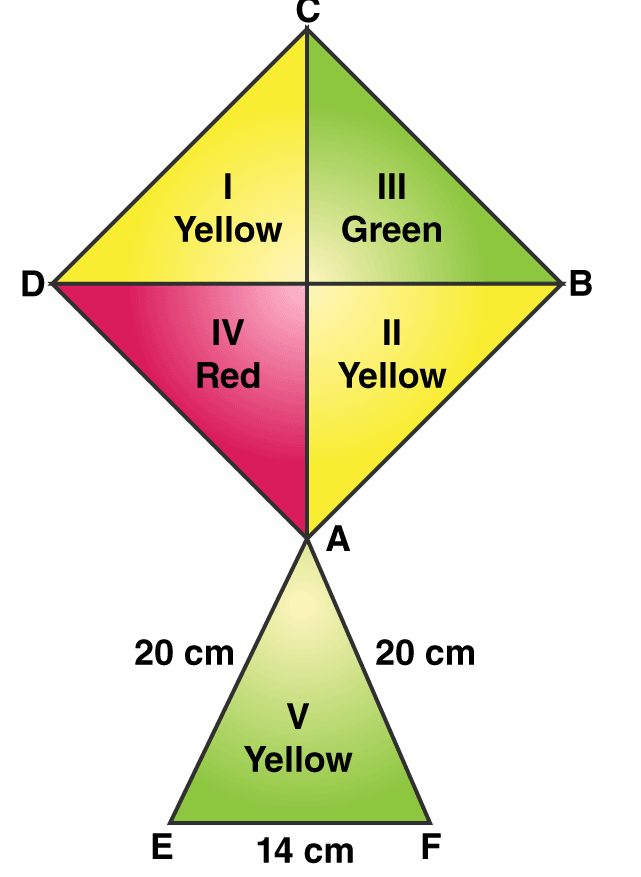Solution:

We know that

Area of square sheet ABCD = ½ × diagonal2

By substituting the value

Area of square sheet ABCD = ½ × 44 × 44 = 968 cm2

From the figure we know that the

Area of yellow sheet = Area of region I + Area of region II

It can be written as

Area of yellow sheet = ½ × Area of square sheet ABCD

By substituting the value

Area of yellow sheet = ½ × 968 = 484 cm2

From the figure we know that the

Area of red sheet = Area of region IV

It can be written as

Area of red sheet = 1/4 × Area of square sheet ABCD

By substituting the value

Area of red sheet = 1/4 × 968 = 242 cm2

Consider △ AEF

We know that AE = 20cm, EF = 14cm and AF = 20cm

Consider a = 20cm, b = 14cm and c = 20cm

So we get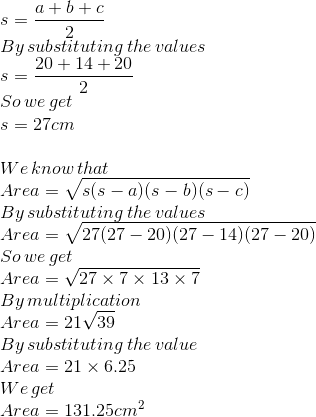From the figure we know that

Area of green sheet = Area of region III + Area of region V

It can be written as

Area of green sheet = ¼ × Area of square sheet ABCD + 131.25

By substituting the values

Area of green sheet = ¼ × 968 + 131.25

On further calculation

Area of green sheet = 242 + 131.25

Area of green sheet = 373.25 cm2

Therefore, the area of yellow sheet is 484 cm2, area of red sheet is 242 cm2 and the area of green sheet is 373.25cm2.

37. A rectangular lawn, 75m by 60m, has two roads, each road 4m wide, running through the middle of the lawn, one parallel to length and the other parallel to breadth, as shown in the figure. Find the cost of gravelling the roads at ₹ 50 per m2.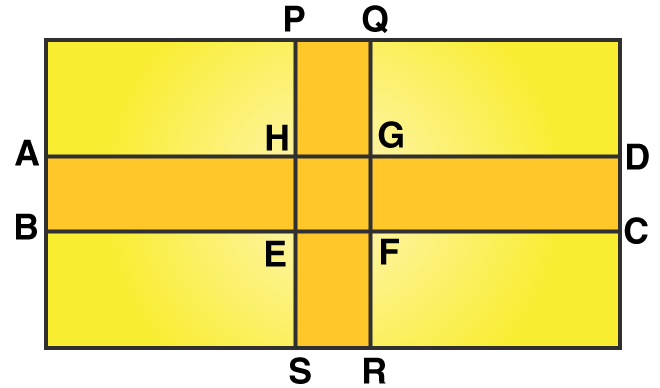Solution:

We know that for the road ABCD

It is given that length = 75m and breadth = 4m

We know that

Area of road ABCD = l × b

By substituting the values

Area of road ABCD = 75 × 4

So we get

Area of road ABCD = 300 m2

We know that for the road PQRS

It is given that length = 60m and breadth = 4m

We know that

Area of road PQRS = l × b

By substituting the values

Area of road PQRS = 60 × 4

So we get

Area of road PQRS = 240 m2

We know that for road EFGH

It is given that side = 4m

Area of road EFGH = side2

By substituting the value

Area of road EFGH = 42 = 16 m2

The total area of the road for gravelling = Area of road ABCD + Area of road PQRS – Area of road EFGH

By substituting the values

Total area of the road for gravelling = 300 + 340 – 16

So we get

Total area of the road for gravelling = 524 m2

It is given that the cost of gravelling the road = ₹ 50 per m2

So the cost of gravelling 524 m2 road = ₹ (50 × 524) = ₹ 26200

Therefore, the cost of gravelling the road is ₹ 26200.

38. The shape of the cross section of a canal is a trapezium. If the canal is 10m wide at the top, 6m wide at the bottom and the area of its cross section is 640m2, find the depth of the canal.

Solution:

It is given that the area of cross section = 640 m2

We know that

Length of top + Length of bottom = sum of parallel sides

It can be written as

Length of top + Length of bottom = 10 + 6 = 16m

We know that

Area of cross section = ½ × sum of parallel sides × height

By substituting the values

640 = ½ × 16 × height

So we get

Height = (640 × 2)/16

By division

Height = 80m

Therefore, the depth of the canal is 80m.

39. Find the area of a trapezium whose parallel sides are 11m and 25m long, and the nonparallel sides are 15m and 13m long.

Solution: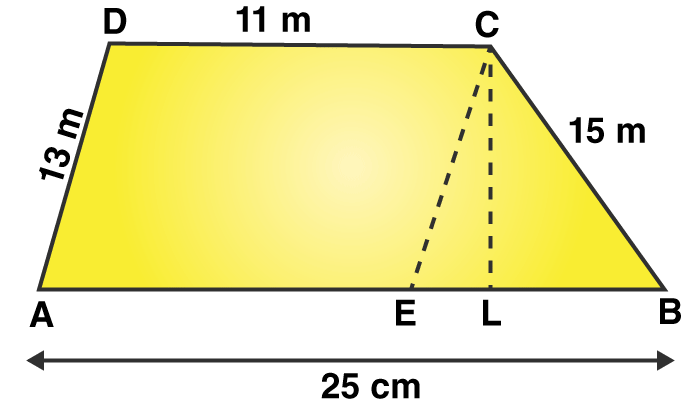From the point C construct CE || DA

We know that ADCE is a parallelogram having AE || DC and AD || EC with AD = 13m and D = 11m

It can be written as

AE = DC = 11m and EC = AD = 13m

So we get

BE = AB – AE

By substituting the values

BE = 25 – 11 = 14m

Consider △ BCE

We know that BC = 15m, CE = 13m and BE = 14m

Take a = 15m, b = 13m and c = 14m

So we get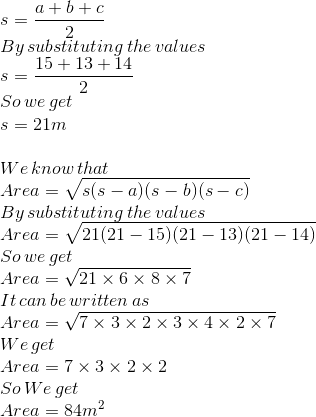We know that

Area of △ BCE = ½ × BE × CL

By substituting the values

84 = ½ × 14 × CL

On further calculation

84 = 7 × CL

By division

CL = 12m

We know that

Area of trapezium ABCD = ½ × sum of parallel sides × height

It can be written as

Area of trapezium ABCD = ½ × (AB + CD) × CL

By substituting the values

Area of trapezium ABCD = ½ × (11 + 25) × 12

On further calculation

Area of trapezium ABCD = 36 × 6

By multiplication

Area of trapezium ABCD = 216 m2

Therefore, the area of trapezium ABCD is 216 m2.

40. The difference between the lengths of the parallel sides of a trapezium is 8cm, the perpendicular distance between these sides is 24cm and the area of the trapezium is 312cm2. Find the length of each of the parallel sides.

Solution:

Consider x cm as the smaller parallel side

So the longer parallel side can be written as (x + 8) cm

It is given that height = 24cm

Area = 312 cm2

We know that

Area of trapezium = ½ × sum of parallel sides × height

By substituting the values

312 = ½ × (x + x + 8) × 24

On further calculation

312 = 12 × (2x + 8)

By division

2x + 8 = 26

So we get

2x = 26 – 8

By subtraction

2x = 18

By division

x = 9cm

So we get

x + 8 = 9 + 8 = 17cm

Therefore, the length of each of the parallel sides is 9cm and 17cm.

41. A parallelogram and a rhombus are equal in area. The diagonals of the rhombus measure 120m and 44m. If one of the sides of the parallelogram measures 66m, find its corresponding altitude.

Solution:

It is given that

Area of parallelogram = Area of rhombus

We can write it as

Base × altitude = ½ × product of diagonals

By substituting the values

66 × altitude = ½ × 120 × 44

On further calculation

66 × altitude = 60 × 44

So we get

Altitude = (60 × 44)/66

Altitude = 40 m

Therefore, the corresponding altitude is 40m.

42. A parallelogram and a square have the same area. If the sides of the square measure 40m and altitude of the parallelogram measures 25m, find the length of the corresponding base of the parallelogram.

Solution:

It is given that

Area of parallelogram = Area of square

We know that

Base × altitude = side2

By substituting the value

Base × 25 = 402

On further calculation

Base = (40 × 40)/25

So we get

Base = 64m

Therefore, the length of the corresponding base of the parallelogram is 64m.

43. Find the area of the rhombus one side of which measures 20cm and one of whose diagonals is 24cm.

Solution:

Consider ABCD as a rhombus with AC and BD as diagonals which intersect each other at O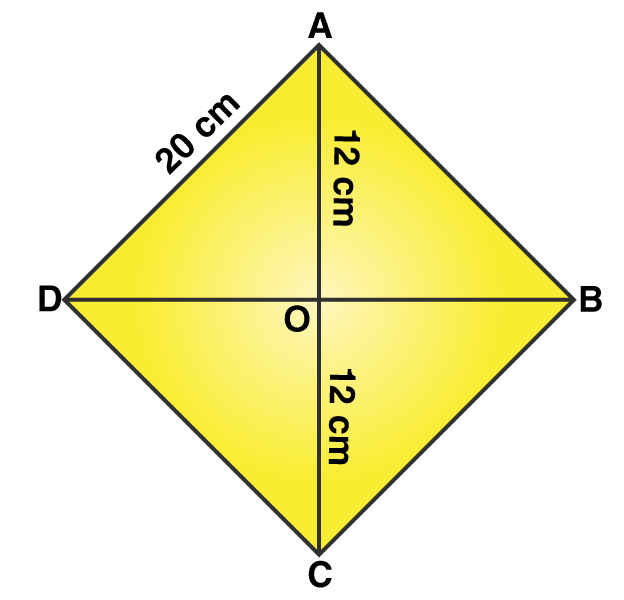The diagonals of a rhombus bisect at right angles

Consider △ AOD

Using the Pythagoras theorem

By substituting the values

202 =OD2 + 122

On further calculation

OD2 = 400 – 144

By subtraction

OD2 = 256

By taking out the square root

OD = √256

So we get

OD = 16cm

We know that BD = 2OD

So we get

BC = 2 (16) = 32cm

We know that

Area of rhombus ABCD = ½ × AC × BD

By substituting the values

Area of rhombus ABCD = ½ × 24 × 32

On further calculation

Area of rhombus ABCD = 384 cm2

Therefore, the area of rhombus ABCD is 384 cm2.

44. The area of a rhombus is 480cm2, and one of its diagonals measures 48cm. Find

(i) the length of the other diagonal,

(ii) the length of each of its sides, and
(iii its perimeter.

Solution:

(i) It is given that

Area of rhombus = 480 cm2

It can be written as

½ × diagonal 1 × diagonal 2 = 480

So we get

½ × 48 × diagonal 2 = 480

On further calculation

Diagonal 2 = 20cm

(ii) Consider AC = 48cm and BD = 20cm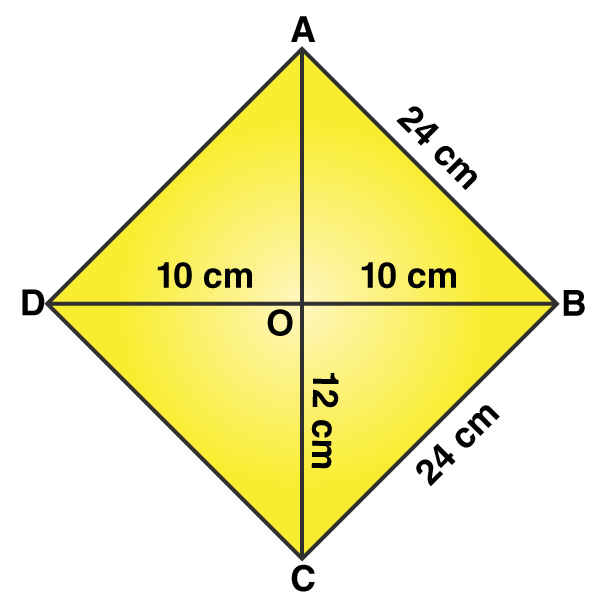The diagonals of a rhombus bisect at right angles

Consider △ AOD

Using the Pythagoras theorem

By substituting the values

On further calculation

By taking out the square root

So we get

We know that AD = BC = CD = AD = 26cm

Therefore, the length of each side of rhombus is 26cm.

(iii) We know that

Perimeter of a rhombus = 4 (side)

By substituting the value

Perimeter of a rhombus = 4 (26)

So we get

Perimeter of a rhombus = 104 cm

### RS Aggarwal Solutions for Class 9 Maths Chapter 14: Areas of Triangles and Quadrilaterals

Chapter 14, Areas of Triangles and Quadrilaterals, has 1 exercise with answers in PDF format which can be downloaded by the students to improve their exam preparation. Some of the topics which are covered in RS Aggarwal Solutions Chapter 14 are:

• Introduction
• Area of Triangles